﻿
С  О  З  Н  А  Н  И  Е    И    Б  Е  С  П  Р  Е  Д  Е  Л  Ь  Н  О  С  Т  ЬПорталКультура 11 дек 2023, 17:27УРАЛ: UTC + 5 часов
﻿
PERSONAL     PAGES     OF     ANDREJ    PAVLOVICH    PUZIKOV

 English Русский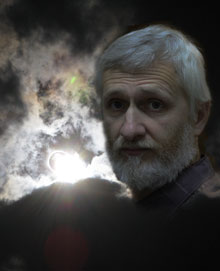Mechanics of Limitlessness

In this work, I present to readers a theoretical model of the formation of physical reality, based on the postulate of the existence of Limitlessness, that which has no limits and stands above all the root causes, since it includes them all. Random fluctuation in Limitlessness gives rise to the process of its multiple projections, going in growing cycles. The zero cycle divides Limitlessness into four identical parts – four dimensions. The eighth cycle in this sequence is our physical Universe, each of whose four dimensions consists of 2128 parts. This is the defining integer of our Universe, its quantum characteristic - the number of state quanta that make up its full cycle. This is the only new and previously unknown to science number that is included in the formulas of this model. Nevertheless this number alone turned out to be enough to reduce to each other the formulas of all basic physical constants, the sizes of elementary particles, atoms and the Universe, to connect the mass of elementary particles with their sizes, to calculate the full cycle of the Universe and its part passed, to get the exact value of the decay time of a free neutron, theoretical value of the electron and proton mass, corresponding to the experimental one, to explain the nuclear forces, the phenomenon of inertia, the constancy of the speed of light and its independence from the motion of the frame of reference, magnetic properties of electron and atom and much more.© Any reprint or duplication only with the consent of the author. It is permitted to make copies for personal use.
﻿
 English Русский

Andrej PUZIKOV

Publication Date
of eighth edition
20.11.2023

Mechanics of Limitlessness
Eighth edition

Preface to the eighth edition

Content

Continuation of the study once again requires correction of some previously made formulations and conclusions.

It is impossible to create an absolutely perfect and correct theory. The scientific process is not an detection in which physicists, as detectives, reveal the hidden actions of nature. Nature is inexhaustible, and the creation of scientific models describing it is much more originative than protocol fixation.

Any describing model, like any reflection, is always poorer than the original, and there will always be the possibility of deepening and improving it. Moreover, at this stage, I have to move practically alone, without being able to get even a simple critique from serious physicists.

The theory I propose is too radically new, and if I turn out to be right, and I have no doubt about it, a huge number of scientific works will turn into unnecessary trash. But this is how the scientific process works, and in the end nothing is wasted.

Unfortunately, modern fundamental science is increasingly similar to religious pharisaism, when faith in traditional approaches, illusion of objectiveness and authorities prevails over the desire for knowledge and common sense.

But the course of evolution will sooner or later do its job, and in the meantime I will being do mine.

Preface to the seventh edition

Content

As the theoretical research progresses, it becomes necessary to seriously correct the conclusions made earlier, to correct errors that are inevitable in such a new and unexplored direction of science, and to add new aspects of the research.

But it is precisely thanks to peripheral errors that the correctness of the main path is strengthened, when each error found does not lead to the destruction of the main building of logic, but, on the contrary, makes it more harmonious and opens up a number of new, previously unnoticed valuable discoveries.

In this seventh edition, the book has been completely revised. In addition to correcting some incorrect and sometimes erroneous logical constructions to explain the found correct formulas, the author set the task to make the reader's perception of the material as easy as possible. The presentation of the material, as far as possible, is adapted to the terminology accepted in modern physical science. The use of new terms has been reduced to the maximum, giving them a systematic, more easy-to-remember character. For the same purpose, some denotations of physical quantities have also been changed.

Foreword

Content

How did it all start?

According to the theories of modern physics based on experimental data, our Universe was born as a result of the "Big Bang". However, can the Big Bang be considered the beginning of everything? The very concept of an explosion implies the existence of conditions for this explosion, in this case, a superdense state of matter. However how did these conditions come about?

Physical science claims to reflect objective reality, while it itself was formed according to the laws of this reality and is its consequence. All the laws of physics were induced from physical experience, which, in turn, is based on the organs of perception of a person and his ability to form models in his internal representation that reflect the logic of the physical world.

Thus, in the process of its formation, physical science is constrained by the rigid limits of the possibilities of human representation, which, in turn, are shaped by the physical conditions of being. Those are these human representations that dictate the production of certain physical practice and experiments. It turns out an enclosed circle.

Mathematics allows you to expand the scope of this circle somewhat, to create models that go beyond the scope of the subject representation, for example: multidimensional spaces. However, in essence, this is just an illusion of going beyond the usual three-dimensional subject representations. The mathematical addition of one or more additional dimensions to three-dimensional space does not change the logic of this space, in other words, it does not go beyond the logic of human spatial representation. Even the introduction of imaginary numbers into formulas, in fact, is just a way to tie the explanation of physical processes to a two-dimensional number series - all the same habit of geometric representation in space, that can be curved, according to Einstein, however at the same time it remains a single space - some kind of original essence, a kind of "god", in which physicists firmly believe, and which was erected on the site of the once overthrown other "god" - ether.

The situation is even sadder with the concept of physical time. The representation of time in modern physics as a continuous, arbitrarily divisible spatial dimension, on the one hand, amuses violent human imagination, but on the other hand, leads science into a dead end of age-old habits of perception.

However, if we try to go beyond all these limits of human ideas and mathematical models? If we take into account a simple fact - any limits and restrictions are the result of some process that forms them. However the process of forming one or another framework itself occurs within the framework defined for it by some previous process.

The question is quite logical: did this process of the consistent formation of frames and restrictions, the consequence of which is our physical world, have a beginning?

The solution of this issue by the methods of modern physical science involves the induction of the root cause from the effect - our physical existence. Perhaps this path will eventually give the necessary answer. However, now, science cannot go further than some indefinite concept of superdense matter that gave rise to the Big Bang.

Nevertheless there is another way - not to induce a cause from an effect, but to assume the existence of the root cause that is not limited by any framework or limits, and on the basis of strict logic to build the theoretical model of what should become the consequence of this root cause.

In this work, I present to readers a theoretical model of the formation of physical reality, based on the postulate of the existence of Limitlessness, that which has no limits and stands above all the root causes, since it includes them all. Random fluctuation in Limitlessness gives rise to the process of its multiple projections, going in growing cycles. The zero cycle divides Limitlessness into four identical parts – four dimensions. The eighth cycle in this sequence is our physical Universe, each of whose four dimensions consists of 2128 parts. This is the defining integer of our Universe, its quantum characteristic - the number of state quanta that make up its full cycle. This is the only new and previously unknown to science number that is included in the formulas of this model. Nevertheless this number alone turned out to be enough to reduce to each other the formulas of all basic physical constants, the sizes of elementary particles, atoms and the Universe, to connect the mass of elementary particles with their sizes, to calculate the full cycle of the Universe and its part passed, to get the exact value of the decay time of a free neutron, theoretical value of the electron and proton mass, corresponding to the experimental one, to explain the nuclear forces, the phenomenon of inertia, the constancy of the speed of light and its independence from the motion of the frame of reference, magnetic properties of electron and atom and much more.

The advantage of this model is not only that it provides an explanation for all physical phenomena, explains the dimensionality of space and time, but also reduces all physical laws to a single mechanical process of interaction of projections into the physical space of elementary localizations that are completely identical to the localization of the Universe. Full projections of elementary localizations are free neutrons, and partial projections are all other elementary particles.

In addition, the physical space itself is nothing more than a relative space of conditions in projection into the frame of reference associated with our planet. These or other conditions determine different spaces, which may be completely indefinite with respect to each other, or be bounding by general conditions.

Time, as a sequence of integral and indivisible quanta of state of the Universe in the relativity of the local frame of reference associated with our planet, displacing at the speed of light along one of the dimensions of space, instead of the principle of continuous flow of time accepted in traditional physics, is one of the main differences between this model and traditional ones.

-------------------

1. The Limitlessness

Content

We put the following postulate as the basis for further theoretical research:

Postulate:

There is something that has no restrictions and limits.

Let us call it Limitlessness:

Definition (d1.1):

The Limitlessness is that which has no restrictions.

Let us prove the main theorem of Limitlessness.

Theorem (t1):

Limitlessness, or what has no restrictions, is all-encompassing.

Proof:

Let us assume that there is something that is not covered by Limitlessness. In this case, a restriction is imposed on Limitlessness, expressed in the fact that Limitlessness does not cover this something. This contradicts the definition of Limitlessness. Consequently, the theorem can be considered proved.

The potential property of unlimited possibility of division into parts necessarily follows from the property of all-encompassing. Let us call this the principle of divisibility.

Definition (d1.2):

The principle of divisibility is the potential property of Limitlessness to be unlimitedly divided into parts.

In this connection, we prove the following theorems:

Theorem (t2):

Any part of Limitlessness is identical with the whole of Limitlessness.

Proof:

If in any part of Limitlessness there are some restrictions, it follows that Limitlessness itself is restricted by these restrictions in this part of itself, which contradicts the definition of Limitlessness. Therefore, parts of Limitlessness cannot have restrictions, and thus is completely identical to all Limitlessness in accordance with the definition. The theorem can be considered proved.

Conclusion (c1.1):

It follows from theorem (t2) that all parts of Limitlessness are parts of any part of it.

Theorem (t3):

The Limitlessness is symmetrical with respect to any parts of itself.

Proof:

If the Limitlessness is not symmetrical with respect to one of its parts, it will mean that Limitlessness is divided into two parts that are not identical to each other, which contradicts Theorem (t2). The theorem can be considered proved.

Conclusion (c1.2):

It follows from theorem (t2) and theorem (t3) that any part of Limitlessness is symmetric with respect to it and any other part of it.

2. localization

Content

As we have already noted in the first chapter, Limitlessness, as a comprehensive phenomenon, includes the principle of divisibility. However, the potential for division into parts does not mean the existence of some particular division.

On the other hand, from the principle of the absence of restrictions, it necessarily follows that there is a one hundred percent probability of localizing a certain part in Limitlessness, as a random fluctuation..

A random fluctuation is a part of Limitlessness and, according to theorem (t2), should be completely identical to it. However, the very local singling out of a part within the whole necessarily imposes a limiting condition, expressed in the fact that the singled out part is smaller than the whole.

Since restrictions cannot be imposed on Limitlessness itself, these limiting conditions are reflected only in the relativity of the limiting process itself, as a frame of reference.

Conclusion (c2.1):

Any restriction in Limitlessness can exist only in the frame of reference associated with this restriction.

In accordance with the conclusion (c1.1)), a random fluctuation, as a localized part of Limitlessness, must include the entire Limitlessness, but with the condition of its own internal restrictions. Thus, the localized part represents the state of Limitlessness under the conditions of its own restrictions.

Definition (d2.1):

The state of Limitlessness is the Limitlessness under conditions of internal relative self-restriction.

Before the occurrence of a random fluctuation, it did not exist, and, therefore, there was no localized state of Limitlessness. Thus, random fluctuation gives rise to the process of changing states of Limitlessness.

Conclusion (c2.2):

Random fluctuation gives rise to the process of changing states of Limitlessness.

Conclusion (c2.3):

The restricting conditions that form the local singling out of a part in Limitlessness are: the presence of the moment of the beginning of the process and its local position in Limitlessness.

By its act of singling out, a random fluctuation in its own relativity divides the state of Limitlessness into two identical parts: itself, as a material phenomenon, and Limitlessness, as a potential substance in which it has been singled out.

This complex phenomenon of two identical parts of Limitlessness, restricted by one restriction, acquires its objective existence.

Let us call it localization and give the following definitions:

Definition (d2.2):

Localization is a part of Limitlessness with a restricting condition imposed on it of the local singling out of part in internal relativity of this condition.

Considering that the localization process takes place exclusively under its own restricting condition, we give the following definition:

Definition (d2.3):

The fundamental frame of reference is the frame of reference associated with the restricting process that forms localization.

Everything that, one way or another, is reflected in the fundamental frame of reference is inside the localization, and nothing outside of localization can be reflected in the fundamental frame of reference. Thus, all processes within localization are determined by the relative restriction forming it and exist exclusively within this restriction.

Let us prove another important theorem.

Theorem (t4):

Each subsequent act of imposing restriction conditions in their own relativity of these conditions occurs in already existing conditions and cannot change them.

Proof:

Any restricting condition reduces the entire Limitlessness into conditions completely closed by this restriction. Thus, any new restricting condition imposed within the previous restricting condition is imposed on the Limitlessness in reduction to the previous condition in its closed restriction and cannot go beyond its restriction, that is, change it. The theorem can be considered proved.

Let us give the following definitions:

Definition (d2.4):

The material part of localization is the locally singled out part of Limitlessness that forms the localization.

Definition (d2.5):

The potential part of localization is the projection of Limitlessness into the relative restricting conditions of localization.

From the condition of restriction by one general restriction and the Conclusion (c1.1), the necessary conclusion follows:

Conclusion (c2.4):

The material part of localization is part of the potential part, and the potential part is part of the material part.

We can call this property a projection into each other, fig. 2.1.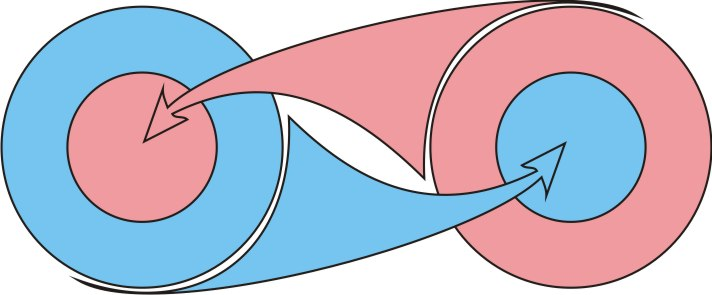Fig. 2.1

Definition (d2.6):

The projection of one part of Limitlessness into another is the property of this second part to include the first part as its own in the relativity of restriction conditions of itself.

Localization generated by a random fluctuation in Limitlessness, we will call primary.

The condition for the internal division of the primary localization into two parts in accordance with theorem (t2) according to the principle of the identity of the parts and the whole must be projected into each of its parts. Accordingly, the material and potential parts must be similarly divided within themselves into two identical parts, fig. 2.2.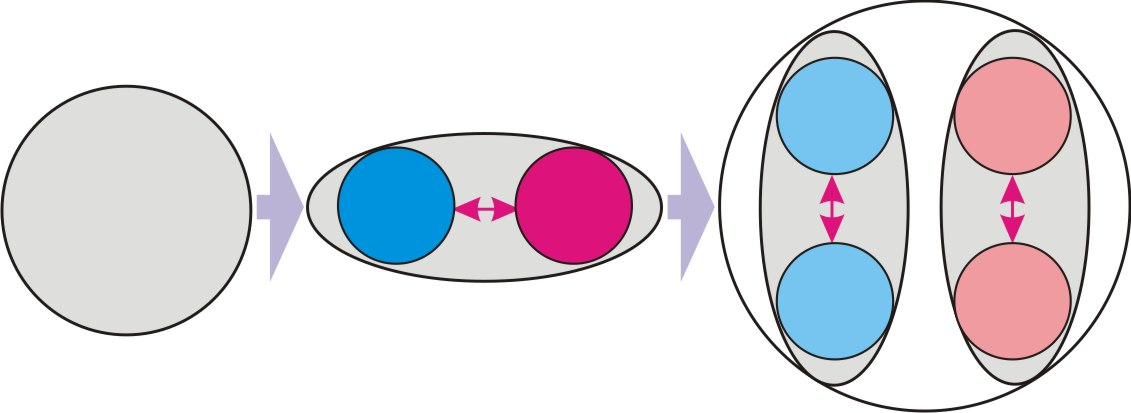Fig. 2.2.

Thus, the primary fluctuation in its internal relativity consists of four singled out parts. In accordance with the Conclusion (c1.1), all these parts are simultaneously parts of each of them.

At first glance, it may seem that it should follow from the principle of the identity of parts that each new part of the divide process must in turn be divided into two parts, and this process of divide with the allocation of new parts will be endless. However, it is not. The divide of each of the parts into two identical secondary parts does not mean the addition of new parts. A similar secondary part of the one part of the primary divide is projected into similar secondary part of second part of the primary divide, fig. 2.3a).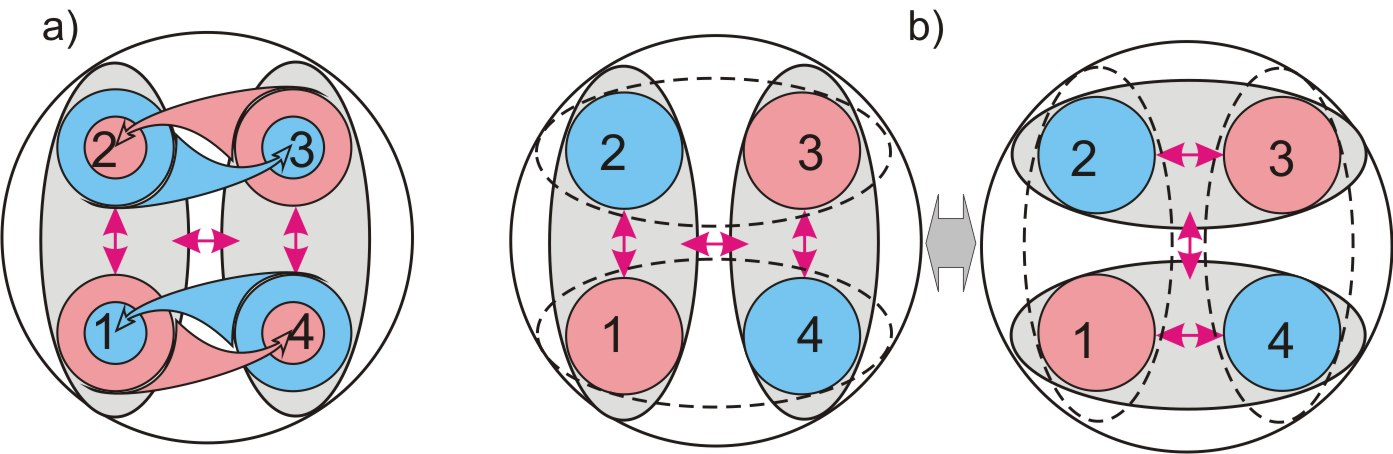Fig. 2.3.

This process of divide into two parts and mutual projection into each other is completely identical and symmetrical with respect to each of the four parts of the primary divide, fig. 2.3, b). In this case, all other parts are projected into each of them.

Thus, the process of singling out parts by dividing each of the parts into two parts closes on itself and ends with the singled out four identical parts that make up the primary localization.

Conclusion (c2.5):

Random fluctuation, as the primary act of singling out a part of Limitlessness, in its relativity causes the process of divide the Limitlessness into two parts, which necessarily ends with the singling out of four identical parts in it.

We give the following definitions:

Definition (d2.7):

The main parts of localization are the material part, which forms a local phenomenon and the potential part, which is Limitlessness in reduction to the limiting condition for the allocation of the material part.

Definition (d2.8):

The secondary parts of localization are four identical parts, singled out by the projection of the process of division into two parts into both main parts.

3. The sequence of localizations

Content

It is correct to consider processes that occur outside the scope of localization only in a frame of reference taken out of its limiting conditions:

Definition (d3.1):

An external frame of reference is a frame of reference taken out of the bounding framework of localization.

This frame of reference, in some convention, can be considered a "look from the Limitlessness".

In the external frame of reference, the entire primary localization is a localized material phenomenon.

Thus, similarly to random fluctuations, the entire complex primary localization is the material part of the new localization. Let us define the primary localization as a zero-order localization, and the one formed on its basis as a first-order localization.

Let us pay attention to the fact that the fundamental reference frame associated with the first-order localization is external with respect to the zero-order localization.

The differences between the material and potential parts of the primary localization, as well as the differences between its primary and secondary parts, exist only in the fundamental frame of reference associated with it.

ОWith respect to the fundamental reference frame associated with the first-order localization, all four singled out parts of the zero-order localization do not differ from each other in any way and, on the basis of theorem (t2) and Conclusion (c1.1), are completely identical.

Thus, the material part of first-order localization consists of four identical parts.

This condition of preliminary division of the material part into four identical parts in accordance with the theorem (t4) determines the process of formation of the first-order localization.

According to the principle of identity (theorem (t2)) the potential part of the first-order localization should similarly consist of four singled out identical parts, fig. 3.1, a).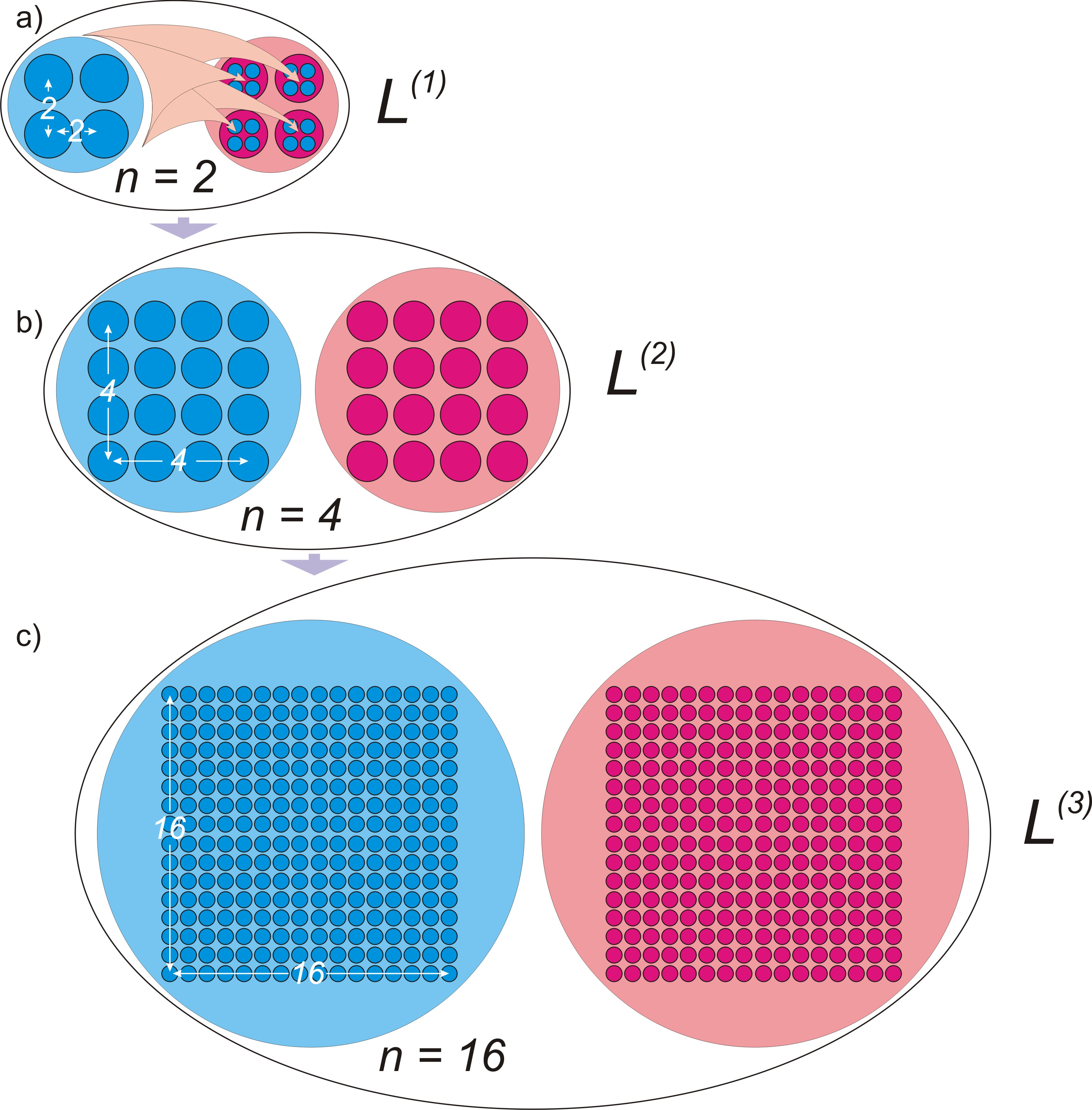Fig. 3.1.

And in accordance with the Conclusion (c1.1), all parts of the material part must be projected into each of these parts of the potential part. And vice versa, all parts of the potential part must be projected into each of the parts of the material part. It follows from these conditions that the total number of identical parts in a first-order localization is equal to the square of the number of identical parts in each of its main parts: 42 = 16.

This first-order localization with respect to the external reference frame is similarly the material part of the second-order localization, consisting of 16 identical parts, fig. 4b). The total number of identical parts in the second-order localization will be equal to 162 = 256.

Thus, as a result of random fluctuation in Limitlessness, a sequence of localizations is formed with a rapidly growing number of parts of internal divide.

Conclusion (c3.1):

The entire complex localization of the previous order represents the initial act of singling out the material part in the localization of the next order.

If we denote the number of parts in each of the four secondary parts of localization as n, then, accordingly, the number of parts in each main part will be equal to n2, and the total number of parts will be equal to n4.

Definition (d3.2):

The defining integer of localization is a positive integer n, equal to the number of identical parts into which each of the four secondary parts of localization is divided.

Conclusion (c3.2):

The material and potential parts of the localization with the defining integer n consist of n2 of their identical parts, and the total number of parts in the localization equal to n4.

In primary or zero order localization n = 1, in first order localization n = 2, in second order localization n = 4, in third order localization n = 16.

Conclusion (c3.3):

The defining integer of localization of the next order, starting from the localization of the first order, is equal to the square of the defining integer of localization of the previous order.

Thus, we can write down the formula for the defining integer of localization in the sequence of formation of localizations, starting from the localization of the first order:

n = 2(2k-1)  (3.1), where k is the order of localization.

Calculate the size of localizations of the first order of values k:

L(0):      n = 1

L(1):      n = 2

L(2):      n = 4

L(3):      n = 16

L(4):      n = 28 = 256

L(5):      n = 216 = 65536

L(6):      n = 232 = 4294967296

L(7):      n = 264 = 18446744073709551616

L(8):      n = 2128 = 3,40282366920938*1038

L(9):      n = 2256 = 1,15792089237316*1077

The logic of the process tells us that our physical Universe is a localization in Limitlessness and occupies a certain place in this sequence.

In the following chapters, on the basis of the complete coincidence of theoretical calculations with experimental physical data, we will prove that our Universe is an eighth-order localization with a defining integer n = 2128.

4. Dimensions of space

Content

In the fundamental frame of reference, the material part is restricted by the condition of local singled out and, as a result, does not coincide with the potential part. They are identical (theorem (t2)), but they do not coincide with each other. This principle of non-coincidence is identically projected into the secondary parts of localization.

Thus, all four secondary parts of localization do not coincide with each other. Herewith, they must be identical and, in accordance with the Conclusion (c1.1), each of them must include the other three with all their parts. At first glance, these conditions seem to contradict each other. But it is the presence of all these conditions that determines what we are accustomed to perceive as physical space. Let us consider it on the example of two-dimensional space.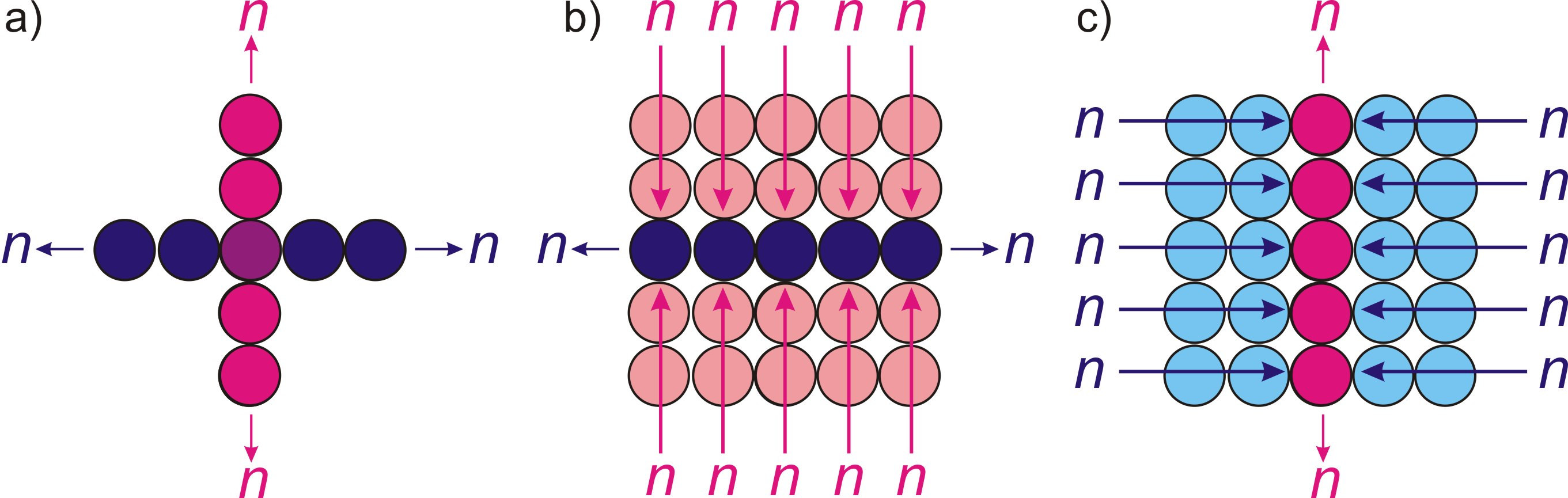Fig. 4.1.

On fig. 4.1, a) shows two completely identical and equal parts that do not coincide with each other. Herewith, each of them meets the condition of symmetry with respect to the other.

In accordance with the Conclusion (c1.1), all parts of one of these two parts are projected (definition (d2.6)) into each of the parts of the other part, fig. 4.1, b) and c).

In the case of localization, there are four such, projecting into each other and non-coinciding parts. Two of them are parts of the material part, the other two are parts of the potential parts. But, proceeding from the same Conclusion (c1.1), all of them must be projecting both into the material and potential part.

Definition (d4.1):

A dimension is each of the four secondary parts of localization.

The dimension, as an identical part of the localization, in accordance with the Conclusion (c1.2) must be symmetrical with respect to any of its parts and have no breaks, from which the conclusion follows:

Conclusion (c4.1):

In the absence of additional restrictions, the dimension is symmetrical with respect to any part of it and is closed on itself.

Conclusion (c4.2):

All parts of one dimension are projected into each part of another dimension.

Thus, the localization in its internal structure in the relativity of the fundamental frame of reference has four dimensions, and the number of parts in each of them is equal to its defining integer n.

This four-dimensional structure consists of two two-dimensional structures, which are the two main parts of the localization.

Conclusion (c4.3):

In the fundamental reference frame, localization is a four-dimensional structure formed by its two two-dimensional main parts projected into each other.

Conclusion (c4.4):

The number of parts in each of the four dimensions of localization is equal to its defining integer n.

The process of formation of the four-dimensional structure of each localization is initiated by the initial act of local singled out its material part. Another important conclusion follows from this:

Conclusion (c4.5):

The four-dimensional localization structure of each order is formed exclusively in the fundamental frame of reference associated with it and does not depend on the similar localization structure of the previous order.

Definition (d4.2):

A space is a set of static conditions that determine the interaction of parts of localization..

Conclusion (c4.6):

The space of localization is four-dimensional.

5. The process of compensating for broken symmetry and the localization cycle

Content

In relation to the fundamental reference frame (definition (d2.3)), the material part of the localization is asymmetrical relative to its potential part. This contradicts the condition of complete symmetry of the parts of Limitlessness relative to each other (conclusion (c1.2)). However, this contradiction exists only in a static situation. Localization is a dynamic process, and the static violation of symmetry in it is compensated by a dynamic process. Since localization is a consequence of the process of local singling out of a part of Limitlessness, compensation of broken symmetry should similarly be a process associated with acts of singling out of a part.

Conclusion (c5.1):

All processes in localization represent compensation for the violation of symmetry by the primary act of local singling out the material part.

The duration of the process of local singling out the material part of localization has no other comparative characteristics, except for the dimensional ratio of the material and potential parts of localization. Since the material part is less than the potential one, the process of singling out the material part must be completed before the process of singling out the entire localization is completed. This process of complete singling out the localization has no comparative characteristics in Limitlessness; accordingly, the probability of repeated singling out the material part under the same restricting conditions is equal to one.

Conclusion (c5.2):

The act of local singling out the material part is necessarily repeated in the potential part of the localization.

By the act of re-singling out the material part, one of the two dimensions of the potential part is selected, as a connecting line between the first and second act. In this case, the static violation of symmetry in localization is projected onto this randomly selected dimension.

Conclusion (c5.3):

As a result of the second act of singling out the material part, the static violation of symmetry in the localization is projected onto one randomly selected dimension of its potential part.

Thus, the second act of local singling out the material part occurs along the selected dimension.

Let's give the following definition:

Definition (d5.1):

The definite dimension is a dimension onto which a static symmetry breaking in localization is projected.

From the condition of identity of parts of dimension, the following conclusion follows:

Conclusion (c5.4):

The act of local singling out the material part along the selected dimension is repeated in each of its parts.

Since this process represents compensation for broken symmetry within localization, we will give it the appropriate name:

Definition (d5.2):

The process of compensating for broken symmetry is the sequential process of local singling out the material part within the localization.

The initial act of local singling out the material part determines the origin of reference on a certain dimension, and the second act of isolating determines the direction of movement of the process along it.

The second act of local singling out the material part of localization along the dimension is identical to the primary act of localization of the part in Limitlessness, from which the conclusion follows:

Conclusion (c5.5):

The second act of local singling out the material part of the localization along the selected dimension triggers in it the process of selecting two main and two secondary parts, identical to the process of selecting two main and two secondary parts in the localization by the primary act of singling out the material part.

This division of the selected dimension into four parts relative to the principle of movement along it is identically projected into all dimensions.

Conclusion (c5.6):

Regarding the principle of movement, each dimension is divided into two main parts, representing two directions of movement of the process along it, each of which is divided into two secondary parts - the right and left sides.

Let us note that this process of dividing the dimension into four parts closes on itself, similar to the primary process of division in localization, fig. 5.1.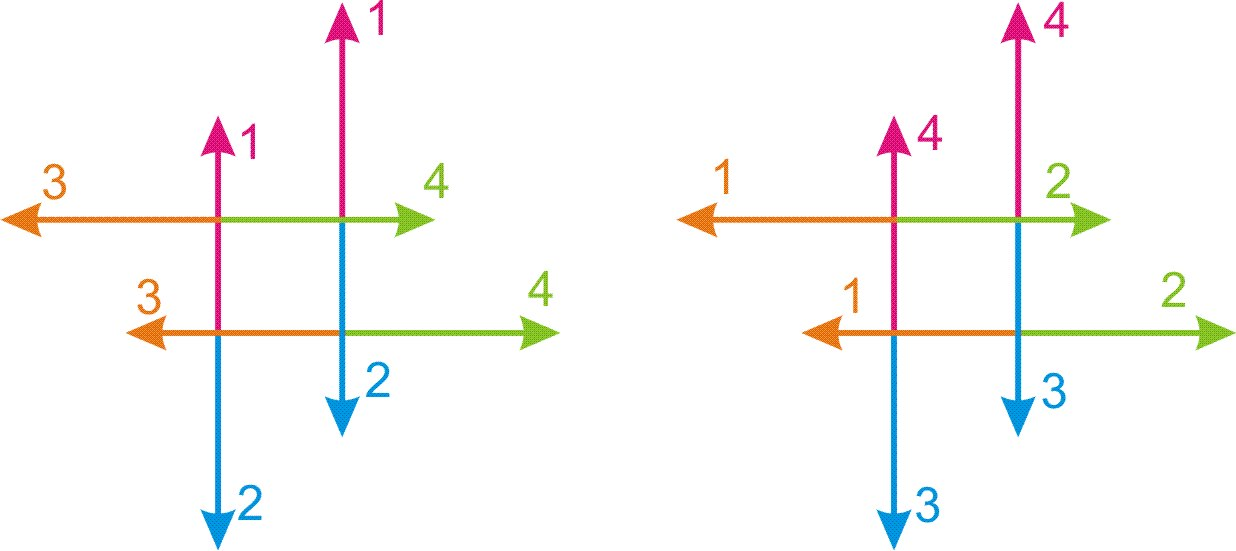Fig. 5.1.

It is important to emphasize: it is not geometric logic that dictates the presence of two sides for a plane, two opposite directions for a line, and the presence of right and left sides when moving in three-dimensional physical space, but the logic of Limitlessness is projected into our customary space of existence by this geometric logic.

Conclusion (c5.7):

The definite dimension is restricted by additional conditions - the presence of origin of reference and the direction of movement of the process of compensating for broken symmetry.

These restricting conditions deprive the definite dimension of the property of complete internal symmetry relative to its parts and its size is reduced relative to the dimension that is not subject to these restrictions.

The movement of the process of compensation for broken symmetry along the definite dimension determines a straight line in the space of the potential part of the localization. This corresponds to the geometric definition of a straight line as the shortest path between two points.

Conclusion (c5.8):

The projection of the definite dimension into the space it forms is a straight line.

In this case, it is worth considering that in the internal space of localization there cannot be points, as objects with zero or infinitesimal size. A geometric point is a mathematical abstraction based on the geometric properties of macro space. Using the abstract concept of a geometric point or an infinitesimal object in physical theories leads to fundamental errors.

The second dimension of the two-dimensional structure of the potential part is not subject to the restrictions imposed on the definite dimension.

Definition (d5.3):

The indefinite dimension is a dimension with respect to which the condition of complete internal symmetry in localization is satisfied.

Conclusion (c5.9):

The indefinite dimension is closed on itself and has no localized parts.

From the condition of closure and complete internal symmetry of the indefinite dimension, together with the Conclusion (c5.8), the following conclusion follows:

Conclusion (c5.10):

The ratio of the size of the indefinite dimension to the size of the definite dimension determines the number π, known in geometry as the ratio of the circumference of a circle to its diameter.

Let us denote the definite dimension of the potential part of localization by the symbol Tu, and the indefinite dimension by the symbol Td0.

From the condition of indeterminacy of the indefinite dimension Td0, as well as the fact that the act of local singling out the material part is projected onto the definite dimension Tu, the following conclusion follows:

Conclusion (c5.11):

The material part of the localization is not localized along the indefinite dimension Td0 of the potential part and is indefinite relative to it.

After passing through all n parts of a definite dimension Tu , the material part ends up at its opposite end. This position similarly violates the symmetry of the internal space of localization, as in the first act. Thus, the cycle of compensation for broken symmetry must be repeated again along the same definite dimension Tu in the opposite direction, that is, along its second main part.

Thus, in the proper relativity of the process, it continues in the same direction, but in the relativity of the fundamental frame of reference and the definite dimension Tu, the process continues in the opposite direction.

Considering that the process proceeds along the two-dimensional structure TuTd0 of the potential part of localization, the two main parts of a definite dimension Tu represent two opposite sides of this two-dimensional structure.

Thus, we are dealing with the refraction of a two-dimensional structure TuTd0 at the end of the definite dimension Tu, and the material part, without repeating the path traveled, passes a second time through all n parts of the definite dimension Tu through the other side of the two-dimensional structure TuTd0.

This process of definite dimension Tu refraction should be identically reflected in the indefinite dimension Td0, as well as in the secondary parts of each of the dimensions. Thus, we are dealing with the refraction of the two-dimensional structure TuTd0 of the potential part of the localization, corresponding to the mathematical projection through the point.

Let's give the following definitions and write down the conclusion:

Definition (d5.4):

The half-cycle of the localization is a sequence of local singling out the material part of localization in n parts of the definite dimension Tu of its potential part.

Definition (d5.5):

The cycle of the localization is a sequence of two of its half -cycles passing along two opposite sides of the two-dimensional structure TuTd0 of its potential part.

Conclusion (c5.12):

The two-dimensional structure TuTd0 of the potential part of localization is refracted at the beginning and end of the definite dimension Tu after the completion of the half-cycle, with a symmetrical revolution of all four parts of each of the two dimensions Tu and Td0, corresponding to the projection through the point.

The restricting condition of having the origin of reference along a certain dimension Tu does not apply to the entire two-dimensional structure TuTd0 of the potential part of the localization.

Conclusion (c5.13):

With respect to the fundamental reference frame, the space of the potential part does not have a singled out center and is symmetrical relative to any of its parts, and the position of its definite dimension Tu is indefinite relative to it.

From this condition, together with the condition of complete internal symmetry of the potential part relative to its parts, as well as its indefiniteness relative to the four-dimensional localization space, the following conclusion follows:

Conclusion (c5.14):

The internal space of localization is a four-dimensional ball, symmetrical with respect to any part of it, the diameter of which is equal to the size of the definite dimension Tu of the potential part of the localization, and the maximum circumference in the section is equal to the size of the indefinite dimension Td0.

Taking into account Conclusions (c5.9) and (в5.10):

Conclusion (c5.15):

The indefinite dimension Td0 of the potential part of the localization is a circle of indefinite radius, the position of which in the localization is determined by the only condition of perpendicularity to the definite dimension Tu, and the size is limited by a maximum size π times larger than the size of the definite dimension Tu.

The displacement of the material part is carried out throughout the two-dimensional structure TuTd0 of the potential part. From the condition that static symmetry breaking is projected only relative to the definite dimension Tu, the following conclusion follows:

Conclusion (c5.16):

The movement of the process of compensation for broken symmetry along the indefinite dimension Td0 occurs simultaneously in two opposite directions along each side of the two-dimensional structure TuTd0.

6. Time

Content

Each act of local singling out the material part of localization represents its separate indivisible state in the sequence of changes of such states according to the cycle of compensation for broken symmetry.

Thus, it is the sequence of states of the material part is what we perceive as the passage of time. The rapid change of states in comparison with the possibilities of registering this process with the human senses creates the illusion of a continuous flow of time. This concept is firmly rooted in physical science, despite the obvious problems of its use in quantum mechanics.

Time is discrete and consists of separate states. The sequence of these states determines the sequence of causes and effects.

Definition (d6.1):

A quantum of state is each act of singling out the material part of localization in the sequence of the process of compensating for broken symmetry.

Conclusion (c6.1):

Time is a sequence of quanta of state.

Thus, the concept of “present”, or “moment of time”, represents a specific quantum of state, and not a conventional boundary between the past and the future.

For each quantum of state separately, the concept of the flow of time is inapplicable, and internal processes are indefinite.

Conclusion (c6.2):

The sequence of processes within a quantum of state is indefinite.

Definition (d6.2):

One-moment processes are processes occurring within a quantum of state.

Based on the fact that all the dimensional characteristics of the internal processes of localization are determined by the movement of the material part along the definite dimension Tu, we will call it the mainstay dimension.

Definition (d6.3):

The mainstay dimension is the definite dimension Tu of the two-dimensional structure TuTd0 of the potential part of localization, along which the sequence of quanta of state of the material part or time is realized.

Conclusion (c6.3):

The process of repeating acts of local singling out the material part according to the mainstay dimension Tu sets the primary measure of extension, which is identically projected into all parts and processes within the localization, determining their commensurability.

Let us define a projection of a quantum of state onto the mainstay dimension Tu as a quantum of extension.

Definition (d6.4):

The quantum of extension is the projection of the quantum of state on the mainstay dimension Tu.

From this definition the following conclusion follows:

Conclusion (c6.4):

The size of the material part of localization along the mainstay dimension Tu is equal to the quantum of extension.

Let us denote the size of the quantum of extension in units of length as dr, and in units of time as dt.

The size of the quantum of extension dr has no comparative characteristics in Limitlessness.

Conclusion (c6.5):

The size of the quantum of extension dr is the basic measure against which all other linear and temporary sizes of parts and processes in localization are projected.

The number of quanta of state in the sequence of time is equal to the number of quanta of extension dr along the mainstay dimension Tu, which in turn is equal to the number of parts singled out in it. Thus, in accordance with definition (d3.2), the number of quanta of state in the half-cycle of localization is equal to its defining integer n.

Rn = ndr (6.1), where Rn - full size of mainstay dimension Tu in units of length.

Tn = ndt (6.2), where Tn - size of half-cycle of localization along mainstay dimension Tu in time units, or time of half-cycle of localization.

Each moment of time is determined by the number of passed quanta of state, which we denote by the symbol nt. Accordingly, we can write:

T = ntdt (6.3), where T - time corresponding to the number nt of quanta of state traversed by the cycle.

Rt = ntdr = Rnnt/n (6.4), where Rt – the path traveled by the material part along the mainstay dimension Tu.

The speed of displacement of the material part in quanta of state along the mainstay dimension Tu is equal to dr/dt .

None of the parts of the material part can outstrip its movement in the space of localization. This gives us reason to denote this maximum speed in the same way as the speed of light is traditionally denoted in physics - the letter c.

In the following chapters, we will prove that light travels in vacuum at precisely this speed.

c = dr/dt (6.5)

Conclusion (c6.6):

The speed c = dr/dt displacement of the material part in quanta of state along the mainstay dimension Tu is the maximum possible speed of movement in the localization.

In accordance with the conclusion Conclusion (c3.1), in the localization of the next order, the whole cycle of the previous localization becomes the first quantum of state.

In the relativity of the external frame of reference, all parts of the localization are indefinite and symmetrical relative to each other and the entire localization. From this condition, the conclusions follow:

Conclusion (c6.7):

In the relativity of the external frame of reference, the process of compensation for broken symmetry occurs simultaneously in two opposite directions.

Conclusion (c6.8):

In the relativity of localization of the next order, the size of the full cycle of localization of the previous order is equal to the size of its half-cycle.

Thus, in accordance with formulas (6.1) and (6.2), the size of quantum of state of the next order localization in units of length is ndr, and in units of time is ndt. Accordingly, the speed of movement through the cycle will be equal to:

ndr/ndt = dr/dt = c =const (6.6)

Conclusion (c6.9):

The speed of displacement of the material part along the localization cycle does not depend of its order and is the universal constant.

7. Local frame of reference and physical space

Content

In accordance with the Conclusion (c3.2), the material part of the localization with the defining integer n consists of n2 of its identical parts.

With respect to the fundamental reference system (definition (d2.3)), the local singling out the material part does not affect its parts and their symmetry relative to each other.

Conclusion (c7.1):

In the fundamental frame of reference, all parts of the material part are not localized relative to it.

The process of random fluctuation, as localization in Limitlessness, must be identically reflected in all its parts. Accordingly, the process of random singling out and localization of parts must also take place inside the material part, creating new frames of reference. For the appearance of an internal reference system inside the material part of the localization, it is sufficient to localize one part, which singling out it from others.

Definition (d7.1):

A local frame of reference is a frame of reference associated with a part of the material part of localization that localized along both dimensions of its two-dimensional structure.

The choice of local reference frame represents a restricting condition. In accordance with theorem (t4), we come to the conclusion:

Conclusion (c7.2):

Processes occurring in one local frame of reference and representing a consequence of the restrictions that form it are not projected into another local frame of reference.

When any of the n2 parts of a material part is localized, all other parts are localized according to the principle of identity. This localization of all parts of the material part, according to theorem (t4), occurs in each local frame of reference according to its restricting conditions.

Conclusion (c7.3):

The localization conditions for all n2 parts of the material part of the localization are relative and depend on the chosen local frame of reference.

An important conclusion follows from this conclusion:

Conclusion (c7.4):

Processes occurring in different local reference systems represent alternative realities, each of which is formed exclusively under the restricting condition of its local reference system.

According to the principle of identity with the potential part, the space of the material part of localization must be formed by definite and indefinite dimensions.

Let us denote a definite dimension of the material part of localization by the symbol Ru, and an indefinite dimension by Rd.

From the condition of identity of the main parts of localization in accordance with the Conclusion (c5.12), a similar conclusion follows in relation to the material part:

Conclusion (c7.5):

The internal space of the material part of the localization is refracted at the beginning and at the end of its mainstay dimension Ru with a symmetrical revolution relative to its two-dimensional structure RuRd corresponding to the projection through the point.

In accordance with definition (d7.1), the localization of all n2 parts of the material part in the local frame of reference occurs along each of its two dimensions Ru and Rd.

This process of localization of parts along the indefinite dimension Rd must be identically projected onto the indefinite dimension Td0.

From this condition, in conjunction with the condition of indefinites of the material part along the indefinite dimension Td0 of the potential part (conclusion (c5.11)), it necessarily follows that the localization of parts of the material part along the dimension Td0 occurs identically to their localization along the indefinite dimension Rd of the material part relative to its size.

Conclusion (c7.6):

The indefinite dimension Td0 of the potential part of the localization is projected into the material part identically to its indefinite dimension Rd and relative to its size.

Let us denote this projection of the indefinite dimension Td0 of the potential part of the localization by the symbol Td.

From the condition of projection of the main parts of localization into each other (Conclusions (c2.4) and conclusion (c4.3)), the conclusion follows:

Conclusion (c7.7):

The indefinite dimension Td is simultaneously a projection of the indefinite dimension Td0 of the potential part of the localization into the local frame of reference and a projection of the material part of the localization onto the dimension Td0 in relation of the local reference frame.

In accordance with the Conclusion (c6.6) in the relativity of the local frame of reference, the entire material part of the localization moves along the mainstay dimension Tu with the same speed c.

Conclusion (c7.8):

All parts of the material part are localized along the mainstay dimension Tu with one size equal to the quantum of extension dr.

Conclusion (c7.9):

The mainstay dimension Tu is not projected into the local frame of reference, and no part of the material part in the local frame of reference can be shifted relative to another part along this dimension, or have quantitative or qualitative relationships along it, except for the principle of complete identity.

Thus, in a local frame of reference, time represents dynamic interaction conditions and is not reflected on static conditions.

Conclusion (c7.10):

Time, as a sequence of quanta of state of the material part of the localization, in the relativity of the local frame of reference has no spatial properties.

Taking into account Conclusions (c4.6) and (в7.9):

Conclusion (c7.11):

The interaction space of the parts of the material part in the local frame of reference is three-dimensional and is formed by two dimensions Ru and Rd of the material part and the dimension Td as the projection of the indefinite dimension Td0 of the potential part.

This is the space that we are accustomed to perceive as physical.

Conclusion (c7.12):

Physical space is a three-dimensional projection of a four-dimensional localization space into a local reference frame.

Taking into account the Conclusion (c7.6):

Conclusion (c7.13):

The size of the dimension Td, as the projection of the indefinite dimension Td0 of the potential part into physical space, is equal to the size of the indefinite dimension Rd of the material part.

In the future, for convenience, we will call the definite dimension Ru of the material part - the definite dimension of the physical space, and the indefinite Rdthe indefinite dimension of the physical space..

According to the principle of symmetry (conclusion (c1.2)), the entire material part of the localization should be projected symmetrically with respect to each of its singled out parts.

Conclusion (c7.14):

Each local frame of reference in its own relativity is in the center of physical space.

From this conclusion it is necessary to follow the following:

Conclusion (c7.15):

Physical space is relative and depends on the choice of local frame of reference.

Thus, not only physical processes in the local reference frame are relative in accordance with Conclusions (c7.3) and (в7.4), but physical space itself is also relative.

From the condition of indefinites of the material part of the localization along the indefinite dimension Td0 of its potential part (conclusion (c5.11)), it follows that the position of the local frame of reference, and, consequently, the physical space relative to it, is indefinite.

Thus, the local reference frame may have motion along the indefinite dimension Td0 of the potential part of the localization, which is not reflected by motion in physical space.

Conclusion (c7.16):

The movement of the local frame of reference along the indefinite dimension Td0 of the potential part of the localization is not reflected by the movement in physical space, and the entire physical space moves with it.

From the condition of symmetry of the material part relative to the local frame of reference, it follows that the origin of reference of the definite dimension of the material part Ru, in projection into physical space, coincides with its center. From this, taking into account the Conclusion (c5.12), the conclusion follows:

Conclusion (c7.17):

The refraction point of the two-dimensional structure RuRd of the material part is projected into the center of the local frame of reference.

Thus, both sides of the two-dimensional structure RuRd of the material part in an unfolded projection should be projected into physical space, Fig. 7.1, a).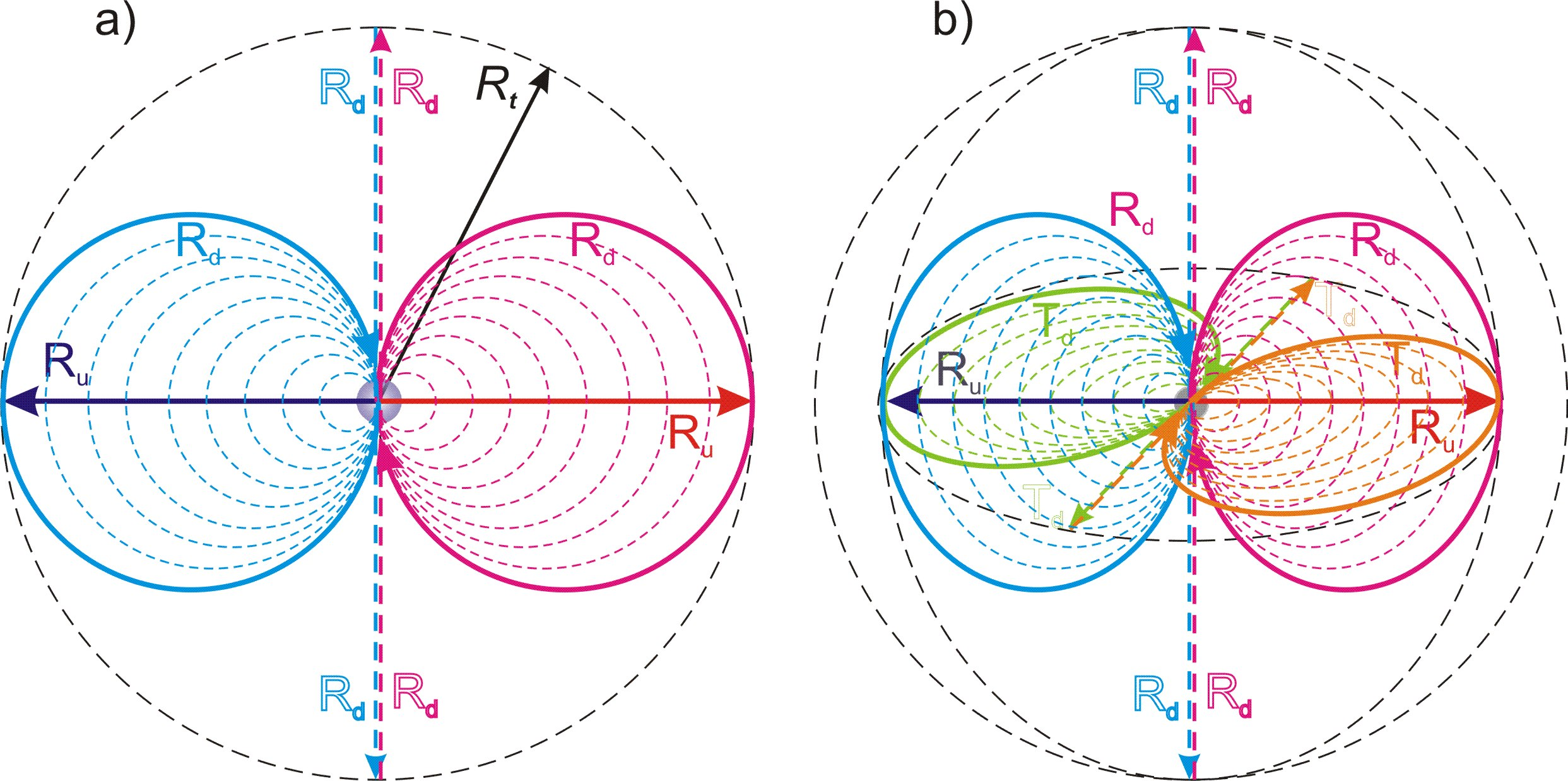Fig. 7.1.

Conclusion (c7.18):

The material part of the localization is projected into physical space relative to the center of the local reference system by an unfolded projection of two opposite sides of the two-dimensional structure RuRd.

Conclusion (c7.19):

The definite dimension Ru of the material part of the localization is projected into physical space with two opposite directions “from inside to outside” relative to the center of the local frame of reference.

Conclusion (c7.20):

The indefinite dimension Rd of the material part of the localization is projected into physical space by two circles of indefinite size, passing through the center of the local frame of reference and turned oppositely.

In accordance with Conclusion (c7.13), the indefinite dimension Td is projected into physical space in a similar way, Fig. 7.1, b).

Conclusion (c7.21):

The indefinite dimension Td is projected into physical space identically to the indefinite dimension Rd and perpendicular to it.

As we can see, only in relative proximity to the center of the local reference frame, physical space is the way we used to perceive it, and how modern physics describes it. In this case, the direction of the indefinite dimensions Rd and Td are straight lines perpendicular to each other and to the definite dimension Ru, fig. 7.1, b).

Conclusion (c7.22):

As we move away from the center of the frame of reference, physical space becomes more and more uncertain.

From the Conclusion (c7.17) and the condition of indefinites of the directions of all three dimensions of physical space, the conclusion follows:

Conclusion (c7.23):

Physical space is a three-dimensional ball whose radius is its definite dimension Ru.

Identical to the movement of the material part along the mainstay dimension Tu, the radius of physical space must increase in proportion to the number nt of traversed quanta of state, and in each quantum of state be equal to the path Rt traversed by the material part.

Conclusion (c7.24):

The radius of physical space is equal to the path Rt traversed by the material part of the main localization along the mainstay dimension Tu.

Thus, the radius of the physical space is determined by formula (6.4):

Rt = ntdr

Conclusion (c7.25):

The radius of the physical space Rt increases along the sequence of quanta of state with the maximum possible speed c.

In accordance with Conclusions (c5.10) and (в7.13) we can write:

Rtd = πRt = πntdr (7.1), where Rtd – size of indefinite dimensions Rd and Td of the physical space.

From the Conclusion (c7.23) and formula (6.4) it follows that in the first quantum of state in the sequence of time, the material part was compressed to a size n times smaller than its potential size in the last act. Thus, the process of expansion with the maximum possible speed c is nothing more than the “Big Bang”, a conclusion about which is prompted by practical measurements and observations of our Universe.

Conclusion (c7.26):

The “Big Bang”, as the beginning of the existence of our Universe, represents the beginning of the sequence of its quanta of state in the cycle of compensation for broken symmetry in its localization.

Thus, the “Big Bang” is not only the first moments of the existence of our Universe, but its state throughout the entire half-cycle. As size of it increases with the maximum possible speed c, the relative size changes in a small local region such as our immediate cosmos become virtually undetectable compared to the historical period of scientific observation.

8. Elementary localizations as fundamental particles of matter

Content

The process of localization of a part within the material part is identical to the process of localization of the material part itself. It follows from this that each of the n2 parts of the material part forms its own localization, identical to the main one. For convenience, we give the following definitions:

Definition (d8.1):

The main localization is each localization in the sequence of localizations generated by a random fluctuation.

Definition (d8.2):

Elementary localization is the localization of each of the n2 identical parts of the material part of the main localization with the defining integer n.

Conclusion (c8.1):

Elementary localizations are identical to the main localization.

It follows from this that elementary localization must consist of material and potential parts, and its size and internal space are determined by the two-dimensional structure of the potential part with definite and indefinite dimensions.

Let us denote a definite dimension of the two-dimensional structure of the potential part of an elementary localization by the symbol ru, and an indefinite dimension by the symbol rd.

From the principle of dividing into n parts (conclusion (c4.4)) each of the two dimensions Ru and Rd of the material part of the main localization, the conclusion follows:

Conclusion (c8.2):

The size of the definite dimension ru of the potential part of elementary localization is n times smaller than the size Rt of the definite dimension Ru of physical space.

Taking into account formula (6.4):

rt = Rt/n = drnt/n (8.1) , where rt — size of the definite dimension ru elementary localization in a quantum of state nt.

Conclusion (c8.3):

The size rt of a definite dimension ru of elementary localization is dynamic and depends on the number of quanta of state nt passed through.

The completion of the half-cycle of elementary localization occurs together with the completion of the half-cycle of the main localization in the last quantum of state of the main localization at nt = n. In accordance with formula (8.1) at nt = n rt = dr.

Conclusion (c8.4):

The size of the half-cycle of an elementary localization along its definite dimension ru is equal to the quantum of extension dr.

From the principle of identity of parts and the whole (theorem (t2)) the conclusion follows:

Conclusion (c8.5):

The defining integer n of the main localization is equal to the defining integer of each of its elementary localizations.

The conditions of the internal space of elementary localization are completely identical to the conditions of the internal space of the main localization in the fundamental frame of reference. Thus, similarly to conclusions (c5.13) and (c5.12), we can write:

Conclusion (c8.6):

The positions of the definite ru and indefinite rd dimensions of the elementary localization are indefinite with respect to the two-dimensional structure rurd of its potential part.

Conclusion (c8.7):

The space of the potential part of the elementary localization is refracted after each half-cycle, and the material part is turned over relative to the main sides and directions of each of its two dimensions ru and rd.

The projections of elementary localizations into the physical space are the fundamental particles of matter.

Definition (d8.3):

Fundamental particles are projections of elementary localizations into the physical space.

The size of a fundamental particle in physical space is determined by the dimensions of the elementary localization, and the size along the mainstay dimension Tu, as well as the size of the entire material part along it, is equal to the quantum of extension dr (conclusion (c7.8)).

Taking into account the three-dimensionality of physical space, Conclusion (c8.6) and the principle of symmetry:

Conclusion (c8.8):

A fundamental particle is a three-dimensional ball in physical space with the indefinite internal space, the diameter of which is equal to the size rt of its definite dimension ru.

The indefinite dimension rd of the potential part of the elementary localization is projected into physical space perpendicular to the definite ru, and the only determining condition for it is the maximum size of its extension, which, in accordance with Conclusion (c5.10), is equal to πrt. From this follows a conclusion similar to the conclusion (c5.15) regarding the space of the main localization:

Conclusion (c8.9):

The indefinite dimension rd of the potential part of the elementary localization is projected into physical space by a circle of indefinite radius, the position of which is determined by the only condition of perpendicularity to the definite dimension ru, and the size is limited by the maximum size πrt.

Unlike the main localization in the fundamental frame of reference, in which it is completely closed and has no external space, the elementary localization in the local frame of reference is in the conditions of physical space, as external, in relation to it. From this follows the conclusion:

Conclusion (c8.10):

The closing sphere of the internal space of a fundamental particle has an internal and external side, each of which is formed by one of the two main sides of the indefinite dimension rd of its potential part.

The material part of the elementary localization must be projected into physical space by a material object.

Definition (d8.4):

The physical body of a fundamental particle is the projection of the material part of the elementary localization into physical space.

From the condition of indefiniteness of the position of the material part in the internal space of elementary localization, in accordance with Conclusion (c8.8), the conclusion follows:

Conclusion (c8.11):

A fundamental particle represents in physical space a three-dimensional sphere of location of its physical body, the position of which relative to it is indefinite.

In quantum mechanics, the state of indefinites of parameters of quantum objects is defined as a superposition of alternative variants of reality, but this approach is not entirely correct and leads to erroneous worldview concepts, such as, for example, the “Everett many-worlds model.” An alternative reality is not chosen from a certain set of variants, but is formed by the act of choice, and begins to exist only from this moment of choice.

The conditions for the projection of the physical body of a fundamental particle into physical space are identical to the conditions for the projection of the entire particle. In this case, the conditions of physical space are imposed on the conditions of the internal space of the fundamental particle.

Conclusion (c8.12):

The physical body of a fundamental particle is a three-dimensional sphere in physical space with an indefinite center, being simultaneously under the conditions of the projection of the internal space of elementary localization and in the conditions of physical space.

Taking into account the indefinites of the position of the physical body inside the fundamental particle (conclusion (c8.11)), we call the value rt the size of presence of the fundamental particle.

Definition (d8.5):

The size of presence rt of a fundamental particle is the diameter of a spherical region of physical space, which represents the region of indefiniteness in the position of its physical body.

According to the principle of identity with the main localization, the ratio of the size of the physical body of the fundamental particle rp to the size of presence rt must be equal to a similar ratio of the size Rt of the material part of the main localization along its definite dimension Ru to the size Rn of the mainstay dimension Tu.

rp/rt = Rt/Rn = nt/n (8.2), where rp — size of a physical body in quantum of state nt.

Taking into account formula (8.1) we can write:

rp = rtnt/n = nt2dr/n2 (8.3)

The physical body of a fundamental particle in each of the possible alternative positions within its size of presence is a material manifestation of the fundamental particle. Thus, we can call the size rp the physical size.

Definition (d8.6):

The physical size of a fundamental particle is the diameter of its physical body rp in the physical space.

9. Movement in physical space

Content

In accordance with Conclusion (c7.4), each local frame of reference forms its own alternative physical reality. Therefore, in the future, when considering physical processes, we assume that they occur in the relativity of one local frame of reference, and represent one alternative reality.

Definition (d9.1):

A physical frame of reference is a frame of reference associated with any physical object in the conditions of physical reality implemented in the selected local frame of reference.

Thus, the concept of "physical frame of reference" corresponds to the concept of "frame of reference" adopted in physics.

Violation of the symmetry of one of the fundamental particles relative to another causes a cycle of compensation for the broken symmetry, which makes them move towards each other. We will examine this issue in the chapter “Gravity,” but now what is important for us is that the particles begin to shift relative to each other.

This shift occurs during the transition from one quantum of state to another.

As an identical part of the material part of localization, in accordance with Conclusions (c7.9) и (в6.6), each fundamental particle in its own relativity moves along the mainstay dimension Tu with the constant speed c = dr/dt.

Conclusion (c9.1):

Any physical frame of reference associated with any of the fundamental particles, or their group, moves along the mainstay dimension with the maximum possible speed c.

The sequence of quanta of state of the material part is displaced along the two-dimensional structure TuTd0 of the potential part of the main localization. It follows from this that any movement of fundamental particles, as parts of a material part, is possible only along this two-dimensional structure TuTd0. But if we take into account the fact that, on the one hand, the movement along the mainstay dimension Tu always occurs at the same speed c, and on the other hand, only one of the dimensions of this two-dimensional structure is reflected in the physical space - the indefinite Td0, the conclusion follows:

Conclusion (c9.2):

Any movement of material objects in the physical space is carried out along the projection Td of indefinite dimension Td0 of the two-dimensional structure of the potential part.

In accordance with this conclusion, for the convenience of further reasoning, we will give the corresponding name to this dimension:

Definition (d9.2):

The mobile dimension is the indefinite dimension Td, which is a projection of the indefinite dimension Td0 of the two-dimensional structure of the potential part of the main localization into physical space.

The localization conditions that determine the speed c of displacement of the material part across the two-dimensional structure TuTd0 of the potential part do not in any way restrict the range of possible directions of this process. As a result of imposing the conditions of a spot cycle of compensation for the broken symmetry on the main cycle, the direction of the mainstay dimension Tu in relation to one or another particle changes.

Conclusion (c9.3):

The direction of the vector of the mainstay dimension Tu in relation of two fundamental particles moving relative to each other is different.

As a consequence of this difference in the direction of the mainstay dimension vector Tu in the reference frame associated with one particle, its projection onto the mobile dimension Td in the relativity of another particle arises, fig. 9.1.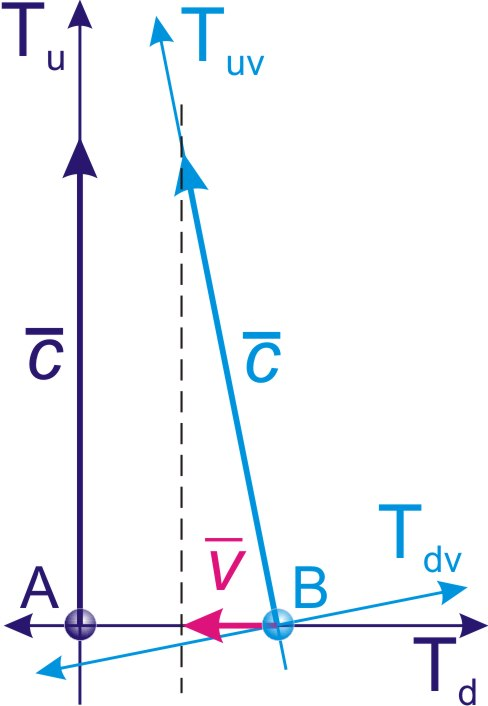Fig. 9.1.

On fig. 9.1 shows the reference frame TuvTdv moving with velocity v relative to the reference frame TuTd.

The motion vector along the mainstay dimension Tu of the reference frame TuvTdv is projected onto the mobile dimension Td of the reference frame TuTd by the motion vector with the velocity v relative to it.

Conclusion (c9.4):

The movement of material bodies in the physical space is a projection of their movement along the eigenvector of the mainstay dimension Tu onto the mobile dimension Td of the reference frame.

Thus, the condition of indefiniteness of the mobile dimension Td in the relativity of each moving physical object is superimposed by the direction condition determined by the vector of its movement.

Conclusion (c9.5):

The direction of the mobile dimension Td of the physical reference system is projected relative to each moving material object by its motion vector.

In accordance with the Conclusions (c7.20) and (в7.21), the mobile dimension Td is projected into physical space in an unfolded projection relative to its two main sides by two circles passing through its center.

From the condition of indefiniteness of the mobile dimension Td it follows that the movement of fundamental particles, as identical parts of the material part, in accordance with Conclusion (c5.16), must pass simultaneously through all of four its parts.

Conclusion (c9.6):

The movement of a fundamental particle in physical space occurs simultaneously along two main parts of the mobile dimension Td in its unfolded projection relative to the sides of the two-dimensional structure TuTd of the potential part of the main localization.

Considering the position of the fundamental particle in its own relativity at the center of physical space, the direction of the mobile dimension Td must coincide with the radius of physical space.

The fact that the definite dimension Ru of physical space determines the radius of physical space in a static situation does not mean that it coincides with it in all cases. In the case of a moving particle, the definite dimension Ru is projected perpendicular to the motion vector.

However, in accordance with the Conclusions (c7.20) - (в7.22), the coincidence of the direction of the mobile dimension Td with the radius of physical space occurs only in the immediate vicinity of the center of the frame of reference, fig. 9.2.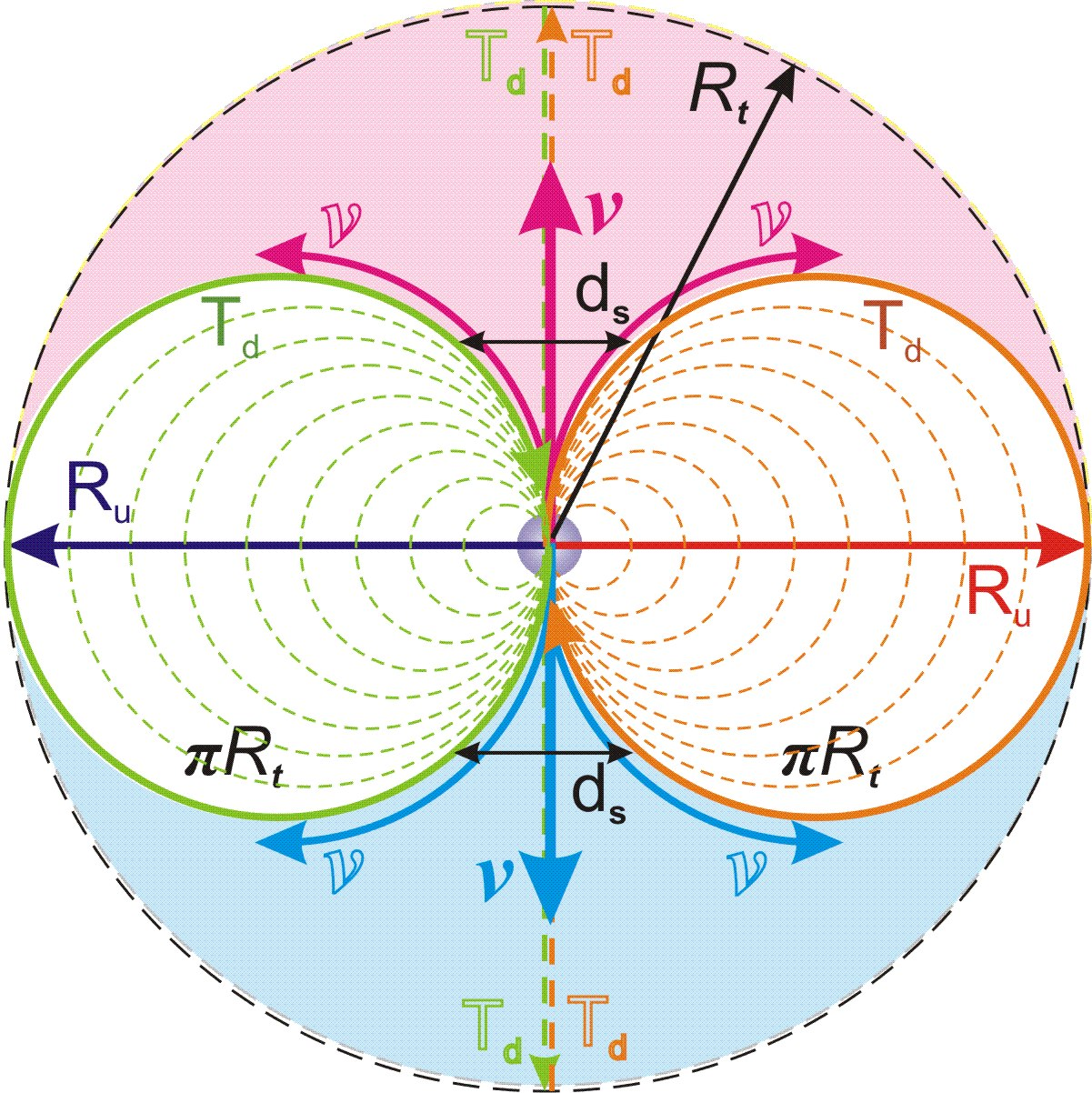Fig. 9.2.

The projections of the two sides of the mobile dimension Td into physical space begin to diverge as they move away from the center of the local frame of reference, and the position of the fundamental particle becomes indefinite.

The further from the center of the reference system, the larger the size ds of the region of this indefiniteness, fig. 9.2. When approaching the boundary of physical space, ds tends to the maximum size Rt.

When the physical frame of reference moves relative to the local frame of reference, in its own relativity, the physical frame of reference at each moment of time will be in the center of physical space, and the size ds of the region of indefiniteness relative to it will be close to zero. But relative to the local frame of reference, this moving frame of reference will be in a region of indefiniteness, the size of which ds will increase as it moves away from the center of the local frame of reference.

Thus, physical space in the relativity of motion processes does not have straight lines. The following conclusion must be drawn from this:

Conclusion (c9.7):

The concept of motion in a straight line for physical bodies is a mathematical abstraction that does not exist in real physical space.

Conclusion (c9.8):

Our planet Earth is a local frame of reference located at the center of the Universe. At the same time, outer space, together with space objects, becomes more and more indefinite with respect to spatial and, consequently, physical properties as they move away from the Earth.

If you move (for example, on a spaceship) over long cosmic distances, the geometry of the Universe will change.

Since we obtain all information about deep space from the movement of particles, this effect of spatial indefiniteness, which increases with increasing distance from Earth, introduces distortions into modern physics calculations that do not take it into account. The erroneous principle of extrapolating near-Earth geometry to long cosmic distances forces physicists to invent non-existent “dark energy” and “dark matter”.

10. Inertial and uniformly accelerated motions

Content

The material part in each quantum of state gains a speed of displacement along the mainstay dimension Tu only relative to itself. Considering the absence of other dimensional relationships in Limitlessness except with itself, this accumulated speed in a quantum of state does not correlate with anything except the quantum of state itself, and in each subsequent quantum of state the process of gaining speed is repeated anew.

Conclusion (c10.1):

The process of the material part gaining speed in a quantum of state is identically repeated in the next quantum of state.

Thus, in each quantum of state, the force of the cycle gives acceleration to the material part:

a0 = c/dt = dr/dt2 (10.1)

In accordance with Conclusions (c6.1) and (в6.2), the concept of “time” does not exist in the quantum of state; accordingly, the concept of “average speed” cannot exist.

Conclusion (c10.2):

The material part of the localization moves along the mainstay dimension Tu with the same speed c = dr/dt and the same acceleration a0 = dr/dt2 in each quantum of state.

Each fundamental particle in its own relativity moves along the mainstay dimension Tu with the maximum possible speed c (conclusion (c9.1)). The direction of the vector of this movement relative to each particle is determined by the process of compensating for broken symmetry.

Conclusion (c10.3):

In the absence of influences that change the state of symmetry, the direction of the motion vector of the fundamental particle along the mainstay dimension Tu is unchanged.

Accordingly, the projection of this movement into the local frame of reference is also unchanged.

This means that if the symmetry state remains unchanged, the speed of the particle relative to the local frame of reference is preserved. Thus, we have found the reason that defines the inertia of movement in physical space.

Let us give the following definitions:

Definition (d10.1):

Inertial movement or inertia is movement under conditions of maintaining its own relative direction of the vector of the mainstay dimension.

Definition (d10.2):

An inertial frame of reference is a physical frame of reference associated with one or a group of fundamental particles moving by inertia.

Further, for convenience, when mentioning a physical frame of reference (definition (d9.1)) we will assume that we mean an inertial frame of reference.

However, in contrast to classical physics, where rectilinear uniform motion is considered inertial, it follows from the theory that only the motions of cosmic bodies in circular orbits are inertial, and in accordance with Conclusion (c9.7) rectilinear motion does not exist in physical space.

In the case of orbital motion, the angle φ between the directions of the mainstay dimension Tu in the relativity of each of the cosmic bodies remains unchanged relative to any inertial frame of reference, fig. 10.1.Fig. 10.1.

But such a circular inertial motion of cosmic bodies is possible only if the orbital radius is insignificant in comparison with the size of the Universe, at which the vector of the mainstay dimension Tu retains its direction on opposite sides of the orbit.

Due to the expansion of physical space, at large distances the direction of the mainstay dimension Tu relative to distant space objects deviates. Since this deviation is a consequence of the movement of the main cycle, no additional forces arise, and the movement of cosmic bodies remains inertial.

Conclusion (c10.4):

At large distances comparable to the dimensions of physical space, the direction of the mainstay dimension Tu deviates from the similar direction at the center of the local frame of reference.

Thus, at large distances this movement will have a shape resembling a diverging spiral, which is what we observe in the form of galaxies, fig. 10.2.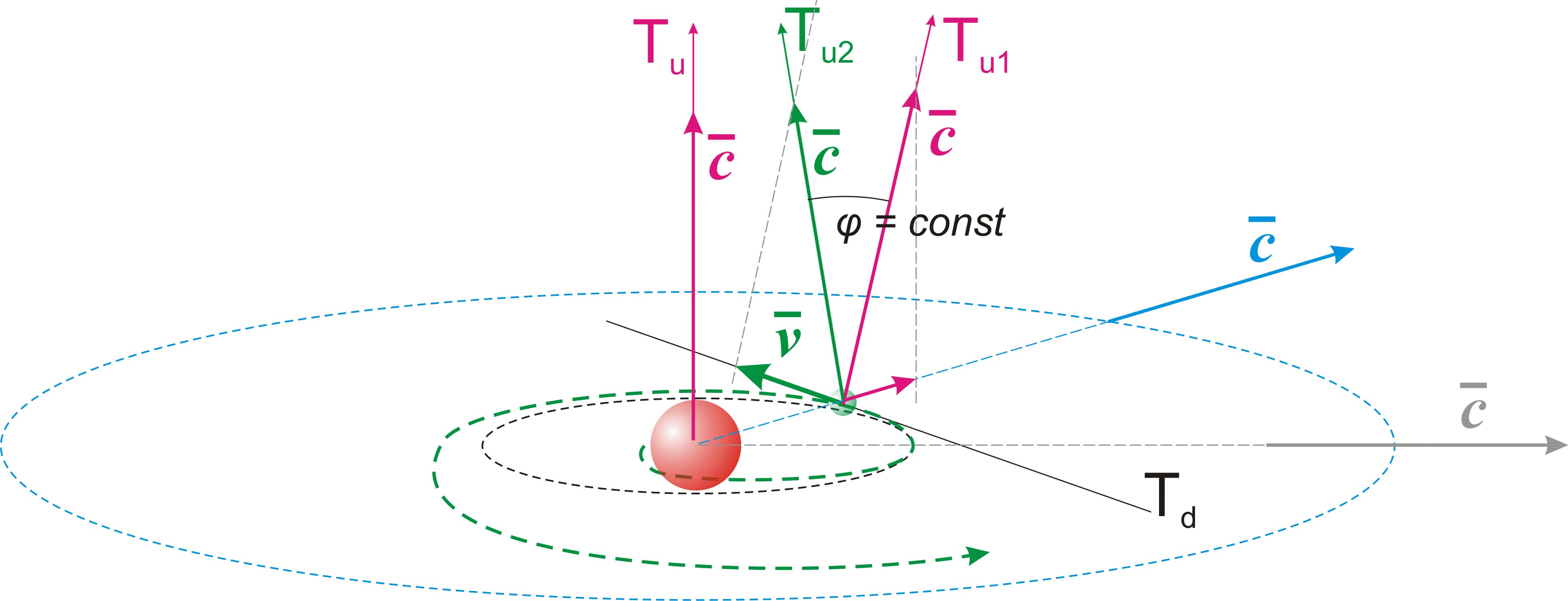Fig. 10.2.

This is additional confirmation of this theory.

Conclusion (c10.5):

Inertial motion at galactic distances is a diverging spiral, the magnitude of the divergence of which at small distances comparable to stellar systems tends to zero, as a result of which the inertial motion of the planets occurs in circular orbits.

To this conclusion we are forced to add another important conclusion:

Conclusion (c10.6):

The principle of inertiality of uniform rectilinear motion when applied to cosmic scales is a systemic error in classical physics.

If any impact on an elementary particle leads to a change in its relative direction of the mainstay dimension Tu, this changed state, according to the principle of inertia (conclusion (c10.3)), remains after the cessation of the impact.

Conclusion (c10.7):

The speed of movement of fundamental particles in physical space, imparted by any influence in the quantum of state, is added to the existing speed and is maintained after the termination of the influence.

Thus, if a certain interaction of particles gives any of them in the quantum of state acceleration a of movement along the mobile dimension Td, its movement becomes uniformly accelerated:

v = anidt (10.2), where ni – the number of quanta of state in which acceleration acted.

nidt = t (10.3), where t – the duration of the acceleration.

Respectively:

v = at (10.4)

11. Time and dimensions in moving reference frames

Content

On fig. 11.1 shows the resting frame of reference TuTd. And the frame of reference TuvTdv moving with speed v relative to the frame TuTd.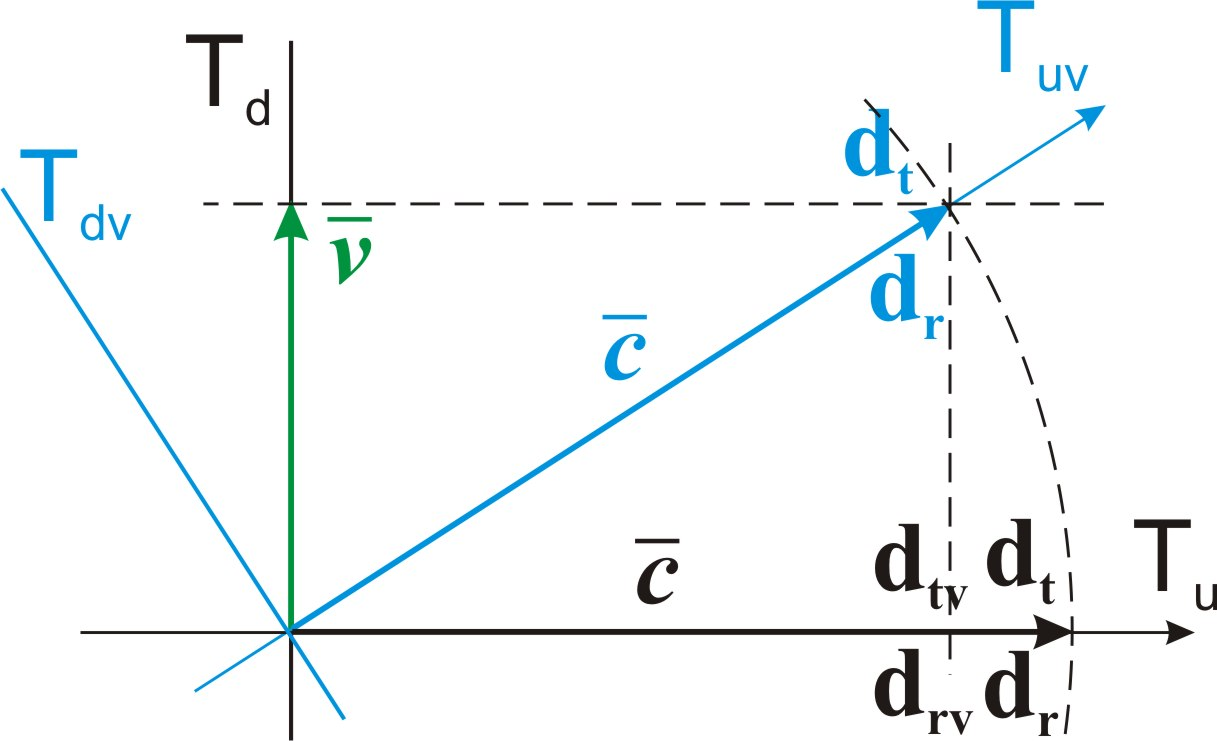Fig. 11.1.

The size of the quantum of extension drv of the moving frame of reference is reduced in projection onto the mainstay dimension Tu of the frame of reference at rest.

From the identity of triangles, we find:

drv/dr = dtv/dt =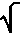(c2-v2)/c =(1-v2/c2) (11.1), where drv – the size of the projection of quantum of extension of the moving frame of reference in units of length, dtv – the size of the projection of quantum of extension of the moving frame of reference in units of time.

drv = dr(1-v2/c2) (11.2)

dtv = dt(1-v2/c2) (11.3)

Conclusion (c11.1):

The size of the quantum of extension of the moving frame of reference is reduced in the projection onto the stationary dimension Tu of the resting frame of reference in proportion to(1-v2/c2).

However, the interpretation of this reduction as time dilation in a moving frame of reference is erroneous.

In accordance with the definition (d6.4) and Conclusion (c6.2), the sequence of processes within the size of the quantum of extension dt along the mainstay dimension Tu is indefinite. It follows from this that the quantum of extension dtv of the moving frame of reference in the projection into the resting frame of reference is indefinite within the boundaries of its quantum of extension dt, fig. 11.2.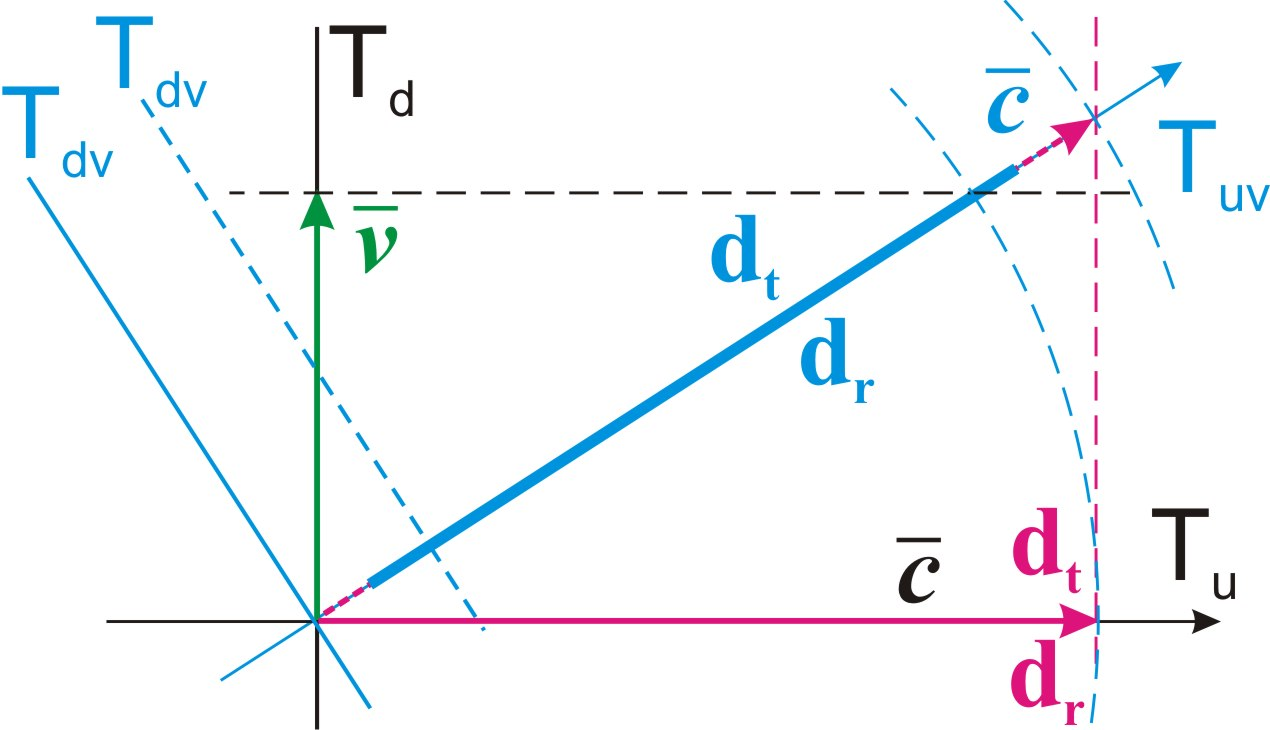Fig. 11.2.

On the other hand, in accordance with Conclusion (c7.9) in the relativity of any local frame of reference, all material objects projected into it, regardless of the relative speeds of their movement, move with it from one quantum of state to another in the sequence of time and cannot get ahead or lag behind this process.

Conclusion (c11.2):

In the relativity of the local frame of reference, the displacement in the quantum of state is the same for all parts of the material part of the localization, regardless of their speeds of movement, and is equal to dr.

Taking into account the indefiniteness of processes within the quantum of extension, we come to the conclusions:

Conclusion (c11.3):

The quantum of extension of a resting frame of reference includes the quanta of extension of all moving frames of reference, in a state of indefinite regarding its size.

Conclusion (c11.4):

Time, as a sequence of quanta of state, flows identically in the relativity of any frame of reference, regardless of the speed of its movement.

In accordance with Conclusion (c11.1) and formula (8.1), the relative reduction in the projection of the quantum of extension of the moving frame of reference into the resting one leads to a relative reduction in the size of the fundamental particle. In accordance with formula (11.2):

rtv/rt =(1-v2/c2) (11.4)

rtv = rt(1-v2/c2) (11.5)

Conclusion (c11.5):

The size of presence rtv of a moving fundamental particle with speed v is reduced relative to the size of presence rt of a particle at rest in proportion to(1-v2/c2), in any inertial frame of reference.

This conclusion coincides with the conclusions of TO only partially. The difference is that in TO the decrease in the size of a moving body occurs exclusively along the vector of its movement. According to this theoretical study, this decrease in the size should occur symmetrically along all three dimensions of physical space.

12. Quantum of state of the physical process and of local frame of reference

Content

Any physical process is an interaction of projections of elementary localizations into the physical space, the movement in which (conclusion (c9.2) and definition (d9.2)) оis carried out along the mobile dimension Td. In this case, the speed of interaction cannot exceed the maximum possible speed c (conclusion (c6.6)).

Conclusion (c12.1):

A change in the state of one fundamental particle can affect the state of another fundamental particle no faster than after a time interval equal to the ratio of the distance between them to the speed c.

This ratio represents the minimum time interval or quantum of extension of the process of interaction of two particles.

Definition (d12.1):

The size of a physical process is the maximum distance between its parts in physical space.

Definition (d12.2):

The quantum of extension of a physical process is the ratio of its size to the maximum possible speed c.

dtr = Rf/c = Rfdt/dr (12.1), where dtr – the size of the quantum of extension of the physical process according to the mainstay dimension Tu in units of time, Rf – spatial size of the physical process.

Within the frames of the process defining by the quantum of extension, the relationship of the parts of this process is indefinite.

Definition (d12.3):

The quantum of state of a physical process is its minimal indivisible state in the sequence of states, in which each of the parts of this process is reflected in every other part of it.

Thus, the quantum of state of the process in the physical space is equal to its size Rf, and in the projection onto the mainstay dimension Tu its size is equal to the size of its quantum of extension dtr.

Within a quantum of state of a process, its parts are in indefiniteness relative to each other.

Conclusion (c12.2):

In the quantum of state of a physical process, the sequence of its parts is indefinite.

Each local frame of reference is formed in relation to a certain set of physical processes with which it is associated.

Definition (d12.4):

The quantum of state of the local frame of reference is the holistic one-moment state of the local frame of reference corresponding to the quantum of state of the physical process in the relativity of which it was formed.

Conclusion (c12.3):

The sequence of events and physical processes in a local frame of reference within its own quantum of state is indefinite.

Another important conclusion follows from the conclusions drawn in this chapter:

Conclusion (c12.4):

Any comparison of processes located in space at a distance R is correct only with respect to a frame of reference, the size of which is greater than R, and the size of the quantum of state along the mainstay dimension Tu in units of time is greater than R/c.

The indefiniteness of events separated by distance R and time less than R/c is called the relativity of simultaneity in Theory of relativity. However, this conclusion about the relativity of simultaneity is completely ignored when asserting that the flow of time in a moving frame of reference slows down relative to a resting one, which leads to a conceptual error.

Let us pay attention to the fact that the size of the quantum of state of the frame of reference associated with planet Earth is equal to the ratio of its diameter to the maximum possible speed c. This ratio is exactly equal to the minimum period of time for a person to perceive successive stages of movement of the objects he observes (24 frames per second). If we take into account the fact that the interval for taking readings by an experimenter from any physical device cannot be less than the size of this quantum of state in units of time, we can come to interesting conclusions, but this topic is beyond the scope of this study.

13. The force and the mass

Content

Following the traditions of mechanics, we will call the effect of the process of compensation of broken symmetry, which imparts acceleration to parts of the material part of the localization, a force and denote it with the symbol f.

Processes of compensation for broken symmetry arise as a result of the local singling out of a part within the whole. Under conditions of indefiniteness of the internal space of localization and of the separated part, the only characteristic of symmetry breaking is the ratio of the size of the internal space of localization to the size of the separated part.

Let us give the following definitions:

Definition (d13.1):

An elementary particle is a locally singled out part of the material part of localization with an indefinite internal space.

Definition (d13.2):

Definiteness along dimension is the ratio of the size of the dimension relative to which an elementary particle has been locally singled out to the size of this particle along it.

Local singling out, both of the entire material part and each of its parts, occurs inside the two-dimensional structure TuTd0 of the potential part.

Thus, symmetry breaking, leading to the movement of the material part and its parts along the potential part, occurs relative to these two dimensions Tu and Td0.

The greater the magnitude of definiteness along each of these dimensions Tu and Td0, the greater is the magnitude of the force of the process of compensating for broken symmetry.

Conclusion (c13.1):

The force of the process of compensation for broken symmetry in the main localization is proportional to the definiteness of the elementary particle along each of the dimensions of the two-dimensional structure TuTd0 of its potential part.

Definition (d13.3):

The material definiteness of an elementary particle is the product of its definiteness along each of the dimensions of the two-dimensional structure TuTd0 of the potential part of localization.

Conclusion (c13.2):

The force of the process of compensation for broken symmetry is proportional to material definiteness.

f = kua (13.1), where f – force giving acceleration a to a particle, u – material definiteness of the particle, k – coefficient of proportionality.

Comparing this formula with the traditional formula of mechanics f = ma, we come to the following conclusion:

Conclusion (c13.3):

The mass of an elementary particle represents its material definiteness, expressed in units of mass.

m = ku (13.2), where m – the mass of an elementary particle, k – coefficient of the system of measures.

In accordance with the definition (d13.1) and Conclusions (c7.1) and (в8.8), elementary particles include both the entire material part of the main localization in the fundamental frame of reference and the fundamental particles in the local frame of reference.

The definiteness of both the entire material part and the fundamental particles, according to the mainstay dimension Tu, is the same in any frame of reference and, in accordance with formula (6.1), is equal to n, as the ratio of the size Rn of localization to the quantum of extension dr.

Along the indefinite dimension Td0 in the fundamental frame of reference, the material part of the main localization, in accordance with Conclusion (c5.11), has not been localized. This means that the size of presence along this dimension is equal to its entire size. Accordingly, the definiteness along this dimension is equal to 1. Thus, we obtain:

u0 = Rn/dr = n (13.3), where u0 – material definiteness of the material part of the main localization in the fundamental frame of reference.

Conclusion (c13.4):

The material definiteness of the entire material part of the main localization in the fundamental frame of reference is equal to n.

In accordance with Conclusion (c7.8), the size of the fundamental particle according to the mainstay dimension Tu is similar to the size of the entire material part and is equal to the quantum of extension dr.

From the condition of indefiniteness of the material part of the main localization along the indefinite dimension Td0 (conclusion (c5.11)), and in accordance with Conclusion (c7.13)), the definiteness of the fundamental particle along the dimension Td0, should be calculated relative to the size of the mobile dimension Td of the physical space, which , in accordance with formula (7.1), is equal toπRt .

In accordance with Conclusion (c11.1), the size of the quantum of extension of the local frame of reference, which is in motion with speed vg along the indefinite dimension Td0 relative to the fundamental reference system, is reduced relative to its quantum of extension in proportion(1-vg2/c2). Accordingly, all sizes in the local frame of reference, including the size of the mobile dimension Td and the size of the fundamental particles, are reduced in projection onto the indefinite dimension Td0 of the fundamental frame of reference in proportion(1-vg2/c2). Thus, despite the fact that the definiteness of fundamental particles along the mobile dimension Td does not change, their material definiteness increases in proportion 1/(1-vg2/c2).

Conclusion (c13.5):

The definiteness of an elementary particle along the mobile dimension Td in a local frame of reference moving with speed vg relative to the fundamental frame of reference should be calculated with a coefficient of 1/(1-vg2/c2).

In accordance with Conclusion (c11.5), the size of presence rtv of a fundamental particle depends from the speed of its movement in different reference systems. Let us give the following definitions:

Definition (d13.4):

The state of rest is the state of a fundamental particle, the speed of which relative to the local frame of reference is equal to zero.

Thus, the definiteness of the fundamental particle according to the mobile dimension Td in the state of rest in the local frame of reference should being calculated relative to the size rt.

Taking into account the Conclusion (c13.5) and the formulas (6.1) and (8.1):

un = (Rn/dr)(πRt/rt)/(1-vg2/c2) = πn2/(1-vg2/c2) (13.4), where un – material definiteness of a fundamental particle in the state of rest in the local frame of reference, vg - speed of movement of the local frame of reference.

Definition (d13.5):

The ideal frame of reference is a local frame of reference, the speed of which along the mobile dimension Td0 of the fundamental frame of reference is equal to zero.

Accordingly, for the ideal frame of reference:

un0 = πn2 (13.5), where un0 – material definiteness of a fundamental particle in the state of rest in the ideal frame of reference.

Let us take the mass of a fundamental particle in the state of rest in the ideal frame of reference as a unit of mass and denote it by dm. In accordance with formula (13.2):

dm = kπn2 (13.6)

k = dm/πn2 (13.7)

Taking into account the movement of the local frame of reference associated with our planet Earth, we denote the mass of a fundamental particle in the state of rest under Earth conditions as dmv.

dmv = dm/(1-vg2/c2) (13.8), where vg – the speed of movement of the local frame of reference associated with the Earth.

For any elementary particle in the state of rest we can write:

uni = (Rn/dr)(πRt/rti)/(1-vg2/c2) = πn2rt/rti(1-vg2/c2) (13.9), where uni – material definiteness of an elementary particle in the state of rest in the local frame of reference, rti- its size of presence.

Taking into account formulas (13.7) and (13.8):

mi = kπn2rt/rti(1-vg2/c2) = dmrt/rti(1-vg2/c2) = dmv rt/rti (13.10), where mi – mass of an elementary particle in the state of rest.

In accordance with Conclusion (c7.16), the movement of the local frame of reference is not reflected by movement in physical space, and in accordance with Conclusion (c7.4) all motion processes in the local frame of reference are a consequence of process of formation of this local frame of reference and occur in its restricting conditions.

Conclusion (c13.6):

The size of a moving elementary particle in the local frame of reference and its mass depend from the projection of its own quantum of extension onto the mainstay dimension Tu of the local frame of reference.

Thus, for all inertial physical frames of reference (definition (d10.2)), moving relative to the local frame of reference, we can write:

Conclusion (c13.7):

The mass of an elementary particle in motion relative to the local frame of reference increases relative to its mass in the state of rest in proportion to 1/(1-v2/c2).

mnv = dmv /(1-v2/c2) = dm/(1-vg2/c2)(1-v2/c2) (13.11), where mnv — mass of a fundamental particle in motion with speed v relative to the local frame of reference.

Similarly, for any elementary particle in motion we can write:

miv = mi/(1-v2/c2) = dmvrt/rti(1-v2/c2) = dmv rt/rtiv (13.12), where miv – mass of an elementary particle in motion, rtiv – the size of presence of this particle in motion.

This formula is valid for any physical body consisting of elementary particles, their parts and identical projections that have a rest mass.

mv = m0/(1-v2/c2) (13.13), where m0 — mass of a physical body in the state of rest in the local frame of reference, mv — mass of a physical body in motion with speed v relative to the local frame of reference.

Conclusion (c13.8):

The mass of a physical body when it moves along the mobile dimension Td relative to the local frame of reference increases in proportion 1/(1-v2/c2).

Unlike a reduction in size, this increase in the mass of a moving fundamental particle is in a certain sense absolute and does not depend from the choice of frame of reference.

Thus, with regard to changes in the mass of a moving body, our model is completely consistent with the formulas of relativistic motion accepted in physics.

From the formulas obtained in this chapter it follows that the mass of elementary particles and, accordingly, physical bodies does not depend from the stage of the main localization cycle, definite by the number of passed quanta of state nt.

Conclusion (c13.9):

Mass as a material characteristic of locally singled out parts in localization does not depend from the stage of the main cycle and the number of passed quanta of state nt.

Let us find the magnitude of the force f0 acting on the material part of the main localization in the fundamental frame of reference along the mainstay dimension Tu, taking into account that in each quantum of state this force gives it acceleration a0 = dr/dt2 (formula (10.1)).

f0 =ku0a0 = dmdr/πndt2 = dmс2/πndr (13.14)

For the magnitude of the forcefn acting on the fundamental particle along the mainstay dimension Tu in the local frame of reference, we obtain:

fn = dmva0 = dmvdr/dt2 = dmvс2/dr = dmс2/dr(1-vg2/c2) (13.15)

14. The energy of the mass

Content

According to the tradition of mechanics, energy is defined as the product of force on distance and represents the work of the force.

Each fundamental particle in its relativity moves only along the mainstay dimension Tu with the same speed c under the action of the force of the main cycle.

Since each quantum of state along the mainstay dimension Tu correlates only to itself, the work of the force of main cycle along the mainstay dimension Tu in each quantum of state is reset to zero and is performed again in the next state quantum.

Conclusion (c14.1):

The energy of motion of a fundamental particle along the mainstay dimension Tu is the work of the force of the main cycle on its displacement in the quantum of state.

Conclusion (c14.2):

The energy of movement of a fundamental particle along the mainstay dimension Tu passes from one quantum of state to another.

The movement of a body of mass m along the mainstay dimension Tu in accordance with formula (10.1) occurs with constant acceleration in the quantum of state a0 = dr/dt2. In accordance with formulas (13.1) and (13.2), a force f acts on it.

f = ma0 = mdr/dt2 (14.1)

This force produces a displacement of the body in a quantum of state by a quantum of extension dr.

We can find the energy of motion of the body in the quantum of state along the mainstay dimension Tu, as the work of this force:

e = fdr = mdr2/dt2 = mc2 (14.2)

Thus, we arrive at a formula known in physics.

Вывод (в14.3):

The energy of mass is the energy of motion along the mainstay dimension Tu.

Вывод (в14.4):

The energy of mass does not depend from the stage of the main cycle and the number of passed quanta of state nt.

15. Gravity

Content

The mutual arrangement of fundamental particles creates a precedent for the broken symmetry of one particle relative to another. This disturbance causes many cycles of compensation for broken symmetry, identical to the main cycle. Namely identical in accordance with theorem (t2), since each of them is part of the localization. Let us call this phenomenon gravity.

Definition (d15.1):

The gravitational cycle or gravity is the process of compensation of broken symmetry by fundamental particles relative to each other.

In a situation of stable circular motion of fundamental particles in the gravitational cycle, corresponding to the circular motion of cosmic bodies, the process of compensation for broken symmetry in accordance with definition (d10.1) is inertial.

Definition (d15.2):

An orbital cycle is a gravitational cycle that takes place under conditions of complete inertiality of its process.

Both interacting bodies in the orbital cycle break symmetry relative to each other.

Conclusion (c15.1):

The relationship between the two main parts of localization, material and potential, is projected into the orbital cycle by the relationship between the two physical bodies interacting in it.

This circular orbital motion occurs along the projection of the closed mobile dimension Td. In the frame of reference associated with one of the two bodies, the second body moves in a circle, fig. 15.1.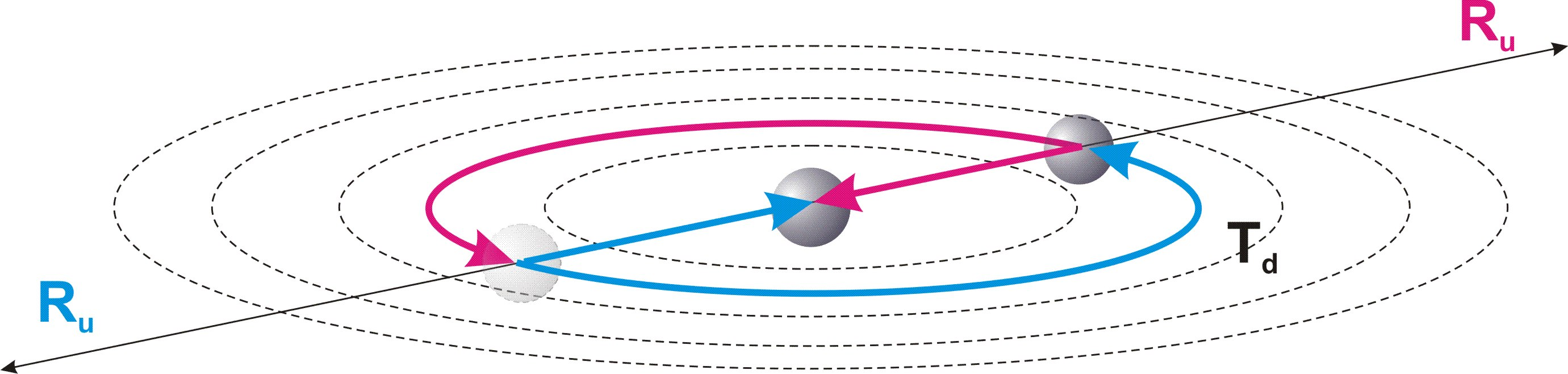Fig. 15.1.

In accordance with the Conclusions (c7.17) and (в7.19), the center of the frame of reference is the point of refraction of physical space, relative to which its definite dimension Ru is projected by its two opposite directions. This condition is identically projected into the orbital cycle.

Thus, the half-cycle of the process of compensating for broken symmetry in this orbital cycle represents a displacement along the indefinite dimension Td by a half-circumference. After each half-cycle passing along a half-circumference, the direction of the definite dimension Ru, coinciding with the radius of the circle, is reversed, and the process continues along the second half-cycle of the orbital cycle. A complete cycle returns both bodies to their original state.

To this, it is necessary to add that in the fundamental frame of reference the material part breaks symmetry only along the mainstay dimension Tu, since it is not localized along the mobile dimension Td and, accordingly, is indefinite relative to its size. In the orbital cycle, symmetry breaking occurs along a definite dimension of physical space Ru, coinciding with its radius, and in the relativity of the mobile dimension Td, projected by the circle, both particles are symmetrical relative to each other.

Conclusion (c15.2):

The orbital cycle in the local frame of reference is identical to the main cycle in the fundamental frame of reference.

Conclusion (c15.3):

The half-orbit along the mobile dimension Td represents the full size of the indefinite dimension of the gravitational cycle, and the orbital radius coinciding with a definite dimension of physical space Ru is the full size of its definite dimension.

From the condition of identity of the main cycle and the gravitational cycle, the conclusion follows:

Conclusion (c15.4):

If the conditions that define the magnitude of symmetry violation in the main and orbital cycles coincide, the forces acting in them are equal.

In accordance with Conclusion (c6.3) in the fundamental frame of reference, all parts of the potential part of the main localization have locally been singled out along the mainstay dimension Tu.

In accordance with Conclusion (c7.1) in the fundamental frame of reference, all parts of the material part are not localized relative to it.

From these conditions it follows that in the fundamental frame of reference the material part, as one of the poles of the cycle, is acted upon by a force from all parts of the potential part, as its opposite pole, the number of which, in accordance with Conclusion (c3.2), is equal to the square of the defining integer n of localization.

The defining integer of an orbital cycle should be calculated similarly to definition (d3.2), as the ratio of the size R of its radius, as a definite dimension, to the size of presence of the fundamental particle rt, relative to which it is formed.

ng = R/rt (15.1), where R – radius of orbital cycle, ng – defining integer of the gravitational cycle.

ТThus, in order to fulfill the conditions of equality of forces of cycles, there must be ng2 fundamental particles at the opposite pole of the orbital cycle formed relative to the fundamental particle, fig. 15.2.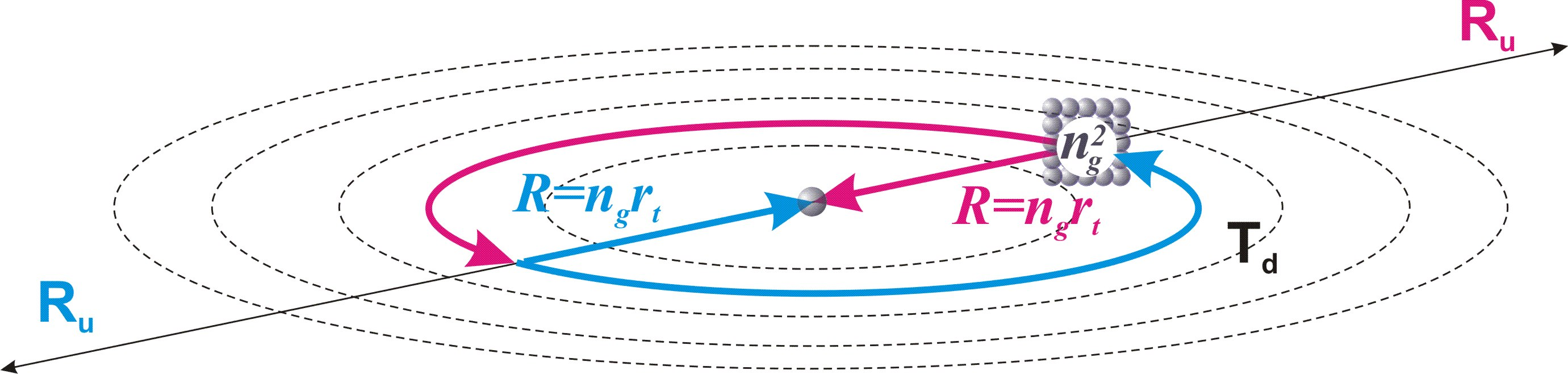Fig. 15.2.

Let us call this condition a complete orbital cycle.

Definition (d15.3):

A complete orbital cycle relative to a fundamental particle is an orbital cycle in which, at the pole opposite to it, there is a number of fundamental particles equal to the square of the defining integer ng of this cycle.

According to definition (d13.2), the definiteness of a particle along the definite dimension of the orbital cycle should be calculated by the ratio of its size R to the size of presence rtof the particle. But this definition refers to the main cycle, which is not restricted by any other conditions than its own defining integer n. The orbital cycle occurs within the restricting conditions of the main cycle, and represents of itself a singled out part of it. This definiteness of the orbital cycle in the main cycle should similarly influence the definiteness of the particle in it. Thus, we conclude:

Conclusion (c15.5):

The complete definiteness of a particle in the orbital cycle is defined by the product of the definiteness of the particle relative to the size of the orbital cycle and the definiteness of this cycle in the main cycle.

ug = (R/rt)( Rt/R) = Rt/rt = n (15.2), where ug – definiteness of the fundamental particle in the orbital cycle.

Conclusion (c15.6):

The definiteness of a fundamental particle relative to the orbital cycle, when its speed of motion is much less than the maximum possible speed c, does not depend from the size of the cycle and is equal to the defining integer n of the main localization.

The material definiteness of the material part in the fundamental frame of reference in accordance with formula (13.3) is similarly equal to the defining integer of localization n.

As we see, the conditions that determine the forces of the cycles coincide in the complete orbital cycle relative to the fundamental particle and in the main cycle in the fundamental frame of reference. It is important to note that for equality of forces it is necessary that the orbital cycle is not restricted by the additional condition of the presence of motion of the local frame of reference. Accordingly we come to the conclusion:

Conclusion (c15.7):

In the ideal frame of reference e, the force of a complete orbital cycle relative to the fundamental particle, if its speed of motion much less than the maximum possible speed c, is equal to the force of the main cycle in the fundamental frame of reference.

Taking into account formula (13.14) we obtain:

fgn = f0 = dmс2/πndr (15.3), where fgn – the force of influence of a complete orbital cycle on a fundamental particle in the ideal frame of reference.

This force represents the gravitational influence on one fundamental particle from ng2 fundamental particles of the opposite pole.

Accordingly, the force of gravitational attraction between two fundamental particles fg0 will be ng2 times less than the force of a full orbital cycle. In accordance with formula (15.1):

fg0 = fgn/ng2 = dmс2/ng2πndr = dmс2rt2/R2πndr (15.4)

Taking into account formula (8.1):

rt = drnt/n

fg0 = dmс2nt2dr /R2πn3 (15.5)

This force represents the force of gravitational attraction between two units of mass dm.

All components of this formula, except for the mass of the fundamental particle, do not change when moving from the ideal frame of reference to any local frame of reference. To this we add that the mass of any physical body consists of the masses of fundamental particles, their parts and projections.

Thus, the force acting between any two masses in any local frame of reference will be proportional to the ratio of each mass to unit of mass dm.

fg = (m1/dm)(m2/dm)dmс2nt2dr /R2πn3 = m1m2с2nt2dr /R2πn3dm (15.6)

Let us equate the resulting formula for the force of gravitational interaction with that accepted in physics:

Gm1m2/R2 = m1m2с2nt2dr /R2πn3dm (15.7)

We obtain the value of the gravitational constant:

G = с2nt2dr /πn3dm (15.8)

All components of this formula are constant quantities, except nt – the number of quanta of state of localization that have been passed.

Conclusion (c15.8):

The gravitational constant increases in proportion to the square of the number of the passed quanta of state nt.

An increase in the size of physical space during the main cycle is proportional to the number of the passed quanta of state nt, leading to a proportional increase in the size of gravitational cycles. Since the strength of the gravitational cycle is inversely proportional to the square of its size, and taking into account the increase in the gravitational constant is proportional to nt, we obtain a decrease in the strength of the gravitational cycle during the main cycle by nt times. Accordingly, the energy of the gravitational cycle, as the product of its size and the magnitude of the force, remains unchanged.

Conclusion (c15.9):

The potential energy of gravitational cycles in the local frame of reference does not depend on the stage of the main cycle and the number of the passed quanta of state nt.

Let us pay attention to the fact that if, in accordance with formula (8.3), in the gravitational constant formula we replace drnt2/n2 with rp, we obtain the formula: G=c2rp/πndm. Further, if we substitute in this formula instead of dm the known neutron mass and the value n = 2128, corresponding to the 8th order localization (formula (3.1)), we obtain a value for rp that coincides with the experimental value of the diameter of neutron within the frame of experimental error. Considering the orders of numbers used, this “coincidence” is more than enough to understand that we are on the right track. But what awaits us further are much more “surprising” coincidences of a number of theoretically obtained values with the corresponding experimental data.

16. Decay of a fundamental particle or beta decay of a free neutron

Content

As a result of the movement of the local frame of reference with speed vg along the indefinite dimension Td0 of the fundamental frame of reference, the direction of the mainstay dimension Tu relative to it has an angle of deviation φ0 from its direction in the fundamental frame of reference, fig. 16.1, a).

In accordance with theorem (t4) and Conclusion (c7.4), this condition for the motion of the local frame of reference is decisive for all processes in it, including the localization of fundamental particles.

Conclusion (c16.1):

The angle of deviation φ0 of the direction of the mainstay dimension Tu of the local frame of reference from its direction in the fundamental frame of reference is identically projected into the internal space of fundamental particles as the angle of deviation of the direction of the definite dimension ru in the relativity of the physical body from its direction in the relativity of the potential part.

As a result of this angle of deviation, the speed vp0 of movement of a physical body in the internal cycle of a fundamental particle along the definite dimension ru is projected as the speed vp of its movement along the indefinite dimension rd.

vp/vp0 = vg/c (16.1)

The increase in the size of the physical body of a fundamental particle rp for one instantaneous interval dt is equal to rt/n.

Accordingly, the speed of displacement of the physical body of a fundamental particle in a quantum of state along the internal definite dimension ru is equal to:

vp0 = rt/ndt (16.2)

Let us apply formulas (8.1) and (6.5):

rt = drnt/n

vp0 = rt/ndt = ntdr/dtn2= cnt/n2 (16.3)

We get value of the speed vp:

vp = vgvp0/c = vgnt/n2 (16.4)

Thus, in each quantum of state the physical body is given acceleration:

ap = vp/dt = vgnt/dtn2 (16.5), where ap – acceleration of the movement of a physical body along the dimension rd in the quantum of state.

Taking into account formula (6.5):

ap = vgnt/dtn2= vgntc/drn2 (16.6)

The time of physical processes is so much less than the time of a complete cycle of the Universe that the size of presence of the fundamental particle rt can be considered unchanged. Accordingly, the speed vp and acceleration ap remain unchanged.

In accordance with Conclusion (c8.12), the physical body of a fundamental particle is simultaneously in the conditions of the projection of the internal cycle of elementary localization and in the conditions of physical space, the movement along which is carried out along the mobile dimension Td.

Thus, taking into account the Conclusion (c8.9), the movement of a physical body in the internal space of a fundamental particle along the indefinite dimension rd is projected into physical space by movement along the mobile dimension Td, and the physical body acquires in the quantum of state the speed vp of movement along it, fig. 16.1, b).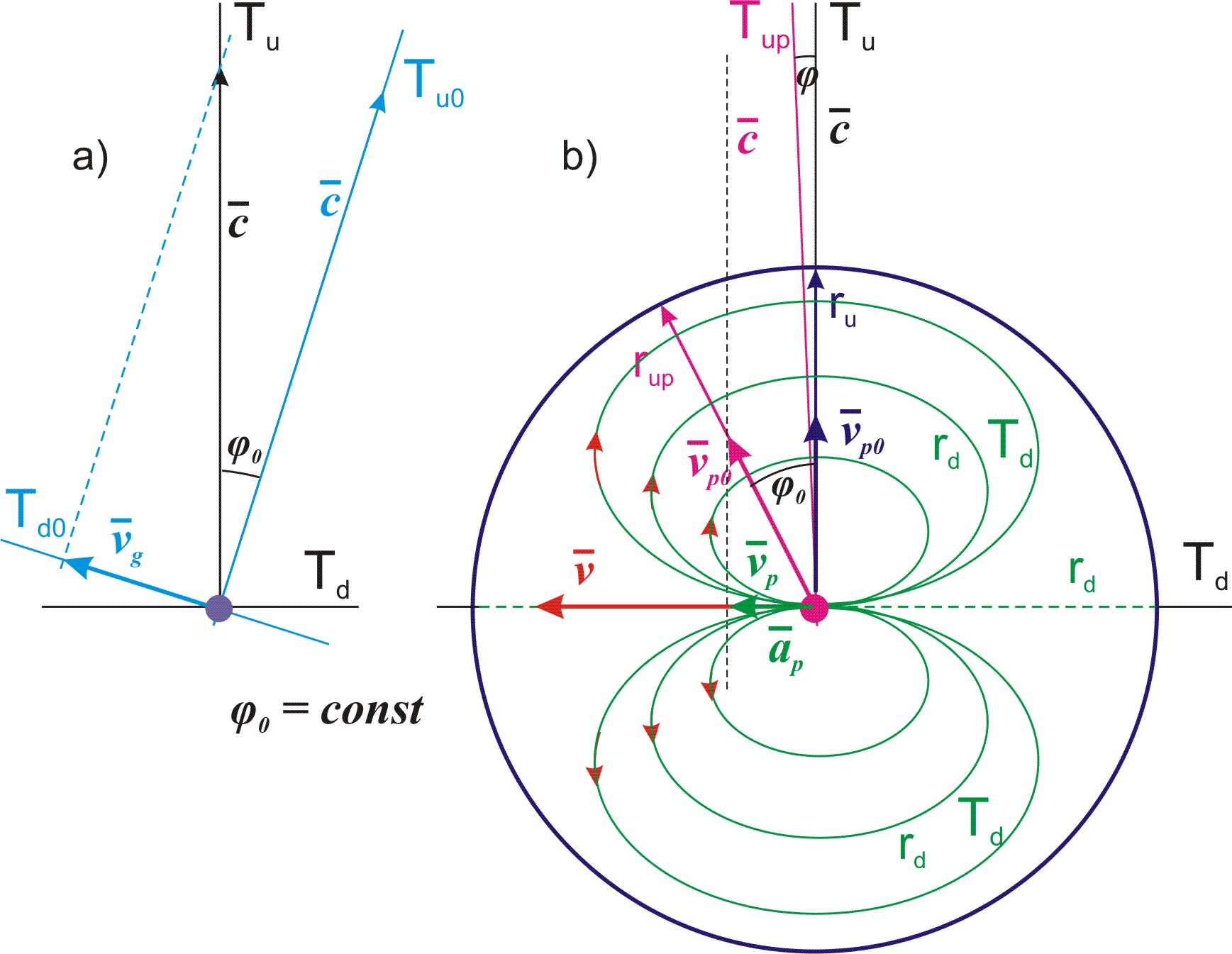Fig. 16.1.

As a result of this movement of the physical body along the mobile dimension Td with speed vp, the direction of the mainstay dimension Tu relative to it is deviated and the speed gained in the quantum of state is maintained. The entire process of gaining speed is repeated in each subsequent quantum of state, and the movement of the physical body along the mobile dimension Td becomes uniformly accelerated. The speed v of this movement is extremely small compared to the speed c, therefore the angle of deviation φ of the mainstay dimension Tu is much smaller than the angle φ0 and does not affect the projection of the acceleration ap onto the mobile dimension Td.

Conclusion (c16.2):

The movement of the local frame of reference relative to the fundamental frame of reference is projected into the internal space of the fundamental particle by the uniformly accelerated movement of its physical body along the mobile dimension Td of the physical space.

This movement of a physical body with acceleration ap along the mobile dimension Td occurs in the region of indefiniteness, which is a projection of the internal space of the fundamental particle into physical space. From these conditions, the conclusion follows:

Conclusion (c16.3):

The uniformly accelerated motion of the physical body of a fundamental particle along the mobile dimension Td within the indefiniteness of the internal space of the fundamental particle does not affect its inertial motion in the local frame of reference.

In accordance with Conclusions (c8.4) and (в5.10), the total maximum size of the area of presence a physical body along the indefinite dimension rd of elementary localization is equal to its full size πdr.

Thus, as a result of a physical body of a fundamental particle passing along the mobile dimension Td of a distance equal to the maximum size πdrof the area of indefiniteness, it will leave it. As a result, the fundamental particle, as the projection of elementary localization, will disintegrate along the mobile dimension Td into two separate parts: a separate projection of its material part and a separate projection of its potential part. From this follows the conclusion:

Conclusion (c16.4):

As a result of the displacement relative to each other of the projections of the main parts of the elementary localization along the mobile dimension Td of the physical space, its definite dimension ru is projected onto it.

The second indefinite dimension rd of the fundamental particle, in accordance with the conclusion (c8.9) before its decay was in conditions of indefiniteness regarding both indefinite dimensions Rd and Td of physical space. After projecting the definite dimension ru onto the mobile dimension Td, the indefinite dimension rd is projected perpendicular to it onto the indefinite dimension Rd.

Conclusion (c16.5):

As a result of the physical body of a fundamental particle leaving the area of indefiniteness formed by the projection of the potential part of the elementary localization, its internal definite dimension ru is projected onto the mobile dimension Td, and the indefinite rd onto the indefinite dimension Rd of physical space.

From the condition of identity of dimensions ru and rd (теорема (т2)) it follows that when the physical body of a fundamental particle is displaced along the projection of the indefinite dimension rd of the potential part of an elementary localization onto its full size πdr, in accordance with the conclusion (c7.5) the physical body must turn over relative to the two-dimensional structure rurd of the potential part of a fundamental particle.

Such an overturn regarding the projection of the potential part of the elementary localization must be accompanied by an overturn regarding the physical space itself. However, the physical body is part of the material part of the main localization, and cannot turn over relative to its two-dimensional structure RuRd.

As a result of these conditions, there is an overturn of the projection of the potential part of elementary localization into physical space.

Conclusion (c16.6):

The exit of the physical body of a fundamental particle from the area of indefiniteness is accompanied by an overturn of the projection of the two-dimensional structure rurd of the potential part of the elementary localization relative to the sides and directions of the two indefinite dimensions Td and Rd of the physical space.

In the process of this overturn, a local singling out of a part of the physical space occurs, which gives rise to a new elementary particle. The logic of the process leaves no doubt that the newly formed elementary particle is an antineutrino, and the fundamental particle is a free neutron. The physical body of a neutron released into physical space becomes a proton, and the separated potential part becomes an electron.

Conclusion (c16.7):

The fundamental particle of our Universe, as localization in Limitlessness, in the relativity of the local frame of reference associated with the Earth, is a free neutron.

Conclusion (c16.8):

The beta decay of a free neutron, as a fundamental particle, into two separate parts with the formation of a new elementary particle occurs due to the movement of the local frame of reference associated with the Earth.

Let us find the lifetime tn of a free neutron, as a fundamental particle, before its decay. According to the formula for the distance traveled in uniformly accelerated motion and found by us formula (16.6):

πdr = aptn2/2 (16.7)

tn =(2πdr/ap) =(2πdr2n2/cntvg) = ndr(2π/cntvg) (16.8)

Conclusion (c16.9):

The time interval tn = ndr(2π/cntvg) represents the lifetime of a free neutron before its decay.

In the following chapters, we will confirm all the conclusions drawn with accurate calculations based on experimental data.

17. Proton and electron

Content

In the previous chapter, we came to the conclusion that the physical body of a fundamental particle, as a projection of the material part of elementary localization into physical space after the decay of the fundamental particle, is a proton.

In accordance with Conclusion (c16.6), as a result of the decay of a fundamental particle, the direction of the projection of the definite dimension ru of the potential part of the elementary localization in relation to the two main parts of the decay changes to the opposite.

Conclusion (c17.1):

The proton represents a separate projection of the material part of an elementary localization into physical space, after the decay of a fundamental particle, with the direction of the projection of its internal definite dimension ru inverted to the opposite direction.

The decay of a fundamental particle does not impose additional conditions on the size rp of the physical body, which is determined solely by the stage of the main cycle.

Conclusion (c17.2):

The size rp of the physical bodies of a neutron and a proton is the same.

A definite dimension Ru of the material part of the main localization in its first half-cycle is projected into the physical space with the “from inside to outside” direction, corresponding to its expansion.

Identical to this, the direction “from inside to outside” projects into physical space the direction of the internal definite dimension ru of the potential part of an elementary localization in the relativity of the fundamental particle.

Thus, the change in the direction of the projection of the definite dimension ru in relation to a proton represents a change in direction from “from inside to outside” to “from outside to inside”.

Conclusion (c17.3):

The movement of a proton along the internal cycle of elementary localization is projected into physical space by the “from outside to inside” motion.

The decay of a free neutron occurs due to the displacement of its physical body along the projection of the internal indefinite dimension rd onto the mobile dimension Td.

Until the moment of decay, a neutron represents in physical space a spherical area of indefiniteness in the position of its physical body (conclusion (c8.11)), the size of which along the internal indefinite dimension rd in the quantum of state nt is equal to πrt.

The moment a physical body leaves this area of indefiniteness along the mobile dimension Td is associated with indefiniteness regarding its physical size rp.

Thus, the size of the area of presence of a proton along its indefinite dimension rd increases to the size πrt + rp, fig. 17.1.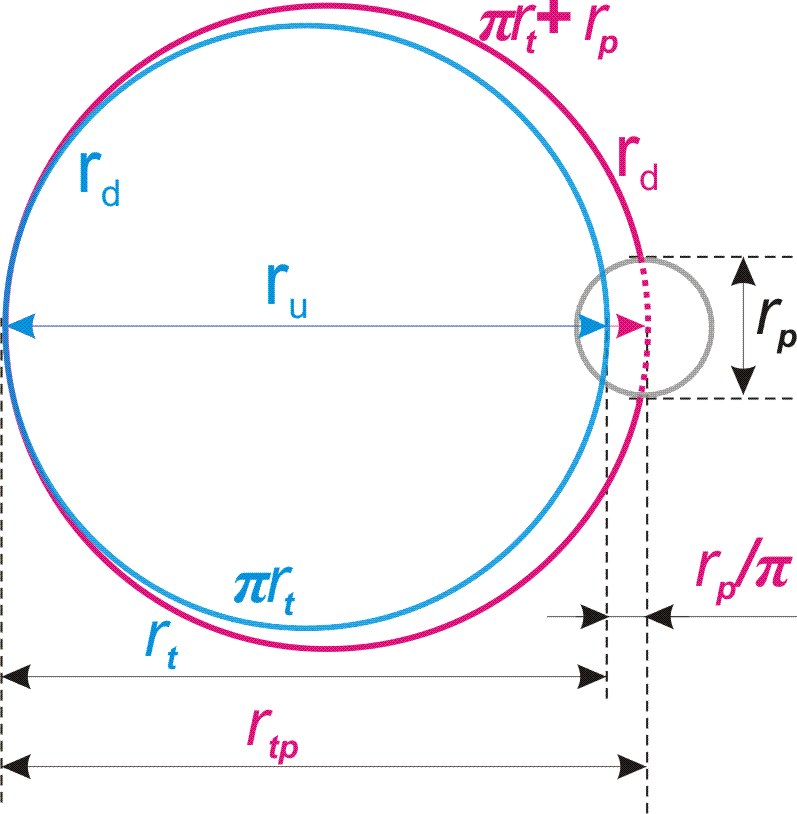Fig. 17.1.

Considering these conditions, we find the size of the area of presence of a proton:

rtp = (πrt + rp)/π = rt + rp/π (7.1), where rtp – the size of the area of presence of a proton.

Taking into account formula (8.3):

rp = rtnt/n

rtp = rt (1 + nt/nπ) (7.2)

Conclusion (c17.4):

A proton is a spherical area in physical space with a diameter rtp of the indefiniteness of the position of its physical body.

Unlike the neutron, the size of the area of presence of a proton is not restricted by the conditions of the cycle, and can vary depending on external influences.

Conclusion (c17.5):

The size of the area of presence of a proton is located, and, consequently, its mass, is not a constant value, but a statistical one.

From the condition of internal indefiniteness of the area of presence of a proton, the conclusion follows:

Conclusion (c17.6):

The proton corresponds to the definition (d13.1) of an elementary particle.

Continuing the logic of neutron decay, a separate projection of the potential part of an elementary localization must represent an electron.

In accordance with the Conclusion (c8.6), until the moment of decay of a fundamental particle, the position of the beginning and end of its definite dimension ru along which the process of compensation for broken symmetry moves, is indefinite relative to its internal space. Thus, the internal space of a fundamental particle represents an area of indefiniteness not only regarding the location of the physical body, but also regarding the boundary of the end of the half-cycle of elementary localization.

After the decay of a fundamental particle, taking into account the Conclusion (c16.6), an electron represents the area in physical space where the boundary of the end of the half-cycle is located, which projected in the direction “from outside to inside”, fig. 17.2.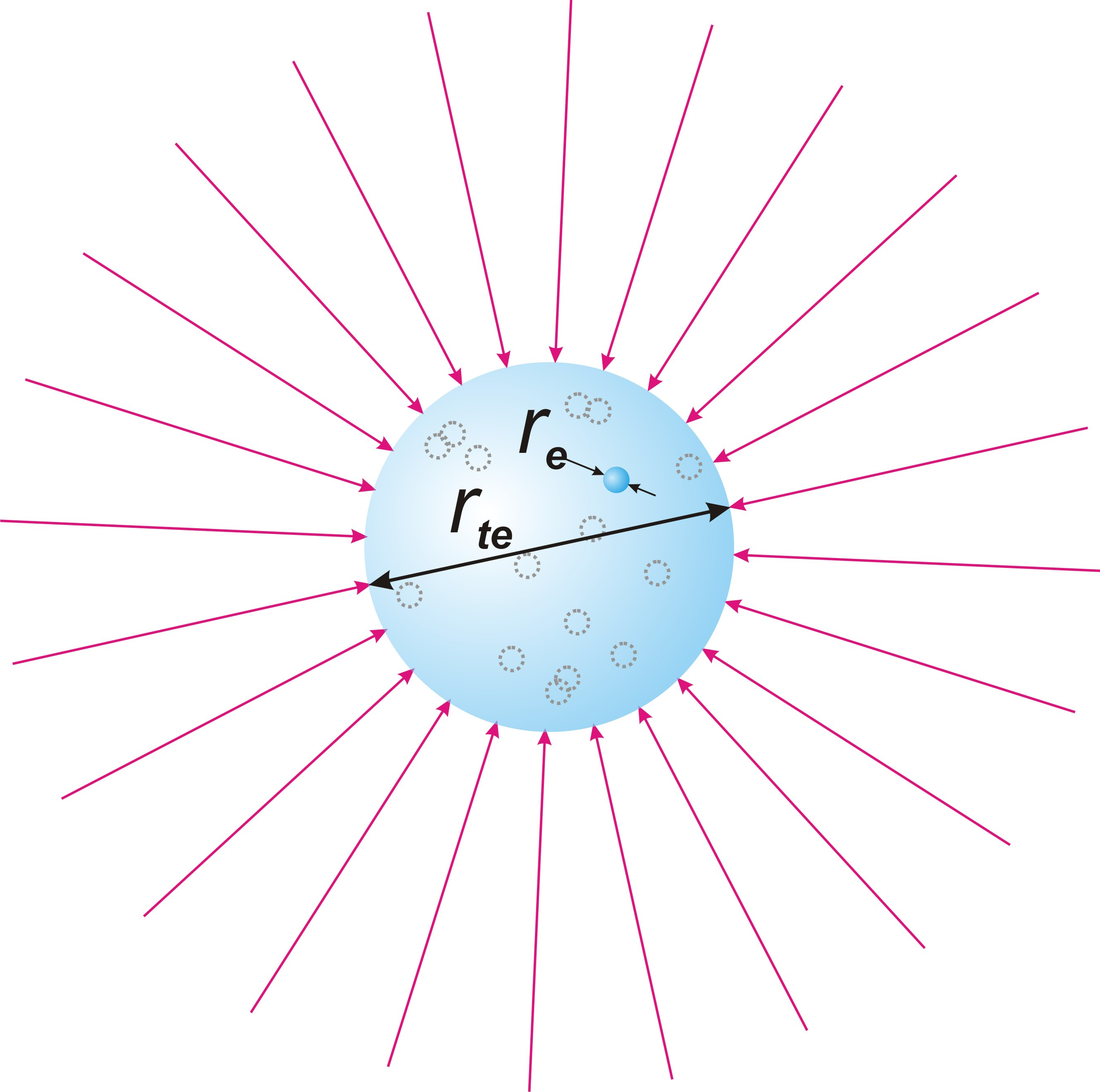Fig. 17.2.

Conclusion (c17.7):

An electron is an elementary particle and represents an area in physical space where the boundary of a half-cycle of elementary localization is located, and projection of the direction of movement of the process is in reverse position as “from outside to inside”.

The size of the final state of elementary localization in the backward projection of the half-cycle is equal to the size of the initial state in its forward projection for nt = 1.

The electron, as a projection of the potential part of the elementary localization, does not have a physical body, like a proton or a neutron, but in a certain convention, this size of the fundamental particle in the first quantum of the state of the main cycle can be considered the physical size (diameter) of the electron, since it is this that is reflected in physical experiments , when reducing its area of presence to a specific alternative position.

Definition (d17.1):

The physical size of an electron is the size corresponding to the size of the material part of the main localization in the first quantum of the state of its cycle at nt = 1.

Since in the first quantum of the state Rt = dr, we find the physical size of an electron:

re = dr/n = const (17.3), where re – physical size of an electron.

Conclusion (c17.8):

The physical size of an electron is constant throughout the entire localization cycle of the Universe.

At the moment the physical body of a neutron leaves the area of indefiniteness, which is a fundamental particle, the entire path traversed by the physical body in the area of indefiniteness is superimposed on the mobile dimension Td by a linear segment.

In accordance with the Conclusions (c5.16) and (в8.1), the movement of a physical body along an indefinite dimension rd is carried out simultaneously in both opposite directions to the full value of its size πdr.

Identical to the projection of the mobile dimension Td into physical space in the unfolded projection relative to its two directions, this path, traversed simultaneously in opposite directions, is projected onto the mobile dimension Td in the unfolded projection.

Conclusion (c17.9):

At the moment the physical body leaves the area of indefiniteness formed by the projection of the potential part of an elementary localization, the entire path traveled along two opposite directions of the indefinite dimension rd is projected onto the mobile dimension Td by a linear segment in an unfolded projection in two opposite directions of size πdr with a refraction point between them.

In this case, in accordance with Conclusion (c16.5), the definite dimension ru of the potential part of an elementary localization is projected onto the mobile dimensionTd.

Conclusion (c17.10):

A definite dimension of the internal space of an electron is an unfolded projection of two opposite directions of the definite dimension ru of the potential part of an elementary localization.

The path traversed by a physical body in the internal space of a fundamental particle is projected with the indefiniteness of the size of presence πrt of the physical body along the indefinite dimension rd in relation to the size πdr of each of the two opposite cycles, fig. 17.3.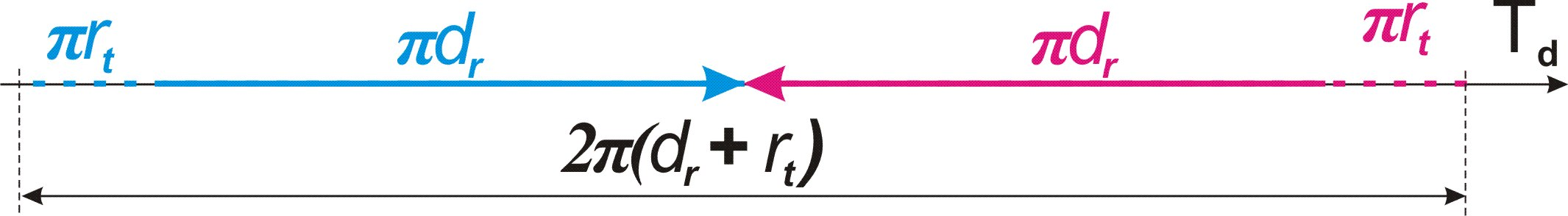Fig. 17.3.

Thus, the size 2πdr + 2πrt is the determining condition for the area of presence of the electron at the time of its formation as an independent elementary particle.

rtev = 2πdr + 2πrt = 2π(dr + rt) (17.4), where rtev – the size of presence of the electron at the time of its formation along the mobile dimension Td.

Taking into account formula (8.1):

rtev = 2π(dr + rt) = 2πdr(1 + nt/n) (17.5)

The increase in the size of the area of presence of an electron from rt to rtev cannot happen instantly. The maximum speed of movement in physical space cannot exceed c (conclusion (c6.6)). At the same time, it is necessary to take into account that the internal indefinite dimension rd is projected onto the direct path along the mobile dimension Td, by its reversal, fig. 17.4.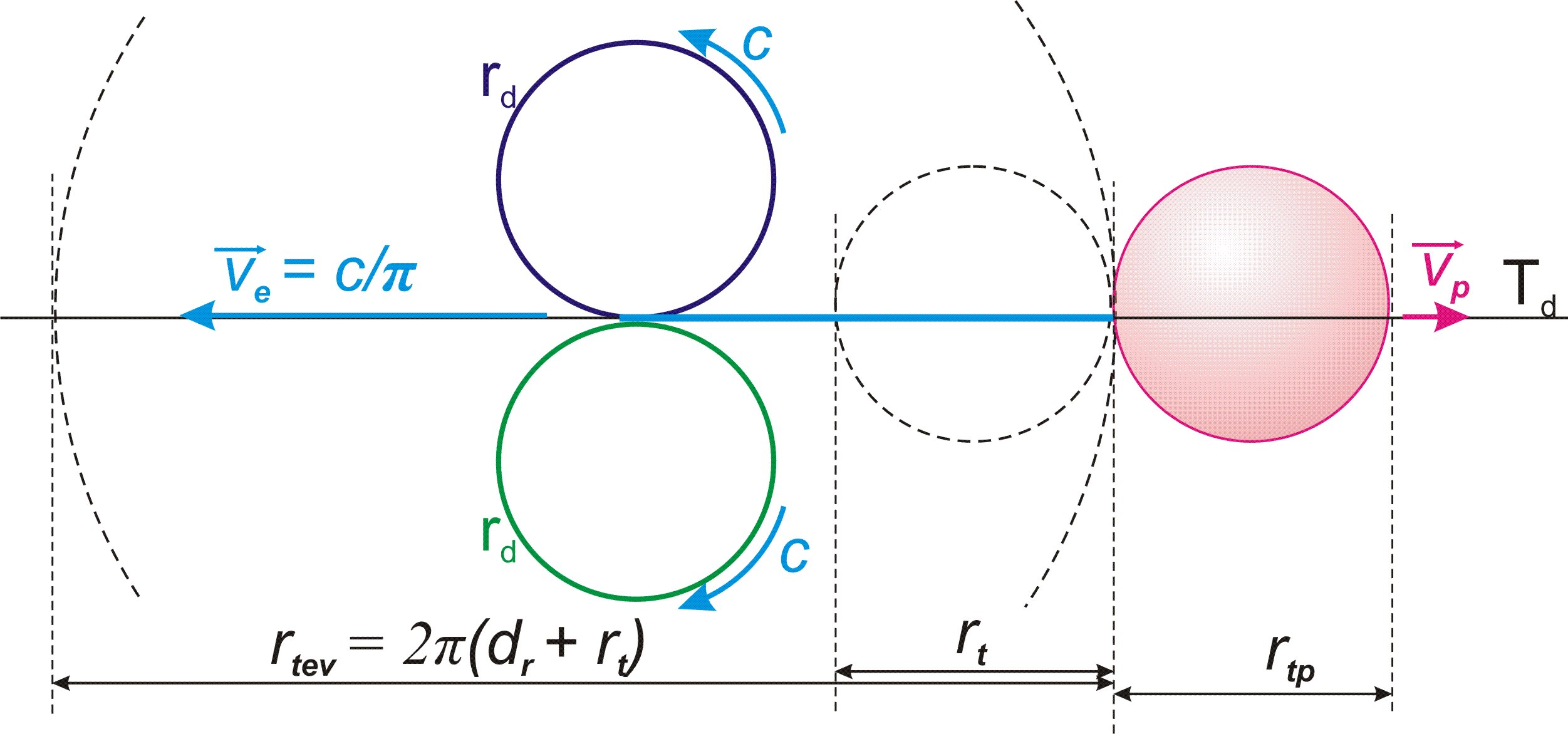Fig. 17.4.

The ratio of the proton mass to the electron mass is quite large, and the speed vp that it acquires in the process of “pushing” with the electron can be neglected

Thus, taking into account the maximum speed of movement c, the electron in the process of its growth acquires the speed c/π of its movement along the mobile dimension Td, in the local frame of reference associated with the Earth.

In accordance with formula (11.5), we find the size of presence of an electron at rest.

rte = rtev/(1-c2/π2c2) = rtev/(1-1/π2) = 2πdr(1 + nt/n)/(1-1/π2) (17.6), where rte – the size of presence of an electron at rest.

18. Electrical forces

Content

The force of the internal cycle of elementary localization is projected into physical space by the force of interaction between a proton and an electron.

Using terms accepted in physics, we come to the following conclusions:

Conclusion (c18.1):

Electrical forces represent the projection of the force of the internal cycle of elementary localization into physical space.

Conclusion (c18.2):

Electric charge is an indivisible potential of electrical force relative to one of the two main parts of elementary localization.

In its own relativity, the electron moves along the projection of the definite dimension ru of the potential part of elementary localization in the direction opposite to the proton. Thus, in accordance with Conclusion (c17.3), the dimension ru is projected in relation to the electron in direct projection – a movement “from inside to outside”.

Since traditionally the electric charge of a proton is considered positive and that of an electron negative, the conclusion follows:

Conclusion (c18.3):

A negative charge is defined by the direct projection of the direction of the definite dimension ru of elementary localization into physical space, and a positive charge by its reverse projection.

From the principle of symmetry and identity (theorem (t2) and conclusion (c1.2)) it follows:

Conclusion (c18.4):

The repulsive force of two charges of the same sign is equal in magnitude to the attractive force of two charges of the different sign.

To find the formula for the force of electrical interaction, it is necessary to find the force of the internal cycle of a fundamental particle.

In accordance with formula (16.2), the speed vp0 of movement of the physical body of a fundamental particle along its internal cycle in the quantum of state is equal to rt/ndt. Accordingly, its acceleration in the quantum of state is equal to:

ap0 = vp0/dt = rt/ndt2 (18.1)

In accordance with formula (13.1):

fn = kupap0 = kuprt/ndt2 (18.2), where fn – force of the inner cycle of a fundamental particle, up - material definiteness of the physical body of a fundamental particle in its internal cycle.

The material definiteness (definitions (d13.3) and (d13.2)) of a physical body in the relativity of physical space is defined relative to its size of presence, which is the size of the entire fundamental particle. In the relativity of the internal cycle, its material definiteness increases in connection with its definiteness relative to the potential part of elementary localization and should be calculated as the product of its material definiteness in relation to physical space by its definiteness relative to the internal cycle of elementary localization.

Along the indefinite dimension rd of the potential part of elementary localization, the physical body has not been singled out, and, accordingly, its definiteness along this dimension is equal to 1.

Along the definite dimension ru of the potential part of elementary localization, the size of the physical body of a fundamental particle is defined as the ratio of the size of presence of a fundamental particle rt to the defining integer n, fig. 18.1.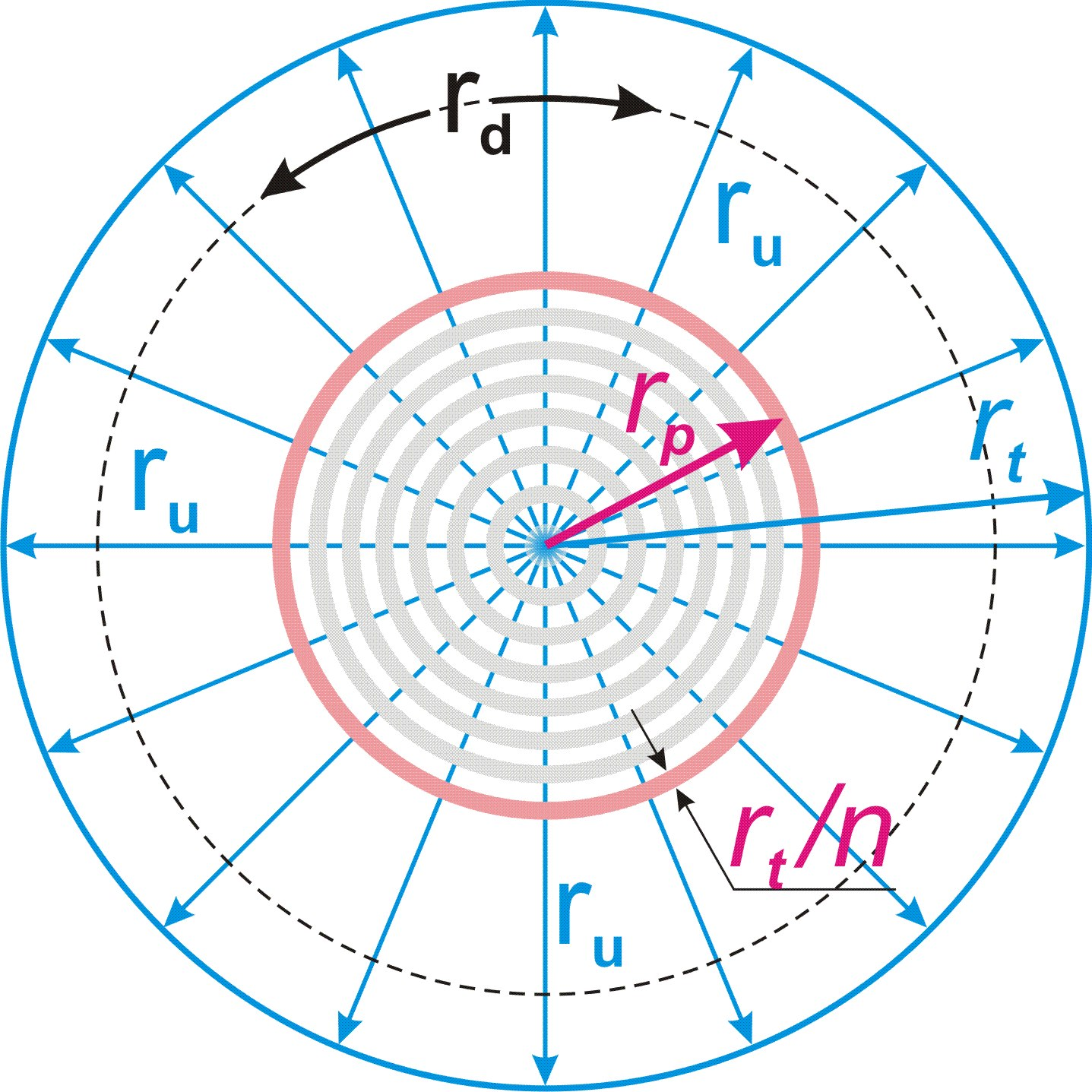Fig. 18.1.

Thus, we come to the conclusion:

Conclusion (c18.5):

The material definiteness of the physical body of a fundamental particle in its internal cycle is n times greater than its material definiteness in the main cycle.

In accordance with formula (13.4):

un = πn2/(1-vg2/c2)

up = nun = πn3/(1-vg2/c2) (18.3)

Taking into account formulas (18.2) and (13.7) for the force of the inner cycle, we obtain:

k = dm/πn2

fn = kuprt/ndt2 = kπn2rt/dt2(1-vg2/c2) = dmrt/dt2(1-vg2/c2) (18.4)

Taking into account formula (13.8):

dmv = dm/(1-vg2/c2)

fn = dmrt/dt2(1-vg2/c2) = dmvrt/dt2 (18.5)

Let us apply formula (8.1) and (6.5):

rt = drnt/n

c = dr/dt

fn = dmvrt/dt2 = dmvdrnt/ndt2 = dmvc2nt/ndr (18.6)

It is also necessary to take into account that the force acting along the internal defined dimension ru is projected onto the mobile dimension Td, which is indefinite.

The indefinite dimension Td is π times longer in size than the definite Tu. According to the condition of symmetry, the work of the cycle force to displace the material part on a full half-cycle along each of the dimensions should be the same. From this follows the conclusion:

Conclusion (c18.6):

The effect of the force of the cycle of compensation for broken symmetry along an indefinite dimension is π times weaker than along a definite one.

fen = fn/π = dmvc2nt/πndr (18.7) , where fen – projection of the force of the internal cycle of a fundamental particle into physical space.

This force will act between the projections of the two main parts of elementary localization at the moment of decay of a fundamental particle. Thus, the distance at which such magnitude of the electrical interaction force will act is equal to its size of presence rt.

This interaction at a distance rt corresponds to a half-cycle with a defining integer equal to 1. Thus, the size rt represents a quantum of extension of the electrical cycle, and, identically to the gravitational cycle (formula (15.1)), we can find the defining integer ne of an electrical cycle of any size:

ne = R/rt (18.8), where R – distance between interacting charges.

Taking into account formula (8.1):

ne = R/rt = Rn/ntdr (18.9)

Similar to the gravitational cycle (Chapter 15), the same force fen must act on a unit charge by ne2 unit charges of the other pole in an electric cycle of any size.

We obtain the formula for the force of interaction between two charges at a distance R:

fe0 = fen/ne2 = dmvc2nt/πndrne2 = dmvc2nt3dr/πn3R2 (18.10)

Since increasing the charge on each side correspondingly increases the force of interaction, this force is proportional to the product of the number of unit charges on each pole:

fe = (q1/q0)(q2/q0)fe = q1q2fe/q02 = q1q2dmvc2nt3dr/πn3q02R2 (18.11), where q0 — unit charge or coefficient of a system of measures, q1 и q2 — interacting charges.

Let us compare it with the well-known formula for electrical interaction in physics:

fe = Qq1q2/R2 , where Q — the electrical constant.

We obtain the value of the electrical constant:

Q = dmvc2nt3dr/πn3q02 (18.12)

This formula contains a variable value of the number of quanta of state nt traversed by the Universe.

Conclusion (c18.7):

The electric constant grows proportionally to the cube of the number of quanta of state nt traversed by the Universe.

Considering that the number of opposite charges in the relativity of any local frame of reference is equal to the number of separate projections of the two main parts of elementary localizations, we come to the following conclusion:

Conclusion (c18.8):

In the relativity of any local frame of reference, the number of positive charges is equal to the number of negative ones.

Let us pay attention to the fact that dividing the formula (18.12) of the electric constant by the formula (15.8) of the gravitational constant, we get the formula for the number of quanta of state nt traversed by our Universe:

Q/G = dmvdmnt/q02 (18.13)

nt = Qq02/Gdmvdm (18.14)

If we neglect the difference between the neutron mass dmv in the local frame of reference associated with the Earth and its mass dm in the ideal frame of reference, then we easily find the value for nt as the ratio of the force of the electrical interaction of two unit charges to the force of the gravitational interaction of two neutrons. We get the value:

nt ≈1.2•1036

The degree of the obtained number leaves no doubt that our Universe is eighth-order localization (formula (3.1)) with the defining integer:

n = 2128 ≈ 3.40•1038 (18.15)

It was this finding (only instead of the mass of the neutron, the mass of the proton was used) that suggested to the author the direction of all further research. As a result, much more accurate values were obtained, including the speed vg of the motion of the local frame of reference associated with our planet, the result of which is the difference between the mass of the neutron dmv and its theoretically calculated mass dm in the ideal frame of reference. Therefore, we will not rush with calculations, and we will first find some more important formulas that will allow us to obtain the theoretical value of the neutron mass.

19. Hydrogen atom

Content

When a proton, moving towards the projection of the boundary of a half-cycle of elementary localization (conclusion (c17.7)), enters the internal space of the electron and passes a distance in it that exceeds the size πdr of the projection of the definite dimension ru, its direction, in accordance with Conclusion (c17.10), changes to the opposite, fig. 19.1, a).

Thus, oscillatory movements of the projection of the boundary of the elementary localization cycle relative to the proton arise in the internal space of the area of presence of the electron, creating a stable state of the hydrogen atom.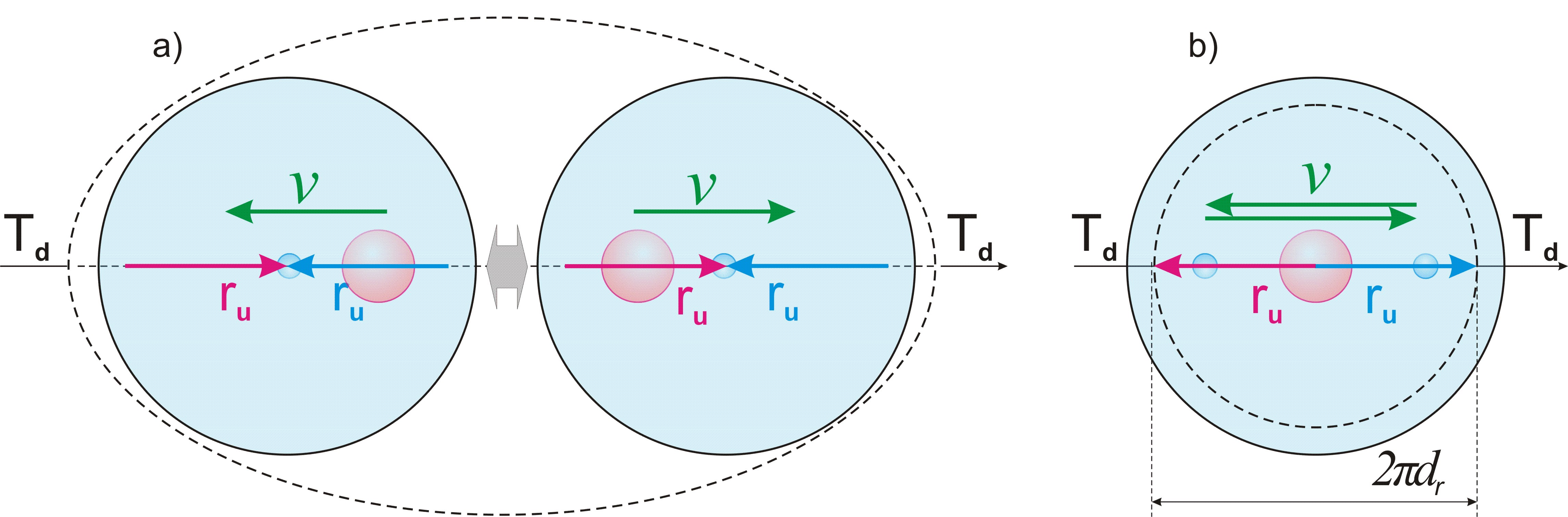Fig. 19.1.

This oscillatory process, occurring in the area of indefiniteness of electron, is a holistic projection of the internal cycle of elementary localization and is not reflected in physical space by the movement of charges, fig. 19.1, b).

Only the oscillatory movements of the proton and electron compound as an integral material object are reflected in physical space.

Conclusion (c19.1):

The hydrogen atom represents a holistic projection of the internal cycle of elementary localization into physical space, as a stable process of oscillations of the projection of the boundary of the elementary localization cycle relative to the proton in the internal space of area of presence of the electron.

Conclusion (c19.2):

The internal oscillation process of the hydrogen atom is not reflected in physical space by oscillations of electric charges.

Conclusion (c19.3):

The size of an hydrogen atom is determined by the size of the area of presence of an electron, which, in turn, is determined by the minimum value of two sizes of the indefinite dimension rd of the potential part of an elementary localization, and in their sum is equal to 2πdr.

The increase in this size due to the loss of energy by the electron is insignificant. Thus, we must come to an important conclusion:

Conclusion (c19.4):

The sizes of atoms during the cycle of the Universe remain unchanged, while the size of the Universe increases by n = 2128 times.

From this conclusion it follows that the sizes of solid cosmic bodies, such as our planet, change slightly during the cycle of the Universe, and the cosmic distances between them increase. Thus, taking into account the Conclusion (c15.8), we come to the following conclusion:

Conclusion (c19.5):

The acceleration of free fall on the surface of the planet increases in proportion to square of the quanta of state nt traversed by the Universe.

This increase in forces squeezing the planet is perhaps one of the main reasons for the internal heating of the planet.

20. Photon and Planck's constant

Content

If the oscillatory motion of the cycle boundary in the area of presence of an electron in one direction relative to the proton reaches a size of 2πdr, this corresponds to the displacement of the proton, as a projection of the material part of an elementary localization, onto a full cycle of two half-cycles along the unfolded projection of its internal definite dimension ru. As a result, in accordance with Conclusion (c8.7), the proton must roll over by the full cycle relative to the internal space of the electron, determined by the projection of the two-dimensional structure rurd of the potential part of elementary localization.

However, as has already been noted, the physical body is part of the material part of the main localization and cannot roll over relative to the two-dimensional structure TdRd of physical space, onto which the dimensions ru and rd are projected.

As a result of these conditions, a rollover of part of the physical space which is an electron occurs relative to the two-dimensional structure of TdRd.

The possibility of such a rollover is ensured by the condition of indefiniteness regarding the physical space of both dimensions Td and Rd.

Conclusion (c20.1):

Under conditions of indefiniteness of the dimensions Td and Rd, their local symmetrical rollover relative to their main and secondary parts is possible.

Since the electron is an unfolded projection of two half-cycles of elementary localization, this rollover must correspond to a complete cycle, that is, consist of two half-cycles going simultaneously in opposite directions, fig. 20.1.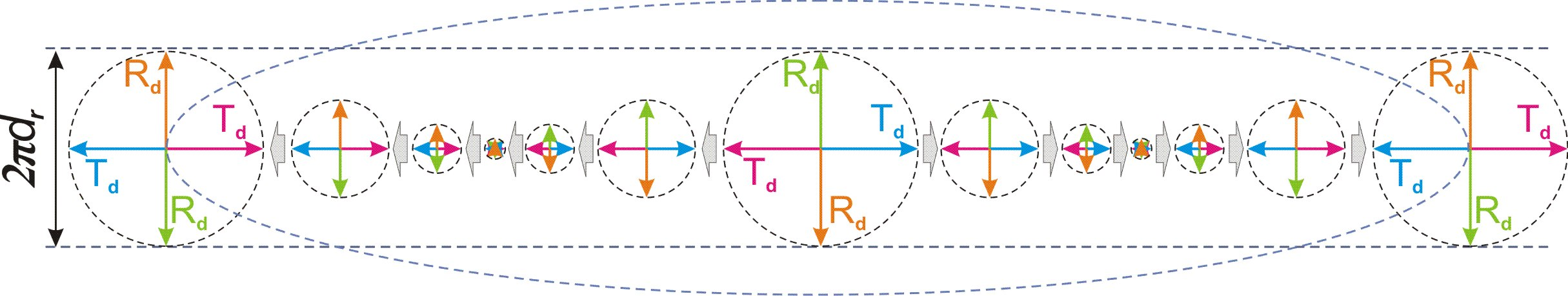Fig. 20.1.

This process of rollover a part of the physical space produces its local singling out along the dimensions Td and Rd of the physical space, which generates a new elementary particle. It is easy to guess that in this case we are dealing with the photon.

Conclusion (c20.2):

A photon is a locally singled out part of the physical space, due to its rollover on a full cycle relative to the two-dimensional structure of mobile Td and indefinite Rd dimensions of the physical space.

The rollover of an electron by a full cycle relative to the physical space returns it to its original position relative to it, but at the same time, it loses part of its mass spent on the release of a photon.

Conclusion (c20.3):

As a result of the release of a photon, the size of presence of the electron increases and its mass decreases.

The conditions of indefiniteness of the two-dimensional structure of two indefinite dimensions Td and Rd are identical to the conditions of the main localization in the first act of singling out its material part.

Conclusion (c20.4):

The release of a photon relative to the two-dimensional structure TdRd of two indefinite dimensions of physical space breaks the symmetry relative to it and causes a cycle of compensation for the broken symmetry, identical to the main cycle.

Thus, a photon must move along one of the dimensions of the two-dimensional structure TdRd with the maximum possible speed c.

Since movement in physical space is possible only along the mobile dimension Td, a photon is displaced in quanta of state along it.

Conclusion (c20.5):

The movement of a photon is carried out along the mobile dimension Td of the physical space with the maximum possible speed c.

Thus, relative to the photon, the mobile dimension Td is projected as a definite dimension of the two-dimensional structure TdRd along which it moves. In this case, the condition of definiteness is imposed on the dimension in the conditions that already exist and define it and, according to theorem (t4), cannot change them.

Conclusion (c20.6):

In photon relativity, the mobile dimension Td is definite, and the indefinite dimension Rd of physical space is indefinite, but at the same time their size is the same and is determined by the size πRt of physical space along the indefinite dimension Rd.

The entire complete cycle of a photon is projected onto a mobile dimension Td by a size rγ, which by definition (d12.2) represents a quantum of extension of the process, or, as it is commonly called, a quantum of light, fig. 20.2, a).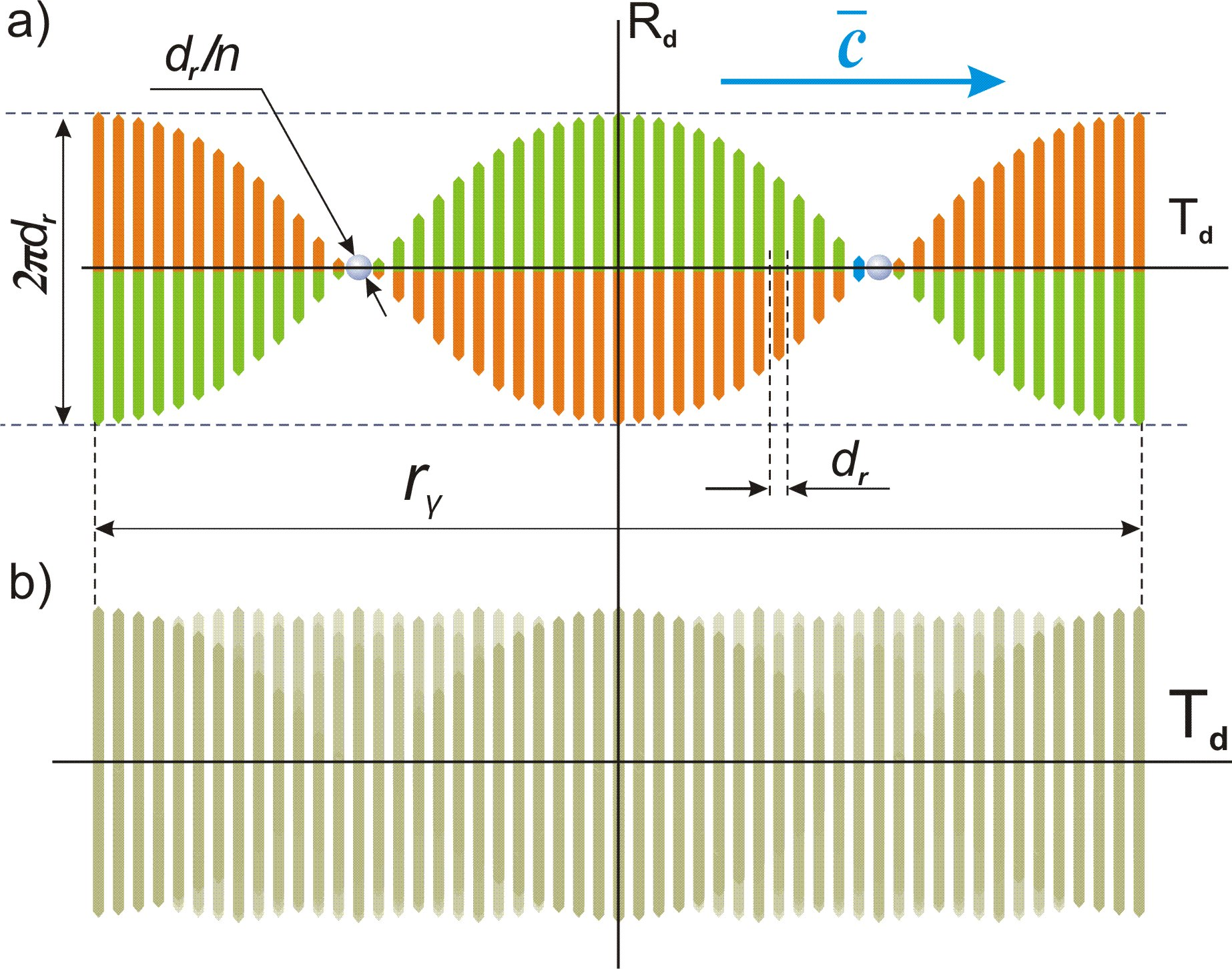Fig. 20.2.

In accordance with Conclusion (c12.2), the sequence of parts of the process within the quantum of extension rγ is indefinite, fig. 20.2, b).

Conclusion (c20.7):

The process of singling out a photon is one-moment and indefinite regarding the sequence of its parts.

This means that relative to the physical space, the internal space of a photon is symmetrical with respect to all its parts and meets the definition (d13.1) of an elementary particle.

Conclusion (c20.8):

A photon is an elementary particle.

An orientation of a photon relative to the indefinite dimension Rd of physical space defines its property, called polarization in physics.

Conclusion (c20.9):

The polarization of a photon is defined by the orientation in physical space of the direction of the indefinite dimension Rd relative to it.

Since the mobile dimension Td, which is indefinite, is projected relative the photon as a definite dimension, according to Conclusion (c7.19) its origin is projected to the center of any physical frame of reference. Considering that the photon moves along it with the maximum possible speed c, we come to a conclusion corresponding to the main postulate of the Theory of Relativity:

Conclusion (c20.10):

A photon moves with the maximum possible speed c relative to any physical frame of reference, regardless of the speed of its movement in physical space.

The size of a quantum of extension of a photon rγ is traditionally called its wavelength in physics.

rγ = сd (20.1), where d – the size of a quantum of extension of the process in time units.

A photon is locally singled out only along two dimensions Td and Rd, and is not defined along all the others; accordingly, its material definiteness in relation to the conditions of its movement must define relative to these two dimensions.

As will be shown below, the photon is not the only elementary particle, the process of compensating for broken symmetry with respect to which occurs along the two-dimensional structure TdRd. Therefore, let us write down a general rule:

Conclusion (c20.11):

A material definiteness of elementary particles, the process of compensation for broken symmetry with respect to which takes place along the two-dimensional structure of two indefinite dimensions Td and Rd of the physical space, is equal to the product of their definiteness along each of these two dimensions.

The size of a photon along the mobile dimension Td is equal to rγ, and its definiteness along this dimension in accordance with the definition (d13.2) is equal to πRt/rγ.

Along the second, indefinite dimension Rd of physical space, the size of the photon is defined by the unfolded projection of two opposite half-cycles of elementary localization along its internal indefinite dimension rd of the potential part and is equal to 2πdr. Accordingly, for each of the two opposite directions of this dimension, the photon is singled out with a size πdr.

Taking into account the size πRt of the indefinite dimension Rd, the definiteness of a photon according to it is equal to πRt/πdr.

Based on a definition (d13.2) and Conclusion (c20.11), we find the material definiteness of the photon:

uγ = (πRt/πdr)(πRt/rγ) = πRt2/drrγ (20.2), where uγ – the material definiteness of the photon.

Let us apply formula (6.4):

uγ = πRt2/drrγ = πnt2dr/rγ (20.3)

Let us find the mass of a photon using formula (13.2):

mγ = kuγ , where mγ – the mass of a photon.

Let us apply formula (13.7):

k = dm/πn2

mγ = kuγ = kπnt2dr/rγ = dmnt2dr/n2rγ (20.4)

A frequency of a photon in physics is the value of the ratio of the speed of its movement c to its wavelength rγ.

γ = c/rγ = 1/d (20.5) , where γ – a frequency of a photon.

A size of a photon does not depend by the stage of the main cycle. Accordingly, the photon frequency remains unchanged.

Conclusion (c20.12):

A frequency and size of a photon do not depend by the number of passed quanta of state nt and do not change during the main cycle.

An energy of a photon should be defined by the work of the force of the symmetry restoration cycle in its quantum of state when moving along the dimension Td:

eγ = mγaγrγ (20.6), where aγ – acceleration with which a photon gain the speed c in its quantum of state.

aγ = c/d (20.7)

Let us apply formula (20.1):

rγ = cd

eγ = mγaγrγ = mγc2 (20.8)

Taking into account formula (20.4):

eγ = mγc2 = dmc2nt2dr/n2rγ (20.9)

Let us replace the wavelength on frequency:

rγ = c/γ (20.10)

eγ = dmc2nt2dr/n2rγ = γdmcnt2dr/n2 (20.11)

Comparing this formula with the formula accepted in physics:

eγ = hγ, где h - the Planck's constant.

We get:

h = dmcnt2dr/n2 (20.12)

Conclusion (c20.13):

The value of Planck's constant and the photon energy grow in proportion to the square of the number of passed quanta of state nt.

21. The defining integer of the Universe and the mass of the fundamental particle

Content

Finally, we have obtained all the formulas we need, which make it possible, on the basis of experimental data of some physical characteristics of our physical world, to obtain the theoretical value of its other main characteristics and compare with their experimental value. This will allow not only to prove the correctness of our theoretical model, but also to obtain some completely new for science exact data on the physical characteristics of our Universe.

We divide formula (20.12) of the Planck constant by formula (15.8) of the gravitational constant:

h = dmcnt2dr/n2

G = c2drnt2/πn3dm

h/G = dm2πn/c (21.1)

dm2 = hc/Gπn (21.2)

dm =(hc/Gπn) (21.3)

We know all the components of this formula, except for the defining integer n of the localization of our Universe. We have already come to the preliminary conclusion in chapter 18 that our Universe is an eighth-order localization with the defining integer n = 2128 (formula (3.1) и (18.15)). Let us make a calculation relative to this value.

n = 2128

G = 6.67384(80)•10-11 m3/kgs2

c = 299 792 458 m/s

h = 6.62606957(29)•10-34 Js

We get the value of the unit of mass:

dm =(hc/Gπn) = 1.66861•10-27 kg (21.4)

Experimentally obtained neutron mass:

dmv = 1.674927351(74)•10-27 kg

Such a coincidence with a discrepancy of only 0.4% eaves no doubt that our Universe is an eighth-order localization with a defining integer n equal to 2128. It makes no sense to carry out calculations for other orders of localizations, since the difference will be dozens of orders of magnitude.

Conclusion (c21.1):

Our Universe is an eighth-order localization with a defining integer n = 2128.

22. The exact value of the speed of movement of the local reference frame associated with the Earth

Content

The slight difference between the unit mass dm and the neutron mass dmv is explained by the motion of the local frame of reference associated with our planet relative to the fundamental frame of reference. Using the previously obtained formula (13.8) for the increase in the mass of a fundamental particle (neutron) in a local frame of reference moving with speed vg relative to the fundamental reference frame, we can find the exact value of this speed.

dmv = dm/(1-vg2/c2)

1- vg2/c2 = (dm/dmv)2

vg2/c2 =1- (dm/dmv)2

vg2 =(1- (dm/dmv)2)c2

vg = c(1- (dm/dmv)2)= 26 013 292 m/s (22.1)

Conclusion (c22.1):

The local frame of reference associated with planet Earth moves relative to the fundamental frame of reference with the speed 26 013 292 m/s.

The fact that the found value of the speed of movement of the local frame of reference associated with the Earth corresponds to reality will be shown by further calculation on its basis of the decay time of a free neutron, which exactly coincides with the experimental one.

23. The number of quanta of state passed by the Universe

Content

As we already noted in chapter 18, the formula (15.8) we obtained for the gravitational constant and formula (18.12) for the electric constant allow us to find the exact value of nt — the number of quanta of state passed by our Universe.

G = c2drnt2/πn3dm

Q = dmvc2nt3dr/πn3q02

Let us divide these formulas into each other:

Q/G = dmdmvnt/q02 (23.1)

nt = Qq02/Gdmdmv (23.2)

Let us substitute the known table values:

Q = 8.9875517873681764•109 Nm2/C2

q0 = 1.602 176 565(35)•10-19 C

G = 6.67384(80)•10-11 м3/kgs2

dmv = 1.674927351(74)•10-27 kg

And the value of the mass unit we found (21.4):

dm = 1.66861•10-27 kg

We get:

nt = 1.23690•1036 (23.3)

Let us find the ratio coefficient of the full half-cycle to the passed part:

kt = n/nt = 275.1084 (23.4)

Conclusion (c23.1):

The localization cycle of our Universe has passed 1/275th of the size of its first half-cycle.

24. Exact characteristics of the neutron and quantum of state

Content

Now we have all the necessary values to calculate the size of presence rt of a neutron and its physical siz rp, as well as the size of the quantum of extension in units of length dr and units of time dt.

From formula (15.8) we find the size dr:

G = c2drnt2/πn3dm

dr = Gπn3dm/c2nt2 = 1.0025089•10-10 m (24.1)

dt = dr/c = 3.3440099•10-19 s (24.2)

Using формулы(8.1) and (8.3) we find the sizes rt and rp:

rt = ntdr/n = Gπn2dm/c2nt = 3.644042•10-13 m (24.3)

rp = nt2dr/n2 = Gπndm/c2 = 1.324581•10-15 m (24.4)

Experimentally obtained the approximate physical size (diameter) of the neutron (rp) - 1.6•10-15 m.

Let us find the approximate size (diameter) ra of the hydrogen atom in accordance with the conclusion (c19.3):

ra ≈ 2πdr ≈ 6,3•10-10 m (24.5)

25. The exact physical characteristics of the Universe

Content

Based on the obtained values, we will calculate the main characteristics of the Universe and compare them with those known to science.

Let us find the lifetime of the Universe using formula (6.3):

T = ntdt = 4.13620•1017 s = 1.31•1010 years, or 13.1 billion years. (25.1)

As we can see, this result is fully consistent with modern scientific data.

Let us find the time of the complete half-cycle of the Universe using formula (6.2):

Tn = ndt = 1.13791•1020 s = 3.60•1012 years, or 3.6 trillion years. (25.2)

Thus, after 3.6 trillion years, our Universe will move into the second half-cycle of its localization, and physical space will begin to contract in order to return to the super-compressed state that preceded the “Big Bang” after the next 3.6 trillion years. We just need to make a fair remark that this “compression” looks like that only from our point of view. For the local frames of reference that arise in this second half-cycle, this “compression” will be projected by a new “Big Bang” and expansion, similarly as in our case.

Let us find the radius of the Universe in accordance with formula (6.4):

Rt = ntdr = 1.244695•1026 m (25.3)

26. Calculation of the decay time of a free neutron (beta decay)

Content

Using the formula (16.8) we obtained earlier, we calculate the decay time of a free neutron:

tn = ndr(2π/cntvg)

We know all the components of this formula and we can calculate this time:

tn= 870.647 s (26.1)

The experimentally defined decay rate of a free neutron is 880 and 865 seconds under various experimental conditions. The result obtained does not need any comment.

27. Calculation of the mass of an electron and a proton

Content

Let us find the size of presence of an electron by applying formula (17.6):

rte = 2πdr(1 + nt/n) /(1-1/π2)

rte = 6.66871•10-10 m (27.1)

Let us find the mass of the electron using formula (13.10):

me = dmvrt/rte = 9.15246•10-31kg (27.2)

The experimentally known electron mass is: 9.10938291(40)•10-31kg. The difference of 0.47% is another clear confirmation of this theory.

In accordance with formula (7.2), we find the size of the proton.

rtp = rt (1 + nt/nπ)

rtp = 3.64825847•10-13 m (27.3)

This size of presence of a proton corresponds to its state at the moment of exit from the projection of the internal space of fundamental particle. It is necessary to take into account that at this moment its energy is spent on a local rollover of part of the physical space, generating an antineutrino. As a result, according to Conclusion (c17.5), its size of presence should increase.

Let us find the proton mass using formula (13.10), based on the value for rtp = 3.64825847•10-13 m :

mp = dmvrt/rtp = 1.6729916•10−27 kg (27.4)

Conclusion (c27.1):

The proton mass must be less than 1.6729916•10−27 kg on the energy value of the emitted antineutrino.

Experimentally known proton mass: 1.672621777(74)•10−27 kg

Thus, depending on the additional conditions for the decay of a free neutron, the antineutrino energy should correspond to a mass energy of the order of 3.6•10−31 kg, which is consistent with experimental data.

28. Antineutrino and neutrino

Content

The third particle of beta decay of a free neutron is an antineutrino, which, according to Conclusion (c16.6), is a local rollover of a part of physical space onto which the area of indefiniteness of the potential part of the neutron is projected relative to the two-dimensional structure TdRd of indefinite dimensions of physical space.

The entire process of decay of a free neutron with the formation of three new elementary particles occurs in one moment and, in accordance with the definition (d12.3), represents a quantum of the state of the process. Thus, according to Conclusion (c12.2), the sequence of parts of this process, including the growth of the electron size of presence and the rollover of space, is indefinite. It necessarily follows from this that the size of the antineutrino along the indefinite dimension Rd must correspond to the maximum size of this process, equal to the unfolded projection of the opposite directions of the indefinite dimension rd, and is equal to 2πdr. Taking into account the Conclusion (c17.3):

Conclusion (c28.1):

An antineutrino is a locally singled out part of physical space, due to its roll over by a half-cycle relative to the two-dimensional structure of two indefinite dimensions Td and Rd of physical space, due to a change in the direction of the projection of the definite dimension ru of elementary localization from “inside to outside” to “outside to inside”.

Thus, the formation of an antineutrino is identical to the formation of a photon, but unlike it, the rollover of space occurs not for a full cycle, but for a half cycle, fig. 28.1, a).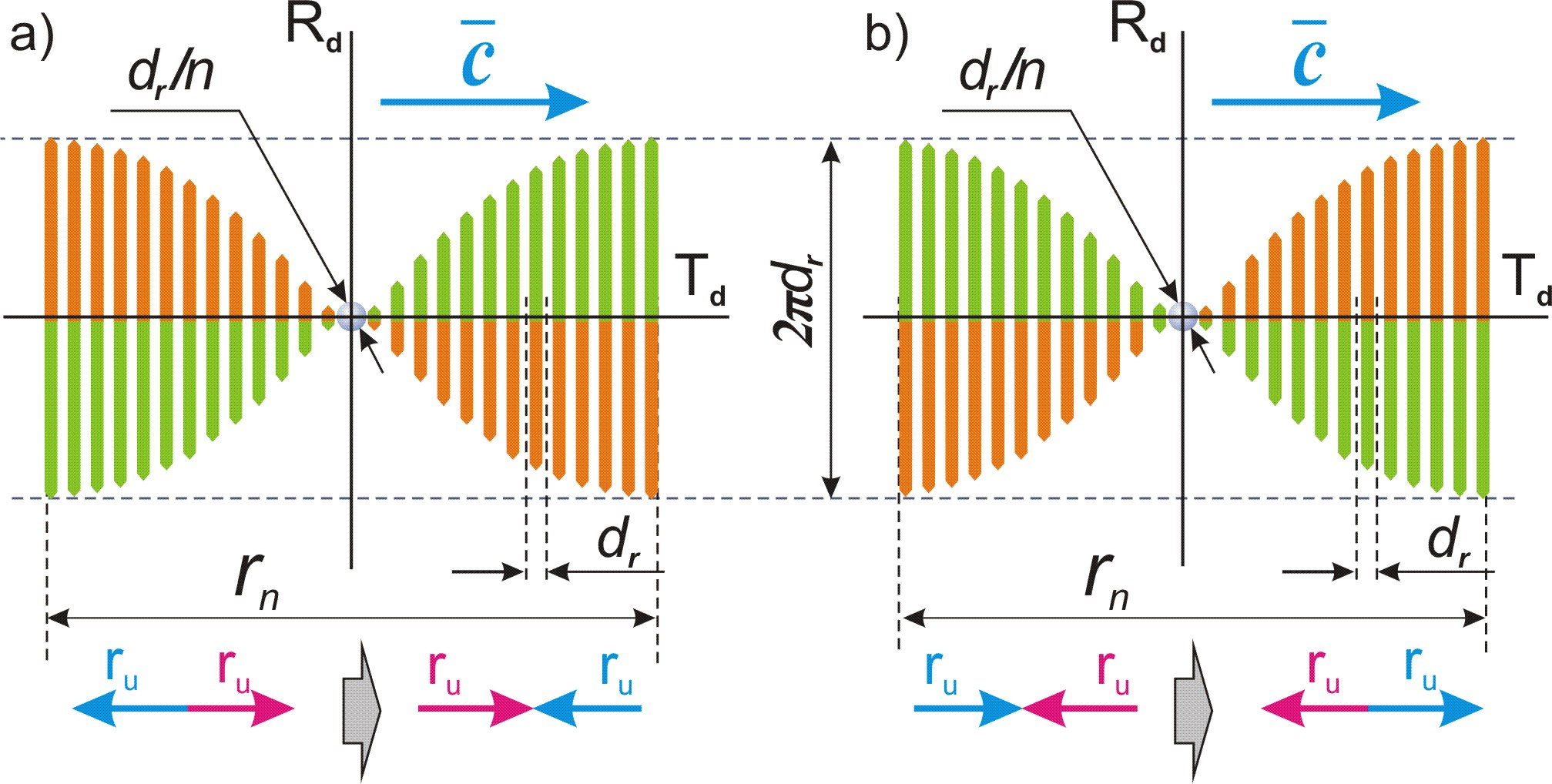Fig. 28.1.

Conclusion (c28.2):

An antineutrino differs from a photon only in that a photon represents a complete cycle of rollover of a part of physical space of size 2πdr relative to the two-dimensional structure TdRd, and an antineutrino is only the first half-cycle of this process.

It is easy to guess that the particle opposite to the antineutrino, which represents the second half-cycle of the rollover of a part of physical space with a change in the direction of the projection of the definite dimension ru from “outside to inside” to “inside to outside”, is a neutrino, fig. 28.1, b).

Conclusion (c28.3):

A neutrino is a locally singled out part of physical space, due to its roll over by a half-cycle relative to the two-dimensional structure of two indefinite dimensions Td and Rd of physical space with the change in the direction of the projection of the definite dimension ru of elementary localization from “outside to inside” to “inside to outside”.

The next cycle for neutrino should be a change in the orientation of the projection of the definite dimension ru from “inside to outside” to “outside to inside”, and for antineutrino, accordingly, from “outside to inside” to “inside to outside”, which determines the logic of their reactions with other particles.

Conclusion (c28.4):

An antineutrino can react with a proton and an electron, but cannot react with a neutron and a positron, while a neutrino, on the contrary, can react with a neutron and a positron, but cannot react with a proton and an electron.

This theoretical conclusion is in strict accordance with modern experimental data.

Similar to the photon (conclusion (c20.10)), antineutrino and neutrino must move with the maximum possible speed c along the mobile dimension Td of physical space.

Conclusion (c28.5):

Antineutrino and neutrino move with the maximum possible speed c relative to any physical frame of reference, regardless of the speed of its movement in physical space.

Thus, neutrinos and antineutrinos, like a photon, cannot have a rest mass, since they do not have a local singling out along the mainstay dimension Tu and are indefinite relative to it.

Conclusion (c28.6):

Neutrinos and antineutrinos are indefinite with respect to the mainstay dimension Tu, and the concept of rest mass in relation to them has no physical meaning.

29. Magnetic forces

Content

As a result of the decay of free neutrons, in accordance with Conclusion (c16.5), the projections of cycles of different elementary localizations overlap each other in physical space. Thus, relative to each charged particle, all other charged particles of the same sign as it are inside the projection of its cycle.

In this situation, there is a mutual breaking of symmetry by charges of the same sign relative to each other in a direction perpendicular to the direction of their movement in electrical interaction cycles.

As a consequence of this breaking of symmetry, another type of cycles of compensation for broken symmetry arises - magnetic.

Taking into account the conclusion (c16.5), this symmetry breaking take place along the projection of the indefinite dimension rd of elementary localization onto the indefinite dimension Rd of physical space.

Conclusion (c29.1):

Magnetic interaction is a consequence of the mutual breaking of symmetry by electric charges of the same sign along the projection of the indefinite dimension rd of elementary localization onto the indefinite dimension Rd of physical space.

As a result of this breaking of symmetry, a process of compensation for the broken symmetry arises, identical to the main cycle.

Conclusion (c29.2):

The force of magnetic interaction is directed along the indefinite dimension Rd of physical space.

The strength of the magnetic interaction of charges should depend on the projection of the internal cycle of a fundamental particle into physical space after its decay.

Conclusion (c29.3):

The force of magnetic interaction, similar to the force of electrical interaction, is a projection of the force of the internal cycle of elementary localization into physical space.

Let us find the conditions for this projection.

For simplicity, let us consider the option of parallel movement of charges.

The mobile dimension Td, along which the movement of charges occurs, is indefinite and closed on itself. Based on the conditions for the projection of this dimension into physical space (Conclusions (c7.21) and (в7.20)), the parallel movement of charges in one direction of physical space is carried out in opposite directions along the mobile dimension Td relative to the physical frame of reference associated with any of the two particles, fig. 29.1, a).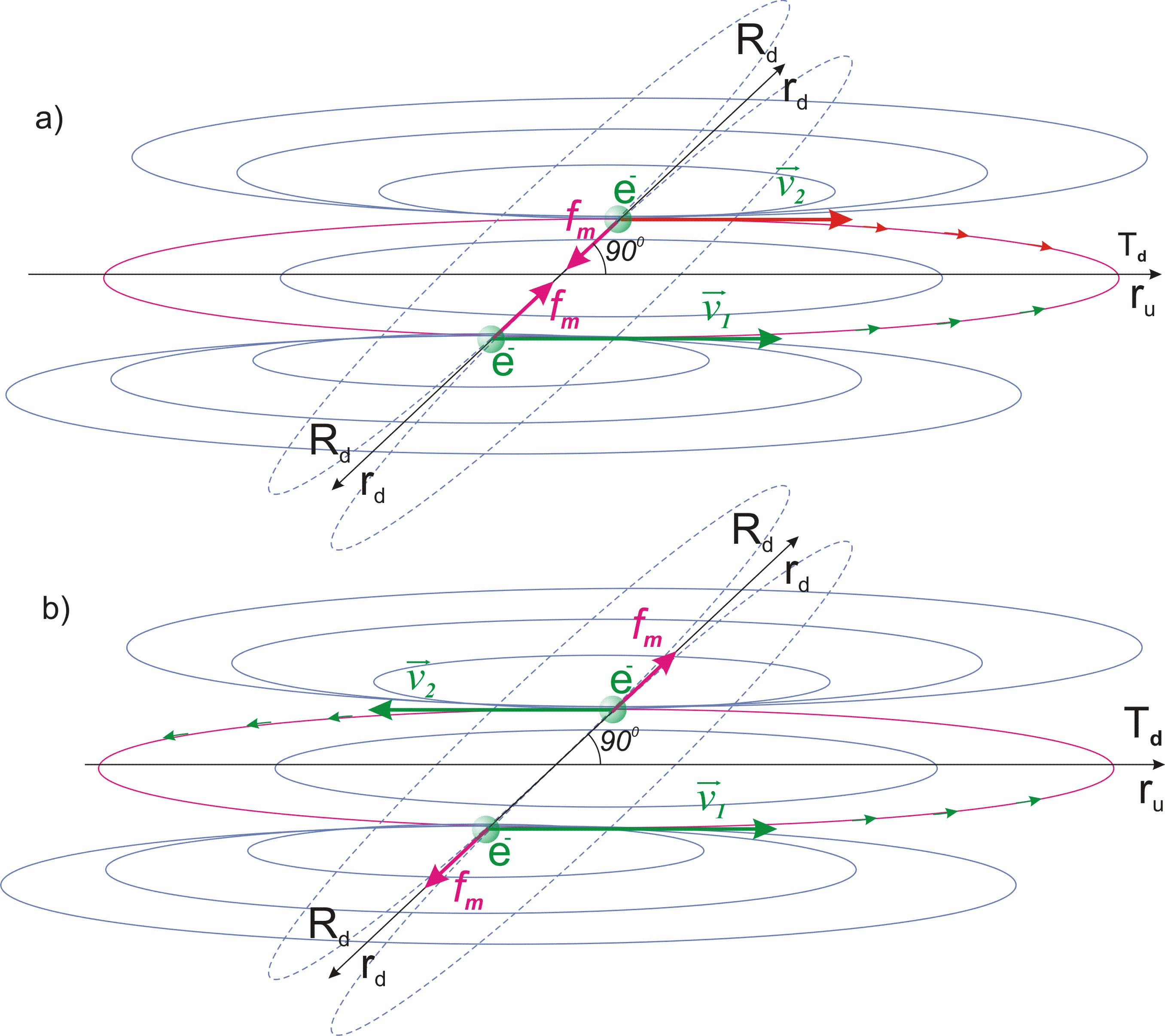Fig. 29.1.

Accordingly, movement in one direction along the mobile dimension Td corresponds to parallel movement in the physical space in opposite directions, fig. 29.1, b).

Electrons, in accordance with Conclusion (c17.7), represent the area where the boundary of the projection of the internal cycle of a fundamental particle into physical space is located. Thus, the process of compensation for the symmetry broken by them relative to each other should push them apart to the maximum distance along the indefinite dimension of physical space Rd.

Conclusion (c29.4):

The force of the magnetic interaction of negative charges, when they move parallel in opposite directions relative to physical space, is projected by the repulsive force.

Opposite to the negative charge, the positive charge represents the area where the center of the projection of the internal cycle of the fundamental particle into physical space is located. Thus, the process of compensating for broken symmetry by positive charges relative to each other should tend to move them as close as possible to the center of the frame of reference associated with them.

Conclusion (c29.5):

The force of the magnetic interaction of positive charges, when they move parallel in opposite directions relative to physical space, is projected by the force of attraction.

From the principle of symmetry, it follows that when the direction of movement of one of the charges changes to the opposite, the direction of the magnetic interaction will also change to the opposite.

Conclusion (c29.6):

The force of magnetic interaction of positive charges, when they move parallel in one direction relative to physical space, is projected by the force of repulsion, and of negative charges moving in one direction is projected by the force of attraction.

In any inertia frame of reference, the speed of movement of charges is the sum of the speed of movement of the conductors themselves and the speed of movement of electrons relative to the conductors. In relation to the movement of conductors, the magnetic forces of positive charges and negative charges balance each other by the opposite direction of its. Thus, the resulting magnetic force is determined solely by the speed of the electrons relative to the conductors.

Conclusion (c29.7):

The magnetic force acting between two conductors with electric current is determined by the speed of movement of charges relative to the conductors in any inertial frame of reference and does not depend from the speed of movement of the frame of reference or the speed of movement of the conductors.

Let us find the magnitude of the magnetic interaction force in a complete magnetic cycle, similar to a complete orbital cycle (Chapter 15, definition (d15.3)).

The cycle of elementary localization along the definite dimension ru is projected onto the mobile dimension of physical space Td. This dimension is indefinite, and in relation to each moving particle is projected as the vector of its motion. Thus, the speed of the particle in physical space relative to the local frame of reference is the only defining condition for the size of this projection.

The size of the physical space is proportional to the number of passed quanta of state nt. Accordingly, the projection of the internal cycle of a fundamental particle after its decay should be similarly determined in the quantum of state nt.

If a particle moves with speed v relative to the local frame of reference, then its displacement in the quantum of state is equal to vdt. This shift in the quantum of state nt corresponds to the size of the projection ntvdt.

Conclusion (c29.8):

The size of the projection of the internal cycle of elementary localization into the main cycle relative to a particle with a charge is defined by the speed of its movement relative to the local frame of reference and is equal to ntvdt.

The force of the internal cycle of elementary localization after the decay of a fundamental particle is projected into physical space relative to its size Rt. Thus, the force of the inner cycle must be projected into the magnetic cycle in proportion to the ratio of its size ntvdt to the size of the physical space Rt.

Taking into account formulas (6.4) and (6.5):

Rt = ntdr

c = dr/dt

ntvdt/Rt = ntvdt/ntdr = v/c (29.1)

Identically to the force of gravitational interaction, the force of magnetic interaction must be proportional to the definiteness of both interacting charges in the magnetic cycle.

Thus, for two particles in a magnetic cycle moving in parallel with speeds v1 and v2, the force of the inner cycle of elementary localization is projected in proportion to v1v22.

This projection of the force of the internal cycle of elementary localization is carried out onto the indefinite dimension Rd in accordance with Conclusion (c18.6) with the coefficient π, similar to its projection on the mobile dimension Td in the form of the force of electrical interaction. We can write, taking into account the formulas: formulas (18.7) - (18.11):

fm = fev1v22 (29.2), where fm – magnetic force acting between two charges moving in parallel.

fe = q1q2dmvc2nt3dr/πn3q02R2

fm = q1q2dmvv1v2nt3dr/πn3q02R2 (29.3)

In the case of non-parallel motion of charges, the process of their interaction should be considered in the frame of reference associated with this process. In this case, the direction of the indefinite dimension of physical space Rd is projected onto the line connecting the charges, and the mobile dimension Td is projected perpendicular to it. Thus, the movement of charges in relation to the magnetic interaction represents a projection of their movement onto the mobile dimension Td of the frame of reference, fig. 29.2.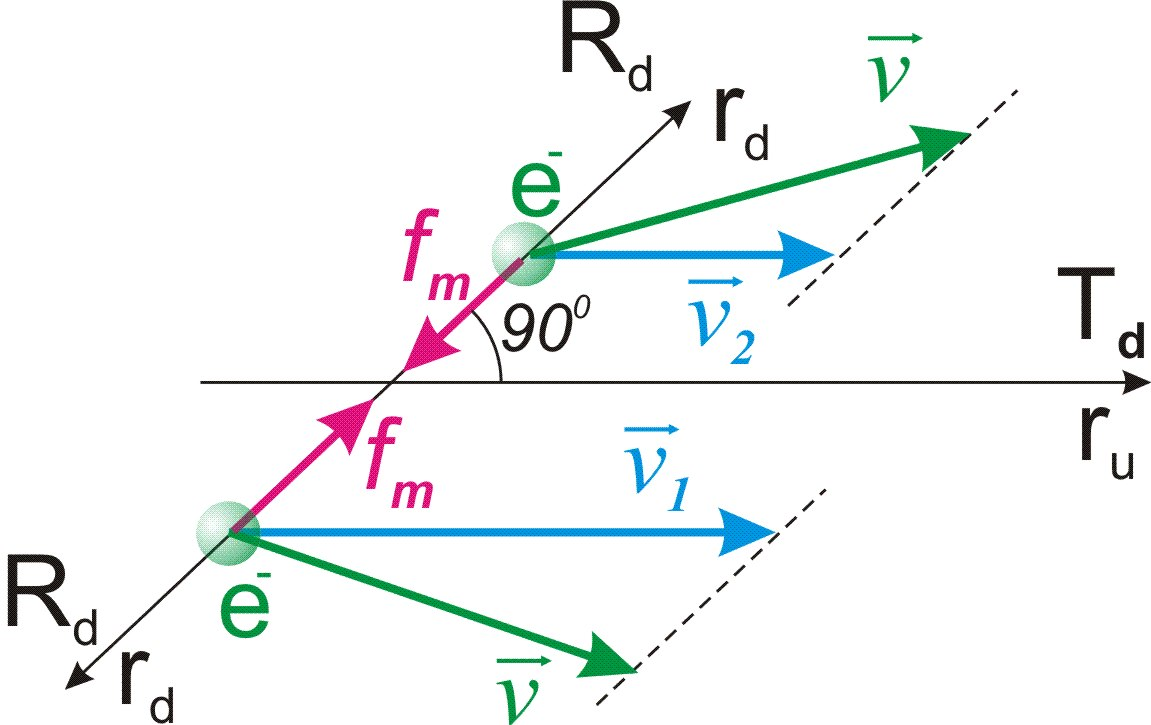Fig. 29.2.

Conclusion (c29.9):

The magnetic force acting between two charges of the same name is determined by the projections of their motion in the perpendicular direction to the line connecting them, onto which the force vector is projected.

Let us use the formula (18.12) we obtained for the electric constant:

Q = dmvc2nt3dr/πn3q02

fm = q1q2v1v2Q/с2R2 (29.4)

This formula fully corresponds to the formula obtained experimentally:

fm = µ0q1q2v1v2/4πR2

µ0 = 4πQ/с2 = 4π•10-7N/A2

In a frame of reference associated with a closed loop, the movement of a charge along it is a movement in one direction along the closed mobile dimension Td. Accordingly, the indefinite dimension Rd is projected perpendicular to it, as shown in fig. 29.3.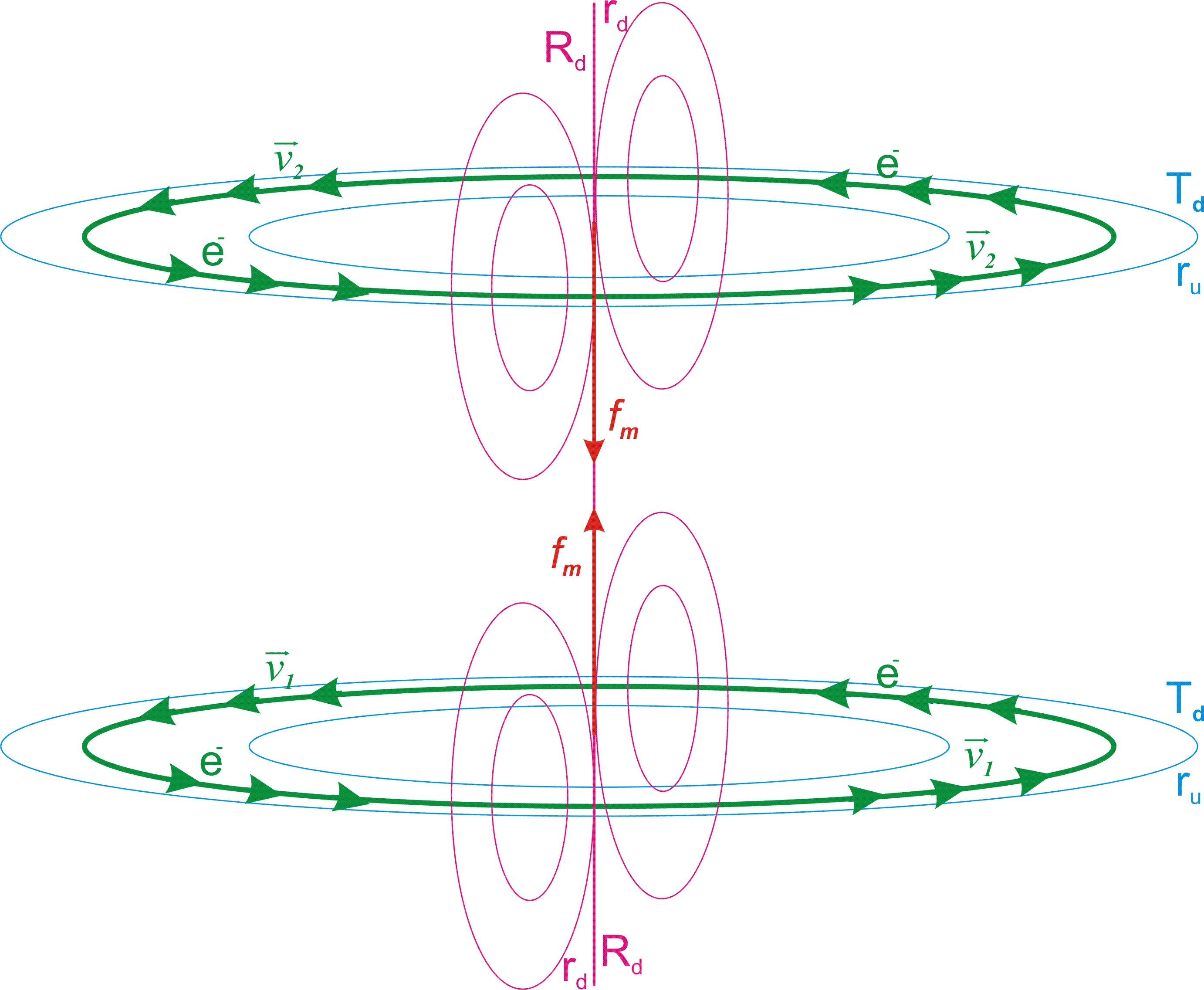Fig. 29.3.

This process creates a permanent magnet condition, which is realized when a similar magnet approaches.

30. Magnetic properties of atom and electron

Content

In accordance with Conclusion (c9.5), the mobile dimension Td is projected relative to the electron by its motion vector. But this applies only to the electron as an elementary particle in the relativity of physical space, but not to its internal space.

In the internal space of the electron, the condition of indefiniteness and closeness of the mobile dimension Td is superimposed on the condition of indefiniteness of this internal space relative to the physical space (conclusion (c8.11)).

Conclusion (c30.1):

The mobile dimension Td is projected into the internal space of an electron as a closed circle of indefinite size.

In accordance with the Conclusion (c17.10), the definite dimension ru of the potential part of elementary localization at the moment of decay of a fundamental particle is projected into the internal space of an electron by its definite dimension in the unfolded projection of its two directions.

In accordance with these conditions, the mutual vibration motion of an electron and a proton under conditions of indefiniteness of the internal space of the electron is reflected simultaneously by the circular motion along the mobile dimension Td and a rectilinear motion along the projection of the definite dimension ru.

After displacement along the half-circle, fig. 30.1, a), the projection of the definite dimension ru changes its direction, and the movement continues along the next half-circle, fig. 30.1, b). It is important to note that this “circular motion” is not such in the geometric sense corresponding to circular motion in physical space, but according to the physical conditions of motion it corresponds to it and is identical to motion in the orbital cycle (Chapter 15, definition (d15.2)).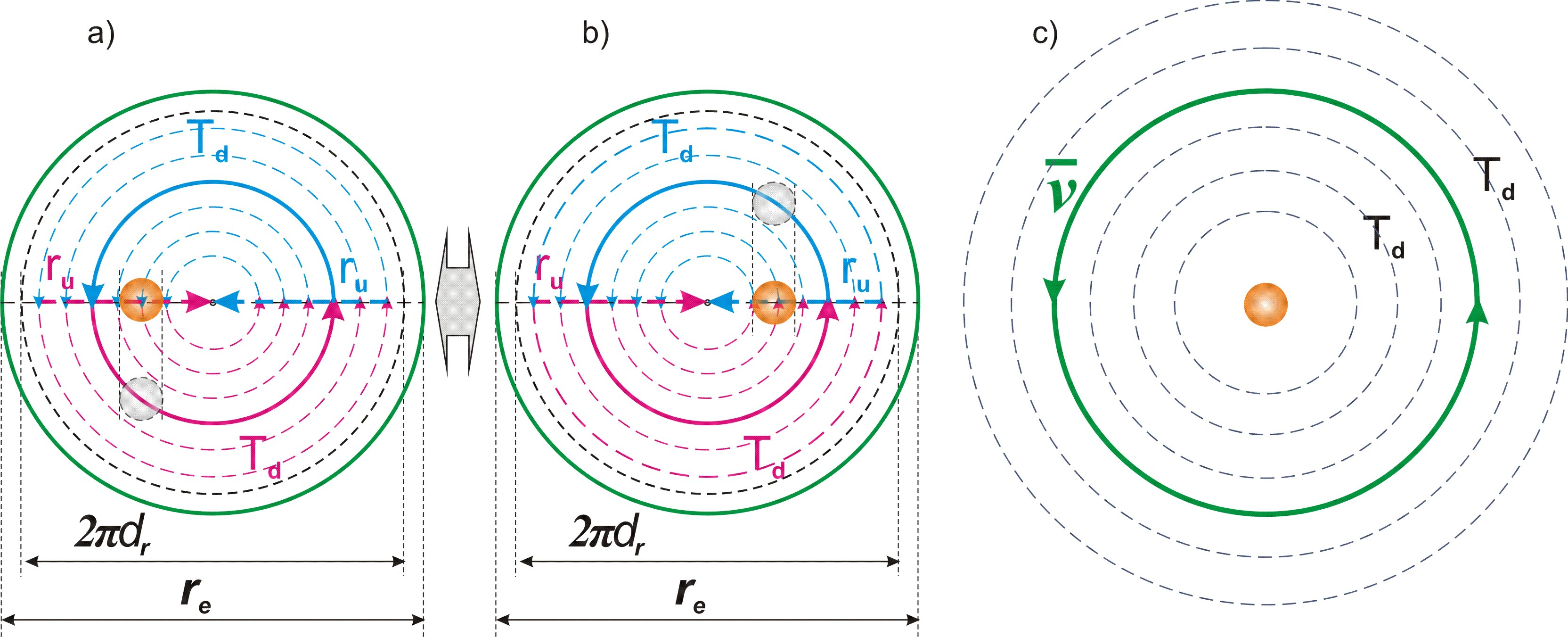Fig. 30.1.

Thus, in the relativity of the physical space and a proton, as part of the material part of the main localization, this mutual oscillatory motion of an electron and proton under conditions of indefiniteness in the internal space of the electron is reflected by the rotation of the area of presence of the electron relative to the physical space along the mobile dimension Td, fig. 30.1, c).

Conclusion (c30.2):

The mutual vibration motion of an electron and a proton under conditions of indefiniteness in the internal space of the electron is reflected by the rotation of the area of presence of the electron relative to the physical space along the mobile dimension Td.

This rotational motion of the area of presence of an electron along the mobile dimension Td is completely identical to the movement of electrons along a closed loop in the relativity of magnetic forces.

Conclusion (c30.3):

Atoms have magnetic properties due to the rotation of the area of presence of electron relative to proton in their nuclei.

In accordance with Conclusion (c16.1) and formula (16.4), until the moment of decay of a free neutron, the movement of the local frame of reference with speed vg along the indefinite dimension Td0 of the potential part of the main localization is projected by the movement of its physical body with speed vp along the indefinite dimension rd of its potential parts.

This movement occurs in the area of indefiniteness of physical space corresponding to the area of presence of a neutron.

At the moment the physical body leaves the projection of the potential part of elementary localization, additional conditions of movement in physical space are imposed on it.

Since, in accordance with the Conclusion (c7.16), physical space moves together with the local frame of reference along the dimension Td0 with speed vg, this movement cannot affect the movement in physical space of both the proton and the electron. On the other hand, this movement must take place along the mobile dimension Td. Taking into account these conditions and the Conclusion (c30.1), we come to the conclusion:

Conclusion (c30.4):

The motion of the local frame of reference relative to the fundamental frame of reference is projected by the closed motion of the area of presence of the electron with speed vp relative to physical space along the mobile dimension Td.

Due to the indefiniteness of the internal space of an electron, this motion is not projected by motion in physical space.

Thus, with respect to the projection of the internal cycle of elementary localization into physical space, an electron is in a state of motion along the mobile dimension Td even at the state of rest relative to the local frame of reference.

Conclusion (c30.5):

Due to the motion of the local frame of reference associated with the Earth, an electron at the state of rest has magnetic properties.

The speed vp of this internal motion of an electron is defined in accordance with formula (16.4):

vp = vgnt/n2

Taking into account the previously found values (22.1), (23.3) and the Conclusion (c21.1) we find the value of this speed:

vp = vgnt/n2 = 2.77876•10−34 m/s (30.1)

The closed motion of the internal space of the area of presence of the electron along the mobile dimension Td determines its position relative to the two-dimensional structure of the physical space RuTd, which is projected by the plane, fig. 30.2.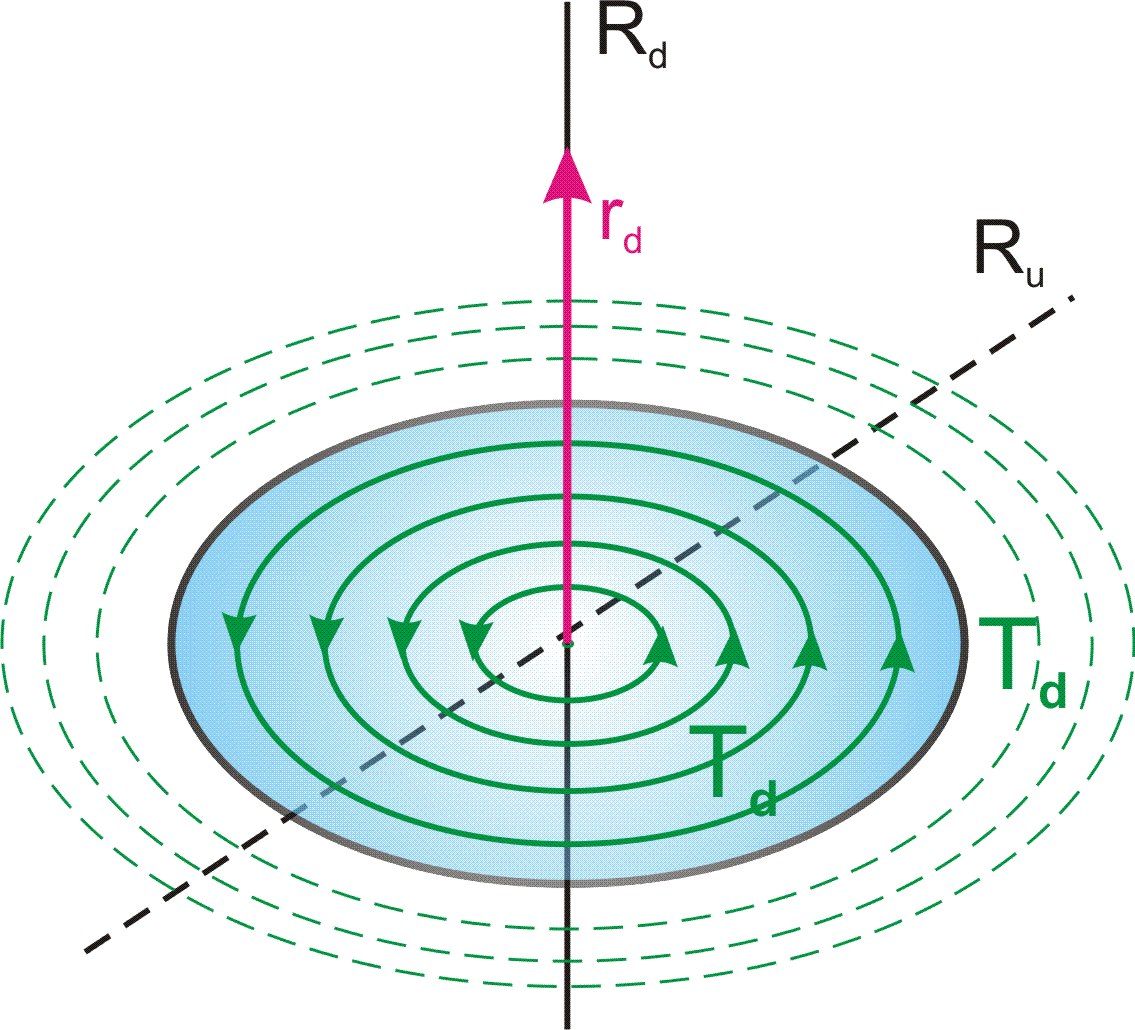Fig. 30.2.

Due to this definiteness of the position of the RuTd plane relative to the electron, the indefinite dimension Rd of physical space is projected perpendicular to it and defines the direction of the magnetic force.

This property of an electron corresponds to the concept of spin.

Conclusion (c30.6):

The spin of an electron is the direction of the projection of the indefinite dimension rd of the potential part of elementary localization onto the indefinite dimension Rd of the physical space relative to the plane RuTd of its two other dimensions, defined by the movement of the internal space of the electron relative to the physical space.

31. Electromagnetic induction

Content

Taking into account the indefiniteness of the mobile dimension Td (conclusion (c5.9)), the only condition that defines the difference between charges of the same sign is their mutual relative motion.

Thus, the invariance of the relative speeds of the mutual motion of charges is a condition for the inertiality of their movement, similar to the invariance of the relative directions of the mainstay dimension Tu in relation to elementary particles with a rest mass is a condition for the inertiality of their movement in physical space (conclusion (c10.3), definition (d10.1)).

Conclusion (c31.1):

The constancy of the relative speeds of the mutual motion of charges is the condition for the inertiality of their movement relative to the projection of the internal cycle of elementary localization into physical space.

When one charge is exposed to a certain force, its speed relative to other charges of the same name begins to change. As a result, a force arises that tends to maintain the condition of inertiality and accordingly change the speed of movement of the charges.

Conclusion (c31.2):

Magnetic induction is a force that compensates for the violation of the state of inertial movement of charges that occurs as a result of the application of force to one or more charges and tends to maintain the state of their inertiality.

The magnitude of this force depends on the relative position of the charges along the indefinite dimension Rd of the physical space onto which the indefinite dimension rd of elementary localization is projected.

Conclusion (c31.3):

The direction of magnetic induction is a projection of the indefinite dimension rd of elementary localization onto the indefinite dimension Rd of physical space in the relativity of the frame of reference associated with the electromagnetic process.

32. Nuclear forces

Content

When superimposing in the physical space the area of presence of a proton with the area of presence of a neutron, additional conditions of the projection of the internal space of the neutron are imposed on the physical body of the proton, as a projection of cycle of elementary localization.

The direction of the projection of the definite dimension ru of the inner cycle of elementary localization with respect of the proton and neutron is opposite. Accordingly, inside the area of presence of the neutron between the physical bodies of the proton and neutron, a force equal to the force of electrical attraction begins to act, directing them to each other.

The approach of the physical bodies of the proton and neutron occurs according to the conditions of the physical space, in accordance with the Conclusion (c9.5), along the mobile dimension Td. The inner space of the neutron, as a fundamental particle, is indefinite in relation to the physical space conclusion (c8.8), and its conditions, such as the stage of the cycle, do not prevent this approaching.

As a result, the physical bodies of the proton and neutron approach so much that they begin to superimpose in one area of physical space.

Prior to the superimposition of the area of presence of the proton on the area of presence of the neutron, their physical bodies were determined by their sizes of presence which is rt for the neutron and rtp for the proton.

As the physical bodies approach and superimpose, the size of area of presence of the proton is reduced in relation to the neutron along the mobile dimension Td from the size rtp to the size of the physical body rp, fig. 32.1.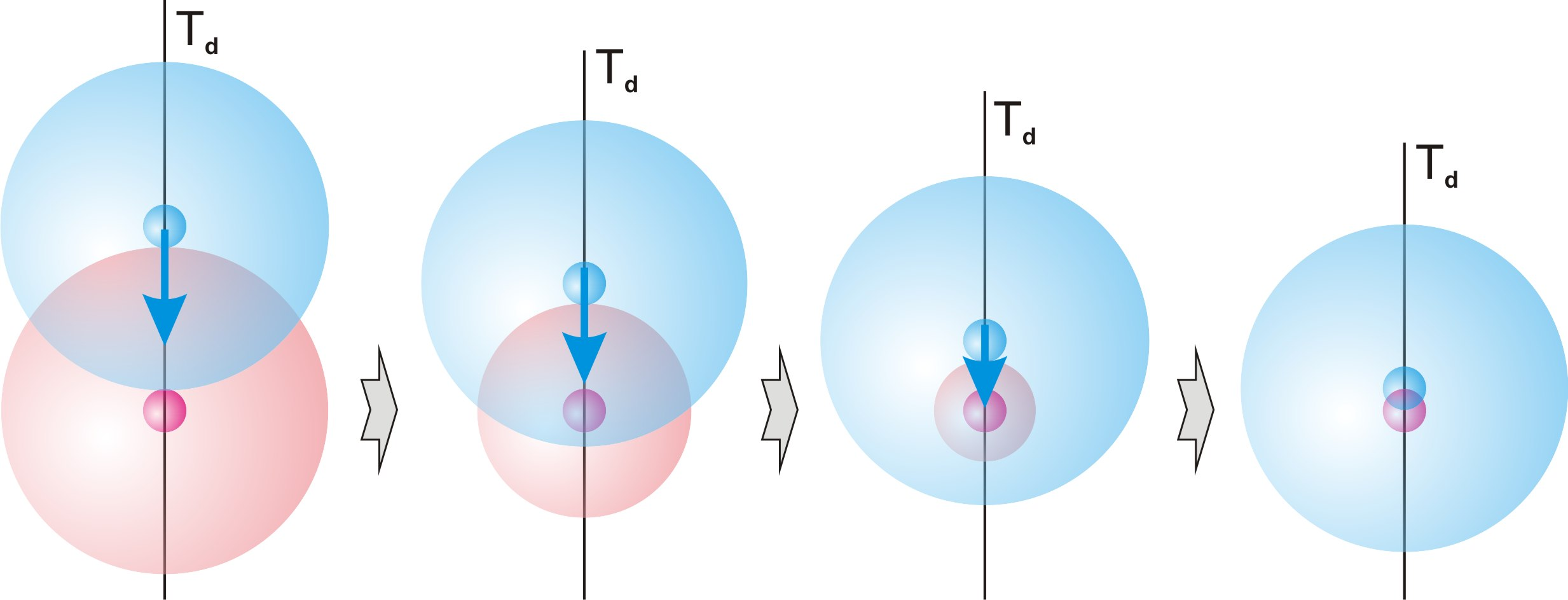Fig. 32.1.

Accordingly, its definiteness in relation to the physical body of the neutron along the mobile dimension Td increases by the ratio of its size of presence rtp to the size of its physical body rp. In accordance with formula (8.2) and the found value (23.4):

rtp/rp ≈ rt/rp = n/nt = 275.1 (32.1)

This increase in definiteness (definition (d13.2)) leads to a proportional increase in the size of the symmetry disturbance of one physical body relative to another, which leads to the proportional increase in the force of their attraction.

Conclusion (c32.1):

The force of interaction between a proton and a neutron in a deuterium nucleus is in n/nt = 275.1 times greater than the force of the electrical interaction of two unit charges at a similar distance.

From the condition that each of the two physical bodies, of the proton and of the neutron, represents for each other the area of presence of boundaries of their cycles, it necessarily follows that the size of the area of mutual overlap of the physical bodies of the proton and neutron cannot exceed the size rp of the definite dimension of each of them.

Both physical bodies overlap each other simultaneously on two sides of their two-dimensional structure rurd. From this condition it necessarily follows that the size of the overlap along the definite dimension ru should be calculate in total in its two directions, fig. 32.2.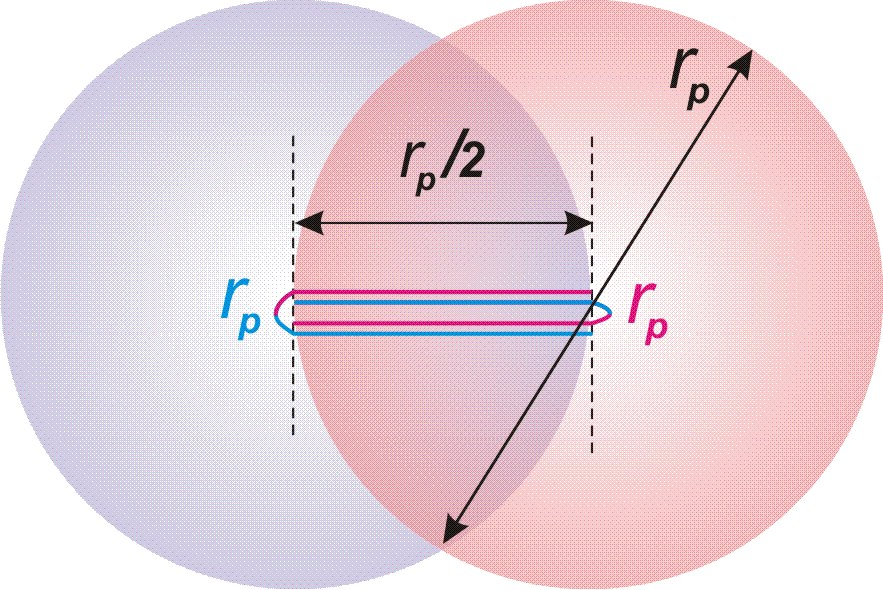Fig. 32.2.

Thus, the mutual superposition of the physical bodies of the proton and neutron in physical space is possible only up to half the size of each of them.

Their further rapprochement is impossible, since this requires a reversal of the direction of the projection of the internal definite dimension ru of one of them. Since physical bodies are part of the material part of the main localization, such a reversal separately from it is impossible.

Conclusion (c32.2):

The overlap of the internal space of the physical bodies of the neutron and proton cannot occur more than until the mutual achievement of half the size of each of them.

This process of superimposition of the physical bodies of a neutron and proton does not affect the process of motion of the physical body of the neutron along the internal indefinite dimension rd of its localization, caused by the motion of the local frame of reference associated with the Earth.

The physical body of the neutron, just as during the decay of a free neutron, uniformly accelerates along the mobile dimension Td relative to its internal space. The physical body of the proton coupled with it also moves with it.

As a result, after passing a distance equal to the size πdr of the indefinite dimension rd of elementary localization, the physical body of the neutron leaves the projection of its internal space, and the direction of the projection of the definite dimension ru in relation to it changes to the opposite. The direction of the projection of the definite dimension ru in relation to the physical body of the proton linked to it is simultaneously reversed.

Thus, the physical body of the proton occupies the vacant place in the fundamental particle and, as the physical body of the neutron, gets the opportunity to move along the mobile dimension Td in the area of indefiniteness of its internal space for another distance πdr.

Conclusion (c32.3):

The physical bodies of the neutron and proton are in constant closed motion along the mobile dimension Td in the area of indefiniteness that the neutron represents in physical space, and after each passage the distances πdr replace each other with the rollover of the projection of the definite dimension ru of the potential part of elementary localization relative to each of them.

Thus, a stable, non-decaying, state of proton-neutron conjunction arises.

Conclusion (c32.4):

A neutron in connection with a proton represents a dynamically stable state.

It is important to note that in this process of roll over the projection of the direction of dimension ru in relation to both physical bodies, there is no rollover of part of the physical space with the release of neutrinos and antineutrinos. The entire process occurs under conditions of indefiniteness of the quantum of state of the process (Definition (d12.3) and Conclusion (c12.2)). Thus, in relation to physical space, the periodic change in the direction of dimension ru in relation to both physical bodies is in indefiniteness.

The size of presence rtd of deuterium nucleus, as the connection of the physical bodies of the neutron and proton, increases relative to the size of presence rt of the neutron by going beyond its limits the attached physical body of the proton by half its size rp, fig. 32.3.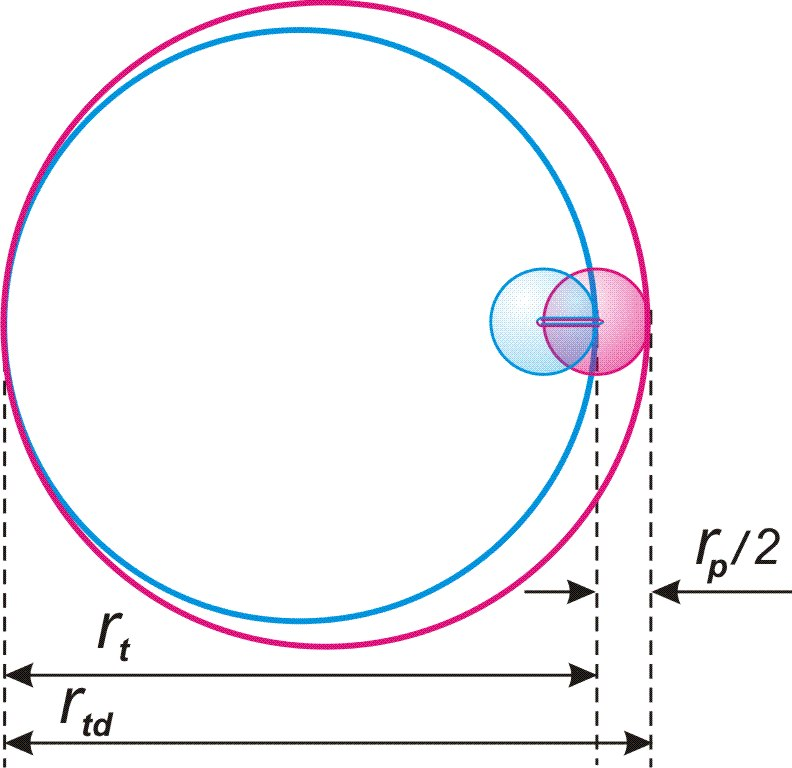Fig. 32.3.

rtd = rt + rp/2 (32.2), where rtd – the size of presence of the connection of the physical bodies of the proton and neutron.

According to the condition of the connection of both physical bodies, this size of presence will be equally projecting relative to each of them.

In accordance with this change in the size of presence, the definiteness of each of them along the mobile dimension Td and, accordingly, the mass will change proportionally.

Let us find the mass of the deuterium nucleus as the sum of two baryons, the mass of each of them is determined by the size of presence rtd of the connection of their physical bodies, according to the formula (13.10):

md = 2dmvrt/rtd = 2dmvrt/(rt + rp/2) = 2dmv/(1 + nt/2n) (32.3) , where md – the mass of the deuterium nucleus.

md = 3.34375•10-27 kg (32.4)

Experimentally known mass of deuterium nucleus - 3.343 583 20(17)•10-27 kg.

Discrepancy of only 0.005%.

Sufficient accuracy of coincidence of the calculated and experimental masses of the deuterium nucleus leaves no doubt about the correctness of the theory.

Let us find the value of the force of interaction between a proton and a neutron in a deuterium nucleus at a distance rp, using the formula (18.10) we have already found for the electrical interaction of two charges:

fe0 = dmvc2nt3dr/πn3R2

fnp= (n/nt)fe0 = (n/nt)dmvc2nt3dr/πn3rp2 = dmvc2nt2dr/πn2rp2 (32.5), where fnp – the force of nuclear interaction in the nucleus of deuterium.

Let us apply formula (8.3):

rp = nt2dr/n2

fnp = dmvc2nt2dr/πn2rp2 = dmvc2n2/nt2drπ (32.6)

We calculate the exact value of this force using the tabular and found values (в21.1),(23.3),(24.1):

dmv = 1.674927351(74)•10-27 kg

c = 299 792 458 m/s

n = 2128

nt = 1.23690•1036

dr = 1.0025089•10-10 m

fnp = dmvc2n2/nt2drπ = 36 175.0 N (32.7)

33. Quarks

Content

Modern physics considers baryons (protons and neutrons) as consisting of three subatomic particles — quarks. In experiments, quarks manifest themselves exclusively in a very small region and a very short period of time, and it is impossible to separate them into independent existence.

Unlike the classical view of physics on the dimensions of space, solely as structural characteristics that are possible for measurement, this theory considers the dimensions of space as parts of the localization of the Universe, identical to any other parts of it, which are singled out material objects. This means that within the quantum of state of the process (definition (d12.3)), in accordance with the Conclusion (c12.2), within certain frames of the experiment, it is possible to fix the projection of an elementary particle on any of the dimensions of the physical space, as an independent physical object.

Conclusion (c33.1):

Within the quantum of state of the process, there is the possibility of dividing the process of measuring an elementary particle into three separate stages for each of the dimensions of physical space, perceived as independent material particles.

Alike process occurs during the experimental detection of three quarks that make up a baryon.

The indefinite and the definite properties of dimensions define two types of quarks.

The neutron, as an identical part of the material part of the main localization, is defined in the physical space by its three dimensions (chapter 7, conclusion (c7.11)): one definite dimension Ru and two indefinite dimensions Td and Rd (udd).

In relativity of proton, the mobile dimension Td, due to the projection onto it of the definite dimension ru of the potential part of elementary localization (conclusion (c16.5)), acquires the properties of a definite dimension. Accordingly, the proton is defined in physical space by two definite and one indefinite dimensions (uud).

Conclusion (c33.2):

Quarks are a projection of baryon properties onto definite and indefinite dimensions.

Conclusion (c33.3):

The quark (u) reflects the properties of the definite dimension of localization.

The quark (d) reflects the properties of the indefinite dimension of localization.

----------------------------------------
﻿
 ««ПУБЛИКАЦИИ««

 Ваше имя:     Н А П И Ш И Т Е     В А Ш     К О М М Е Н Т А Р И Й : Если Вы не робот, Напишите название месяца следующего после осени без мягкого знака.:

 К О М М Е Н Т А Р И И

 Страница 1 из 1Страница 1 из 1Сейчас посетителей в разделе : 0 .ya-page_js_yes .ya-site-form_inited_no { display: none; }